# US8947273B2 - Entropy coding - Google Patents

## Info

Publication number
US8947273B2
US8947273B2 US14/230,183 US201414230183A US8947273B2 US 8947273 B2 US8947273 B2 US 8947273B2 US 201414230183 A US201414230183 A US 201414230183A US 8947273 B2 US8947273 B2 US 8947273B2
Authority
US
United States
Prior art keywords
entropy
symbols
symbol
buffer
bin
Prior art date
Legal status (The legal status is an assumption and is not a legal conclusion. Google has not performed a legal analysis and makes no representation as to the accuracy of the status listed.)
Active
Application number
US14/230,183
Other versions
US20140210652A1 (en
Inventor
Christian BARTNIK
Benjamin BROSS
Valeri GEORGE
Anastasia HENKEL
Tobias Hinz
Heiner Kirchhoffer
Detlev Marpe
Tung Nguyen
Matthias PREISS
Thomas Schierl
Heiko Schwarz
Mischa SIEKMANN
Jan STEGEMANN
Thomas Wiegand
Current Assignee (The listed assignees may be inaccurate. Google has not performed a legal analysis and makes no representation or warranty as to the accuracy of the list.)
Fraunhofer Gesellschaft zur Forderung der Angewandten Forschung eV
Original Assignee
Fraunhofer Gesellschaft zur Forderung der Angewandten Forschung eV
Priority date (The priority date is an assumption and is not a legal conclusion. Google has not performed a legal analysis and makes no representation as to the accuracy of the date listed.)
Filing date
Publication date
Priority to US201161543942P priority Critical
Priority to PCT/EP2012/069894 priority patent/WO2013050612A1/en
Application filed by Fraunhofer Gesellschaft zur Forderung der Angewandten Forschung eV filed Critical Fraunhofer Gesellschaft zur Forderung der Angewandten Forschung eV
Priority to US14/230,183 priority patent/US8947273B2/en
Publication of US20140210652A1 publication Critical patent/US20140210652A1/en
Assigned to FRAUNHOFER-GESELLSCHAFT ZUR FOERDERUNG DER ANGEWANDTEN FORSCHUNG E.V. reassignment FRAUNHOFER-GESELLSCHAFT ZUR FOERDERUNG DER ANGEWANDTEN FORSCHUNG E.V. ASSIGNMENT OF ASSIGNORS INTEREST (SEE DOCUMENT FOR DETAILS). Assignors: SCHIERL, THOMAS, SCHWARZ, HEIKO, BROSS, BENJAMIN, BARTNIK, CHRISTIAN, HINZ, TOBIAS, KIRCHHOFFER, HEINER, SIEKMANN, MISCHA, NGUYEN, TUNG, PREISS, Matthias, GEORGE, Valeri, HENKEL, ANASTASIA, MARPE, DETLEV, WIEGAND, THOMAS, STEGEMANN, JAN
Publication of US8947273B2 publication Critical patent/US8947273B2/en
Application granted granted Critical
Active legal-status Critical Current
Anticipated expiration legal-status Critical

• 239000000872 buffers Substances 0.000 claims description 831
• 230000000875 corresponding Effects 0.000 claims description 150
• 230000001960 triggered Effects 0.000 claims description 34
• 238000004590 computer program Methods 0.000 claims description 14
• 230000003139 buffering Effects 0.000 claims description 12
• 238000000034 methods Methods 0.000 description 132
• 241000023320 Luma <angiosperm> Species 0.000 description 109
• 238000010586 diagrams Methods 0.000 description 41
• 238000005192 partition Methods 0.000 description 39
• 230000003044 adaptive Effects 0.000 description 25
• 239000002158 endotoxins Substances 0.000 description 24
• 238000007906 compression Methods 0.000 description 22
• 230000001419 dependent Effects 0.000 description 22
• 238000004422 calculation algorithm Methods 0.000 description 21
• 101710020325 cbp-4 Proteins 0.000 description 20
• 230000001131 transforming Effects 0.000 description 18
• 239000000203 mixtures Substances 0.000 description 16
• 238000000638 solvent extraction Methods 0.000 description 16
• 230000004301 light adaptation Effects 0.000 description 15
• 230000011664 signaling Effects 0.000 description 13
• 230000004044 response Effects 0.000 description 12
• 230000005540 biological transmission Effects 0.000 description 9
• 280000651184 Binary Tree companies 0.000 description 8
• 230000037242 Cmax Effects 0.000 description 7
• 230000003068 static Effects 0.000 description 7
• 230000002123 temporal effects Effects 0.000 description 7
• 101710010199 csd Proteins 0.000 description 5
• 101710034595 sufS Proteins 0.000 description 5
• 239000000969 carriers Substances 0.000 description 4
• 241001522296 Erithacus rubecula Species 0.000 description 3
• 102000002067 Protein Subunits Human genes 0.000 description 3
• 108010001267 Protein Subunits Proteins 0.000 description 3
• 238000006243 chemical reactions Methods 0.000 description 3
• 230000000694 effects Effects 0.000 description 3
• 230000004048 modification Effects 0.000 description 3
• 238000006011 modification reactions Methods 0.000 description 3
• 239000011433 polymer cement mortar Substances 0.000 description 3
• 238000000926 separation method Methods 0.000 description 3
• 230000004075 alteration Effects 0.000 description 2
• 238000004364 calculation methods Methods 0.000 description 2
• 238000004891 communication Methods 0.000 description 2
• 230000001276 controlling effects Effects 0.000 description 2
• 230000003111 delayed Effects 0.000 description 2
• 238000009795 derivation Methods 0.000 description 2
• 238000000605 extraction Methods 0.000 description 2
• 238000001914 filtration Methods 0.000 description 2
• 239000000463 materials Substances 0.000 description 2
• 239000011159 matrix materials Substances 0.000 description 2
• 230000000630 rising Effects 0.000 description 2
• 241000212893 Chelon labrosus Species 0.000 description 1
• 241001025261 Neoraja caerulea Species 0.000 description 1
• 280000644727 Special Events companies 0.000 description 1
• 230000002547 anomalous Effects 0.000 description 1
• 230000006399 behavior Effects 0.000 description 1
• 239000012141 concentrates Substances 0.000 description 1
• 230000003750 conditioning Effects 0.000 description 1
• 238000010276 construction Methods 0.000 description 1
• 230000001934 delay Effects 0.000 description 1
• 230000002349 favourable Effects 0.000 description 1
• 239000011133 lead Substances 0.000 description 1
• 235000021190 leftovers Nutrition 0.000 description 1
• 238000005457 optimization Methods 0.000 description 1
• 230000000750 progressive Effects 0.000 description 1
• 230000003252 repetitive Effects 0.000 description 1
• 230000011218 segmentation Effects 0.000 description 1
• 239000007787 solids Substances 0.000 description 1
• 238000001228 spectrum Methods 0.000 description 1
• 238000010561 standard procedure Methods 0.000 description 1
• 230000001360 synchronised Effects 0.000 description 1
• 230000000007 visual effect Effects 0.000 description 1

## Images

•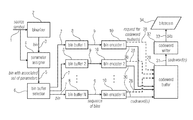••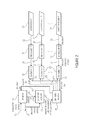•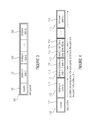•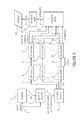•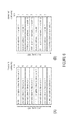•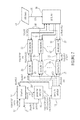•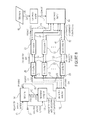•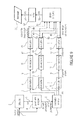•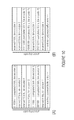•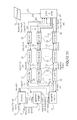•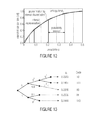•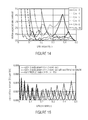•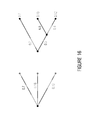•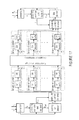•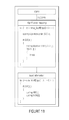•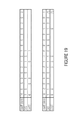•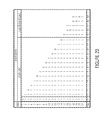•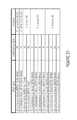•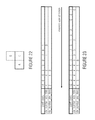•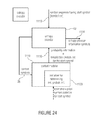•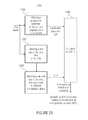•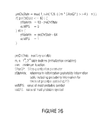•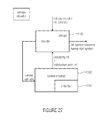•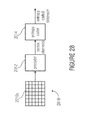•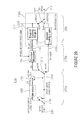•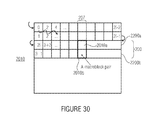•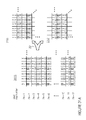•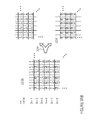•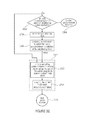•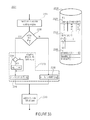•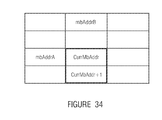•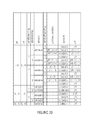•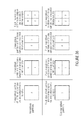•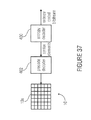•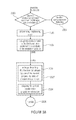•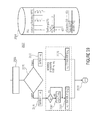•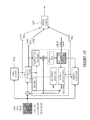•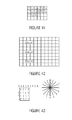•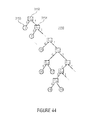•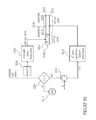•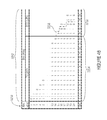•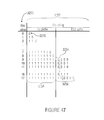•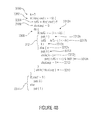•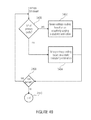•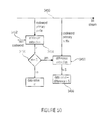•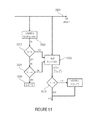•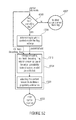•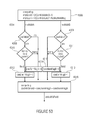•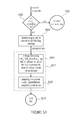••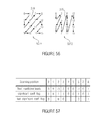•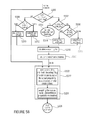•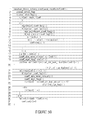•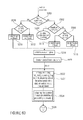••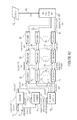•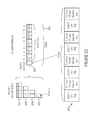•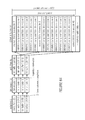•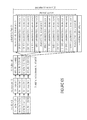•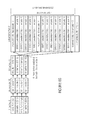•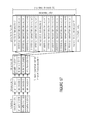•## Classifications

• HELECTRICITY
• H03BASIC ELECTRONIC CIRCUITRY
• H03MCODING; DECODING; CODE CONVERSION IN GENERAL
• H03M7/00Conversion of a code where information is represented by a given sequence or number of digits to a code where the same, similar or subset of information is represented by a different sequence or number of digits
• H03M7/30Compression; Expansion; Suppression of unnecessary data, e.g. redundancy reduction
• H03M7/40Conversion to or from variable length codes, e.g. Shannon-Fano code, Huffman code, Morse code
• HELECTRICITY
• H03BASIC ELECTRONIC CIRCUITRY
• H03MCODING; DECODING; CODE CONVERSION IN GENERAL
• H03M7/00Conversion of a code where information is represented by a given sequence or number of digits to a code where the same, similar or subset of information is represented by a different sequence or number of digits
• H03M7/30Compression; Expansion; Suppression of unnecessary data, e.g. redundancy reduction
• H03M7/60General implementation details not specific to a particular type of compression
• H03M7/6064Selection of Compressor
• H03M7/607Selection between different types of compressors
• HELECTRICITY
• H04ELECTRIC COMMUNICATION TECHNIQUE
• H04NPICTORIAL COMMUNICATION, e.g. TELEVISION
• H04N19/00Methods or arrangements for coding, decoding, compressing or decompressing digital video signals
• H04N19/10Methods or arrangements for coding, decoding, compressing or decompressing digital video signals using adaptive coding
• H04N19/102Methods or arrangements for coding, decoding, compressing or decompressing digital video signals using adaptive coding characterised by the element, parameter or selection affected or controlled by the adaptive coding
• H04N19/13Adaptive entropy coding, e.g. adaptive variable length coding [AVLC] or context adaptive binary arithmetic coding [CABAC]
• HELECTRICITY
• H04ELECTRIC COMMUNICATION TECHNIQUE
• H04NPICTORIAL COMMUNICATION, e.g. TELEVISION
• H04N19/00Methods or arrangements for coding, decoding, compressing or decompressing digital video signals
• H04N19/10Methods or arrangements for coding, decoding, compressing or decompressing digital video signals using adaptive coding
• H04N19/134Methods or arrangements for coding, decoding, compressing or decompressing digital video signals using adaptive coding characterised by the element, parameter or criterion affecting or controlling the adaptive coding
• H04N19/156Availability of hardware or computational resources, e.g. encoding based on power-saving criteria
• HELECTRICITY
• H04ELECTRIC COMMUNICATION TECHNIQUE
• H04NPICTORIAL COMMUNICATION, e.g. TELEVISION
• H04N19/00Methods or arrangements for coding, decoding, compressing or decompressing digital video signals
• H04N19/42Methods or arrangements for coding, decoding, compressing or decompressing digital video signals characterised by implementation details or hardware specially adapted for video compression or decompression, e.g. dedicated software implementation
• H04N19/436Methods or arrangements for coding, decoding, compressing or decompressing digital video signals characterised by implementation details or hardware specially adapted for video compression or decompression, e.g. dedicated software implementation using parallelised computational arrangements
• HELECTRICITY
• H03BASIC ELECTRONIC CIRCUITRY
• H03MCODING; DECODING; CODE CONVERSION IN GENERAL
• H03M7/00Conversion of a code where information is represented by a given sequence or number of digits to a code where the same, similar or subset of information is represented by a different sequence or number of digits
• H03M7/30Compression; Expansion; Suppression of unnecessary data, e.g. redundancy reduction
• H03M7/40Conversion to or from variable length codes, e.g. Shannon-Fano code, Huffman code, Morse code
• H03M7/4006Conversion to or from arithmetic code

## Abstract

An encoder for encoding a sequence of symbols is described which has an assigner configured to assign a number of parameters to each symbol of the sequence of symbols based on information contained within previous symbols of the sequence of symbols; a plurality of entropy encoders each of which is configured to convert the symbols forwarded to the respective entropy encoder into a respective bitstream; and a selector configured to forward each symbol to a selected one of the plurality of entropy encoders, the selection depending on the number of parameters assigned to the respective symbol.

## Description

CROSS-REFERENCE TO RELATED APPLICATIONS

This application is a continuation of copending International Application No. PCT/EP2012/069894, filed Oct. 8, 2012, which is incorporated herein by reference in its entirety, and additionally claims priority from U.S. Provisional Application No. 61/543,942, filed Oct. 6, 2011, which is also incorporated herein by reference in its entirety.

BACKGROUND OF THE INVENTION

The present invention relates to entropy coding and may be used in applications such as, for example, video and audio compression.

The present invention describes a new method and apparatus for entropy encoding and decoding of discrete data. Entropy coding, in general, can be considered as the most generic form of lossless data compression. Lossless compression aims to represent discrete data with fewer bits than needed for the original data representation but without any loss of information. Discrete data can be given in the form of text, graphics, images, video, audio, speech, facsimile, medical data, meteorological data, financial data, or any other form of digital data.

In entropy coding, the specific high-level characteristics of the underlying discrete data source are often neglected. Consequently, any data source is considered to be given as a sequence of source symbols that takes values in a given m-ary alphabet and that is characterized by a corresponding (discrete) probability distribution {p1, . . . , pm}. In these abstract settings, the lower bound of any entropy coding method in terms of expected codeword length in bits per symbol is given by the entropy

$H = - ∑ i = 1 m ⁢ p i ⁢ log 2 ⁢ p i . ( A1 )$
Huffman codes and arithmetic codes are well-known examples of practical codes capable of approximating the entropy limit (in a certain sense). For a fixed probability distribution, Huffman codes are relatively easy to construct. The most attractive property of Huffman codes is that its implementation can be efficiently realized by the use of variable-length code (VLC) tables. However, when dealing with time-varying source statistics, i.e., changing symbol probabilities, the adaptation of the Huffman code and its corresponding VLC tables is quite demanding, both in terms of algorithmic complexity as well as in terms of implementation costs. Also, in the case of having a dominant alphabet value with pk>0.5, the redundancy of the corresponding Huffman code (without using any alphabet extension such as run length coding) may be quite substantial. Another shortcoming of Huffman codes is given by the fact that in case of dealing with higher-order probability modeling, multiple sets of VLC tables may be necessitated. Arithmetic coding, on the other hand, while being substantially more complex than VLC, offers the advantage of a more consistent and adequate handling when coping with adaptive and higher-order probability modeling as well as with the case of highly skewed probability distributions. Actually, this characteristic basically results from the fact that arithmetic coding provides a mechanism, at least conceptually, to map any given value of probability estimate in a more or less direct way to a portion of the resulting codeword. Being provided with such an interface, arithmetic coding allows for a clean separation between the tasks of probability modeling and probability estimation, on the one hand, and the actual entropy coding, i.e., mapping of a symbols to codewords, on the other hand.

For the sake of clarity, we will restrict the exposition in the following to the case of a binary alphabet, although conceptually, the very basic methods of this invention also apply to the general case of an m-ary alphabet with m>2. The probability distribution of symbols with values in a binary alphabet can be described by a single parameter p=pLPS, where the useful distinction is made between the so-called less probable symbol (LPS) with probability estimates pLPS≦0.5 and the more probable symbol (MPS) with pMPS=1−pLPS. Thus, in the general case we have 0<pLPS≦0.5, where in practical cases, the probability estimate is often bounded from below by a minimum probability value pmin such that pmin≦pLPS.

A good compromise between coding efficiency on the one hand and coding complexity on the other hand offers entropy coding a symbol sequence in parallel via partial bitstreams each of which assumes responsibility of coding a respective portion of the symbol sequence which is most suitable for the respective portion such as for example in terms of symbol statistics or probability estimation as it is the case in PIPE coding (PIPE=probability interval partitioning entropy). However, the problem resulting therefrom is the necessity to interleave the partial bitstreams in case a common single bitstream is wanted.

SUMMARY

According to an embodiment, an encoder for encoding a sequence of symbols may have: an assigner configured to assign a number of parameters to each symbol of the sequence of symbols based on information contained within previous symbols of the sequence of symbols; a plurality of entropy encoders each of which is configured to convert the symbols forwarded to the respective entropy encoder into a respective bitstream; and a selector configured to forward each symbol to a selected one of the plurality of entropy encoders, the selection depending on the number of parameters assigned to the respective symbol, wherein each entropy encoder is a variable length coder configured to map symbol sequences to codewords shorter as, or as long as, a respective maximum bit length Lx, wherein the encoder has, for each entropy encoder, an individual buffer for buffering the respective bitstream of the respective entropy encoder, and a global buffer, wherein the global buffer is configured to reserve a sequence of tranche entries of respective bit length Nx for the plurality of entropy encoders in a sequential order depending on an order in which the reservations of the tranche entries for the plurality of entropy encoders are triggered; wherein the plurality of entropy encoders and the respective individual buffers cooperate to trigger a reservation of a tranche entry of bit length Nx for a respective one of the entropy encoders at times where the symbols of the sequence of symbols forwarded by the selector to the plurality of entropy encoders result in a beginning of a new symbol sequence to be mapped to a codeword at the respective entropy encoder, provided that at the respective time a number of bits currently reserved in the global buffer for the respective entropy encoder minus a number Mx of bits currently buffered in the individual buffer of the respective entropy encoder or the global buffer for the respective entropy encoder is not sufficient to accommodate an intended minimum number Cx of further codewords for the respective entropy encoder plus one, times the respective maximum bit length Lx, i.e. (Cx+1)·Lx, wherein the individual buffers of the plurality of entropy encoders are configured to output buffered portions of the bitstreams to fill the respective reserved tranche entries in the global buffer, and the global buffer is configured to remove filled tranche entries of the tranche entries in the sequential order to obtain a single stream of tranches of the bitstreams of the plurality of bitstreams, interspersed into the single stream of tranches in an interleaved manner.

According to another embodiment, a method for encoding a sequence of symbols may have the steps of: assigning a number of parameters to each symbol of the sequence of symbols based on information contained within previous symbols of the sequence of symbols; and forwarding each symbol to a selected one of a plurality of entropy encoders, the selection depending on the number of parameters assigned to the respective symbol, and each of the plurality of entropy encoders being configured to convert symbols forwarded to the respective entropy encoder into a respective bitstream, wherein each entropy encoder is a variable length coder configured to map symbol sequences to codewords shorter as, or as long as, a respective maximum bit length Lx, wherein the method further has for each entropy encoder, buffering the respective bitstream of the respective entropy encoder in a respective individual buffer, reserving a sequence of tranche entries of respective bit length Nx for the plurality of entropy encoders in a global buffer in a sequential order depending on an order in which the reservations of the tranche entries for the plurality of entropy encoders are triggered; triggering a reservation of a tranche entry of bit length Nx for a respective one of the entropy encoders at times where the symbols of the sequence of symbols forwarded by the selector to the plurality of entropy encoders result in a beginning of a new symbol sequence to be mapped to a codeword at the respective entropy encoder, provided that at the respective time a number of bits currently reserved in the global buffer for the respective entropy encoder minus a number Mx of bits currently buffered in the individual buffer of the respective entropy encoder or the global buffer for the respective entropy encoder is not sufficient to accommodate an intended minimum number Cx of further codeword for the respective entropy encoder plus one, times the respective maximum bit length Lx, i.e. (Cx+1)·Lx, outputting buffered portions of the bitstreams from the codeword buffers to fill the respective reserved tranche entries in the global buffer, and removing filled tranche entries of the tranche entries in the sequential order to obtain a single stream of tranches of the bitstreams of the plurality of bitstreams, interspersed into the single stream of tranches in an interleaved manner.

According to still another embodiment, a decoder for reconstructing a sequence of symbols may have: a plurality of entropy decoders, each of which is configured to convert a respective bitstream into symbols; an assigner configured to assign a number of parameters to each symbol of a sequence of symbols to be reconstructed based on information contained within previously reconstructed symbols of the sequence of symbols; and a selector configured to retrieve each symbol of the sequence of symbols to be reconstructed from a selected one of the plurality of entropy decoders, the selection depending on the number of parameters defined to the respective symbol, wherein each entropy decoder is a variable length decoder configured to map codewords shorter as, or as long as, a respective maximum bit length Lx, to symbol sequences, wherein the decoder further has global buffer configured to receive a single stream of tranches of the bitstreams of the plurality of bitstreams, interspersed into the single stream of tranches in an interleaved manner, wherein the global buffer is configured to sequentially distribute the tranches from the global buffer to the plurality of entropy decoders depending on an order in which requests for the tranches occur, wherein each of the plurality of entropy decoders is configured to trigger a respective request for a tranche of respective bit length Nx of the respective bitstream from the global buffer at times where, among the symbols of the sequence of symbols to be reconstructed as retrieved by the selector from the plurality of entropy decoders, a symbol is encountered which forms a beginning of a new symbol sequence to be mapped from a new codeword within the respective bitstream by the respective entropy decoder, provided that at the respective time a number Mx of bits of the respective bitstream having been distributed to the respective entropy decoder by the global buffer, but not yet having been entropy decoded by the entropy decoder so far, including the new codeword, is less than an intended minimum number Cx of further codewords for the respective entropy decoder plus one, times the respective maximum bit length Lx, i.e. (Cx+1)·Lx.

According to another embodiment, a method for reconstructing a sequence of symbols may have the steps of: assigning a number of parameters to each symbol of a sequence of symbols to be reconstructed based on information contained within previously reconstructed symbols of the sequence of symbols; and retrieving each symbol of the sequence of symbols to be reconstructed from a selected one of a plurality of entropy decoders, the selection depending on the number of parameters defined to the respective symbol, and each of the plurality of entropy decoders being configured to convert a respective bitstream into symbols, wherein each entropy decoder is a variable length decoder configured to map codewords shorter as, or as long as, a respective maximum bit length Lx, to symbol sequences, wherein the method further has receiving a single stream of tranches of the bitstreams of the plurality of bitstreams, interspersed into the single stream of tranches in an interleaved manner, in a global buffer; sequentially distributing the tranches from the global buffer to the plurality of entropy decoders depending on an order in which requests for the tranches occur, for each of the plurality of entropy decoders, triggering a respective request for a tranche of respective bit length Nx of the respective bitstream from the global buffer at times where, among the symbols of the sequence of symbols to be reconstructed as retrieved by the selector from the plurality of entropy decoders, a symbol is encountered which forms a beginning of a new symbol sequence to be mapped from a new codeword within the respective bitstream by the respective entropy decoder, provided that at the respective time a number Mx of bits of the respective bitstream having been distributed to the respective entropy decoder by the global buffer, but not yet having been entropy decoded by the entropy decoder so far, including the new codeword is less than an intended minimum number Cx of further codewords for the respective entropy decoder plus one, times the respective maximum bit length Lx, i.e. (Cx+1)·Lx.

According to another embodiment, an encoder for encoding a sequence of symbols may have: an assigner configured to assign a number of parameters to each symbol of the sequence of symbols based on information contained within previous symbols of the sequence of symbols; a plurality of entropy encoders each of which is configured to convert the symbols forwarded to the respective entropy encoder into a respective bitstream; and a selector configured to forward each symbol to a selected one of the plurality of entropy encoders, the selection depending on the number of parameters assigned to the respective symbol, wherein each entropy encoder is a variable length coder configured to map symbol sequences to codewords, wherein the encoder has, for each entropy encoder, an individual buffer for buffering the respective bitstream of the respective entropy encoder, and a global buffer, wherein the global buffer is configured to reserve a sequence of tranche entries for the plurality of entropy encoders in a sequential order depending on an order in which the reservations of the tranche entries for the plurality of entropy encoders are triggered; wherein the plurality of entropy encoders and the respective individual buffers cooperate to trigger a reservation of a tranche entry of bit length Nx for a respective one of the entropy encoders at times where the symbols of the sequence of symbols forwarded by the selector to the plurality of entropy encoders result in a beginning of a new symbol sequence to be mapped to a codeword at the respective entropy encoder, provided that at the respective time a difference between a number of bits currently reserved in the global buffer for the respective entropy encoder and a number Mx of bits currently buffered in the individual buffer of the respective entropy encoder or the global buffer for the respective entropy encoder does not fulfill a predetermined criterion, wherein the individual buffers of the plurality of entropy encoders are configured to output buffered portions of the bitstreams to fill the respective reserved tranche entries in the global buffer, and the global buffer is configured to remove filled tranche entries of the tranche entries in the sequential order to obtain a single stream of tranches of the bitstreams of the plurality of bitstreams, interspersed into the single stream of tranches in an interleaved manner, wherein the individual buffers are configured to output buffered portions of the bitstreams to fill the respective reserved tranche entries in the global buffer in units of tranche entries; the global buffer is configured to, at the times of the triggering of a respective reservation by any of the plurality of entropy encoders and the respective individual buffers, finish the removal of the filled tranche entries of the tranche entries in the sequential order from the least recently reserved, already having been filled tranche entry, inclusively, if any, up to the least recently reserved, not yet filled tranche entry, exclusively, then check as to whether a number of bits in the global buffer covered by the reserved tranche entries plus a bit length Nx of the requested tranche entry for the respective reservation-triggering entropy encoder is greater than a maximum buffer delay D, and, if not reserve a respective new tranche entry of bit length Nx for the respective reservation-triggering entropy encoder, but if so, cause the entropy encoder for which the least recently reserved, not yet filled tranche entry is reserved, to extend symbols forwarded to it by the selector, which have not yet been entropy encoded, if any, to a valid symbol sequence, and map the valid symbol sequence to a codeword so as to be buffered by the corresponding individual buffer, and then check as to whether the number Mx of bits currently buffered in the individual buffer of the entropy encoder for which the least recently reserved, not yet filled tranche entry is reserved, is smaller than the bit length Nx for this entropy encoder, and if so, append Nx-Mx bits to the Mx bits currently buffered, and then output Nx buffered bits out of the individual buffer of the entropy encoder for which the least recently reserved, not yet filled tranche entry is reserved, into the least recently reserved, not yet filled tranche entry in order to fill same, with leaving potentially additionally buffered bits beyond the Nx buffered bits output, in the individual buffer.

According to still another embodiment, a decoder for reconstructing a sequence of symbols may have: a plurality of entropy decoders, each of which is configured to convert a respective bitstream into symbols; an assigner configured to assign a number of parameters to each symbol of a sequence of symbols to be reconstructed based on information contained within previously reconstructed symbols of the sequence of symbols; and a selector configured to retrieve each symbol of the sequence of symbols to be reconstructed from a selected one of the plurality of entropy decoders, the selection depending on the number of parameters defined to the respective symbol, wherein each entropy decoder is a variable length decoder configured to map codewords to symbol sequences, wherein the decoder further has global buffer configured to receive a single stream of tranches of the bitstreams of the plurality of bitstreams, interspersed into the single stream of tranches in an interleaved manner, wherein the global buffer is configured to sequentially distribute the tranches from the global buffer to the plurality of entropy decoders depending on an order in which requests for the tranches occur, wherein each of the plurality of entropy decoders is configured to trigger a respective request for a tranche of the respective bitstream from the global buffer at times where, among the symbols of the sequence of symbols to be reconstructed as retrieved by the selector from the plurality of entropy decoders, a symbol is encountered which forms a beginning of a new symbol sequence to be mapped from a new codeword within the respective bitstream by the respective entropy decoder, provided that at the respective time a number Mx of bits of the respective bitstream having been distributed to the respective entropy decoder by the global buffer, but not yet having been entropy decoded by the entropy decoder so far, including the new codeword, is less than a predetermined number, wherein the decoder is configured to log, for each entropy decoder, a number of bits distributed from the global buffer to the plurality of entropy decoders up to the distribution of a least recently distributed tranche distributed from the global buffer to the respective entropy decoder, determine, each time a respective request for a tranche of a respective bit length Nx is triggered, as to whether a difference between a global number of bits distributed from the global buffer to the plurality of entropy decoders up to the respective request on the one hand, and a minimum logged number of bits on the other hand, is greater than a maximum buffer delay D, and, if so, discard the least recently distributed tranche distributed from the global buffer to the entropy decoder for which the minimum logged number has been logged, and any symbols not yet having been retrieved from the entropy decoder for which the minimum logged number has been logged by the selector.

According to another embodiment, an encoder for encoding a sequence of symbols may have: an assigner configured to assign a number of parameters to each symbol of the sequence of symbols based on information contained within previous symbols of the sequence of symbols; a plurality of entropy encoders each of which is configured to convert the symbols forwarded to the respective entropy encoder into a respective bitstream; and a selector configured to forward each symbol to a selected one of the plurality of entropy encoders, the selection depending on the number of parameters assigned to the respective symbol, wherein each entropy encoder is a variable length coder configured to map symbol sequences to codewords, wherein the encoder has, for each entropy encoder, an individual buffer for buffering the respective bitstream of the respective entropy encoder, and a global buffer, wherein the global buffer is configured to reserve a sequence of tranche entries for the plurality of entropy encoders in a sequential order depending on an order in which the reservations of the tranche entries for the plurality of entropy encoders are triggered; wherein the plurality of entropy encoders and the respective individual buffers cooperate to trigger a reservation of a tranche entry of bit length Nx for a respective one of the entropy encoders at times where the symbols of the sequence of symbols forwarded by the selector to the plurality of entropy encoders result in a beginning of a new symbol sequence to be mapped to a codeword at the respective entropy encoder, provided that at the respective time a difference between a number of bits currently reserved in the global buffer for the respective entropy encoder and a number Mx of bits currently buffered in the individual buffer of the respective entropy encoder or the global buffer for the respective entropy encoder does not fulfill a predetermined criterion, wherein the individual buffers of the plurality of entropy encoders are configured to output buffered portions of the bitstreams to fill the respective reserved tranche entries in the global buffer, and the global buffer is configured to remove filled tranche entries of the tranche entries in the sequential order to obtain a single stream of tranches of the bitstreams of the plurality of bitstreams, interspersed into the single stream of tranches in an interleaved manner, the encoder further having a fragmentizer configured to fragmentize the single stream of tranches into chunks with accompanying each chunk with information as to how the tranches of the plurality of entropy encoders are distributed over the respective chunk so as to obtain a sequence of chunks.

According to another embodiment, a decoder for reconstructing a sequence of symbols may have: a plurality of entropy decoders, each of which is configured to convert a respective bitstream into symbols; an assigner configured to assign a number of parameters to each symbol of a sequence of symbols to be reconstructed based on information contained within previously reconstructed symbols of the sequence of symbols; and a selector configured to retrieve each symbol of the sequence of symbols to be reconstructed from a selected one of the plurality of entropy decoders, the selection depending on the number of parameters defined to the respective symbol, wherein each entropy decoder is a variable length decoder configured to map codewords to symbol sequences, wherein the decoder further has a defragmentizer configured to defragmentize and distribute tranches of the bitstreams to the entropy decoders from a sequence of chunks where each chunk has accompanied therewith information as to how the tranches of the bitstreams for the plurality of entropy decoders are distributed over the respective chunk.

According to still another embodiment, a method for encoding a sequence of symbols may have the steps of: assigning a number of parameters to each symbol of the sequence of symbols based on information contained within previous symbols of the sequence of symbols; forwarding each symbol to a selected one of a plurality of entropy encoders, the selection depending on the number of parameters assigned to the respective symbol, each of the plurality of entropy encoders being configured to convert the symbols forwarded to the respective entropy encoder into a respective bitstream, wherein each entropy encoder is a variable length coder configured to map symbol sequences to codewords, for each entropy encoder, buffering the respective bitstream of the respective entropy encoder in an individual buffer, and reserving a sequence of tranche entries for the plurality of entropy encoders in a global buffer in a sequential order depending on an order in which the reservations of the tranche entries for the plurality of entropy encoders are triggered; triggering a reservation of a tranche entry of bit length Nx for a respective one of the entropy encoders at times where the symbols of the sequence of symbols forwarded by the selector to the plurality of entropy encoders result in a beginning of a new symbol sequence to be mapped to a codeword at the respective entropy encoder, provided that at the respective time a difference between a number of bits currently reserved in the global buffer for the respective entropy encoder and a number Mx of bits currently buffered in the individual buffer of the respective entropy encoder or the global buffer for the respective entropy encoder does not fulfill a predetermined criterion, output buffered portions of the bitstreams from the individual buffers of the plurality of entropy encoders to fill the respective reserved tranche entries in the global buffer, removing filled tranche entries of the tranche entries in the sequential order from the global buffer to obtain a single stream of tranches of the bitstreams of the plurality of bitstreams, interspersed into the single stream of tranches in an interleaved manner, wherein output buffered portions of the bitstreams from the individual buffers to fill the respective reserved tranche entries in the global buffer in units of tranche entries; at the times of the triggering of a respective reservation by any of the plurality of entropy encoders and the respective individual buffers, finishing the removal of the filled tranche entries of the tranche entries in the sequential order from the least recently reserved, already having been filled tranche entry, inclusively, if any, up to the least recently reserved, not yet filled tranche entry, exclusively, then checking as to whether a number of bits in the global buffer covered by the reserved tranche entries plus a bit length Nx of the requested tranche entry for the respective reservation-triggering entropy encoder is greater than a maximum buffer delay D, and, if not reserving a respective new tranche entry of bit length Nx for the respective reservation-triggering entropy encoder, but if so, causing the entropy encoder for which the least recently reserved, not yet filled tranche entry is reserved, to extend symbols forwarded to it by the selector, which have not yet been entropy encoded, if any, to a valid symbol sequence, and map the valid symbol sequence to a codeword so as to be buffered by the corresponding individual buffer, and then checking as to whether the number Mx of bits currently buffered in the individual buffer of the entropy encoder for which the least recently reserved, not yet filled tranche entry is reserved, is smaller than the bit length Nx for this entropy encoder, and if so, appending Nx-Mx bits to the Mx bits currently buffered, and then outputting Nx buffered bits out of the individual buffer of the entropy encoder for which the least recently reserved, not yet filled tranche entry is reserved, into the least recently reserved, not yet filled tranche entry in order to fill same, with leaving potentially additionally buffered bits beyond the Nx buffered bits output, in the individual buffer.

According to another embodiment, a method for reconstructing a sequence of symbols using a plurality of entropy decoders, each of which is configured to convert a respective bitstream into symbols, may have the steps of: assigning a number of parameters to each symbol of a sequence of symbols to be reconstructed based on information contained within previously reconstructed symbols of the sequence of symbols; and retrieving each symbol of the sequence of symbols to be reconstructed from a selected one of the plurality of entropy decoders, the selection depending on the number of parameters defined to the respective symbol, wherein each entropy decoder is a variable length decoder configured to map codewords to symbol sequences, wherein the method further has receiving a single stream of tranches of the bitstreams of the plurality of bitstreams, interspersed into the single stream of tranches in an interleaved manner, in a global buffer, sequentially distributing the tranches from the global buffer to the plurality of entropy decoders depending on an order in which requests for the tranches occur, for each of the plurality of entropy decoders, triggering a respective request for a tranche of the respective bitstream from the global buffer at times where, among the symbols of the sequence of symbols to be reconstructed as retrieved by the selector from the plurality of entropy decoders, a symbol is encountered which forms a beginning of a new symbol sequence to be mapped from a new codeword within the respective bitstream by the respective entropy decoder, provided that at the respective time a number Mx of bits of the respective bitstream having been distributed to the respective entropy decoder by the global buffer, but not yet having been entropy decoded by the entropy decoder so far, including the new codeword, is less than a predetermined number, wherein the method further has logging, for each entropy decoder, a number of bits distributed from the global buffer to the plurality of entropy decoders up to the distribution of a least recently distributed tranche distributed from the global buffer to the respective entropy decoder, determining, each time a respective request for a tranche of a respective bit length Nx is triggered, as to whether a difference between a global number of bits distributed from the global buffer to the plurality of entropy decoders up to the respective request on the one hand, and a minimum logged number of bits on the other hand, is greater than a maximum buffer delay D, and, if so, discarding the least recently distributed tranche distributed from the global buffer to the entropy decoder for which the minimum logged number has been logged, and any symbols not yet having been retrieved from the entropy decoder for which the minimum logged number has been logged by the selector.

According to another embodiment, a method for encoding a sequence of symbols may have the steps of: assigning a number of parameters to each symbol of the sequence of symbols based on information contained within previous symbols of the sequence of symbols; forwarding each symbol to a selected one of a plurality of entropy encoders, the selection depending on the number of parameters assigned to the respective symbol, wherein each of the plurality of entropy encoders is configured to convert the symbols forwarded to the respective entropy encoder into a respective bitstream; wherein each entropy encoder is a variable length coder configured to map symbol sequences to codewords, wherein the method has for each entropy encoder, buffering the respective bitstream of the respective entropy encoder in an individual buffer, and reserving a sequence of tranche entries for the plurality of entropy encoders in a global buffer in a sequential order depending on an order in which the reservations of the tranche entries for the plurality of entropy encoders are triggered; triggering a reservation of a tranche entry of bit length Nx for a respective one of the entropy encoders at times where the symbols of the sequence of symbols forwarded by the selector to the plurality of entropy encoders result in a beginning of a new symbol sequence to be mapped to a codeword at the respective entropy encoder, provided that at the respective time a difference between a number of bits currently reserved in the global buffer for the respective entropy encoder and a number Mx of bits currently buffered in the individual buffer of the respective entropy encoder or the global buffer for the respective entropy encoder does not fulfill a predetermined criterion, outputting buffered portions of the bitstreams from the individual buffers of the plurality of entropy encoders to fill the respective reserved tranche entries in the global buffer, with removing filled tranche entries of the tranche entries in the sequential order from the global buffer to obtain a single stream of tranches of the bitstreams of the plurality of bitstreams, interspersed into the single stream of tranches in an interleaved manner, the method further having fragmentizing the single stream of tranches into chunks with accompanying each chunk with information as to how the tranches of the plurality of entropy encoders are distributed over the respective chunk so as to obtain a sequence of chunks.

According to another embodiment, a method for reconstructing a sequence of symbols by a plurality of entropy decoders, each of which is configured to convert a respective bitstream into symbols, may have the steps of: assigning a number of parameters to each symbol of a sequence of symbols to be reconstructed based on information contained within previously reconstructed symbols of the sequence of symbols; and retrieving each symbol of the sequence of symbols to be reconstructed from a selected one of the plurality of entropy decoders, the selection depending on the number of parameters defined to the respective symbol, wherein each entropy decoder is a variable length decoder configured to map codewords to symbol sequences, wherein the method further has defragmentizing and distributing tranches of the bitstreams to the entropy decoders from a sequence of chunks where each chunk has accompanied therewith information as to how the tranches of the bitstreams for the plurality of entropy decoders are distributed over the respective chunk.

Another embodiment may have a computer readable digital storage medium having stored thereon a computer program having a program code for performing, when running on a computer, the above methods of encoding and decoding.

An encoder for encoding a sequence of symbols according to an embodiment of the present invention comprises an assigner configured to assign a number of parameters to each symbol of the sequence of symbols based on information contained within previous symbols of the sequence of symbols; a plurality of entropy encoders each of which is configured to convert the symbols forwarded to the respective entropy encoder into a respective bitstream; and a selector configured to forward each symbol to a selected one of the plurality of entropy encoders, the selection depending on the number of parameters assigned to the respective symbol.

A decoder for reconstructing a sequence of symbols according to an embodiment of the present invention comprises a plurality of entropy decoders, each of which is configured to convert a respective bitstream into symbols; an assigner configured to assign a number of parameters to each symbol of a sequence of symbols to be reconstructed based on information contained within previously reconstructed symbols of the sequence of symbols; and a selector configured to retrieve each symbol of the sequence of symbols to be reconstructed from a selected one of the plurality of entropy decoders, the selection depending on the number of parameters defined to the respective symbol.

In accordance with one aspect, interleaving is performed such that each entropy coder is of the VLC sort, the interleaving is performed in units of tranches of (quasi) fixed size so that the entropy coding's parsing procedure may be performed in parallel for ach entropy coding path. and so that at least one codeword is available at the decoding side at any time during decoding so that a parallelization and pipelining of the decoding procedure is alleviated.

In accordance with another aspect, interleaving is performed such that each entropy coder is of the VLC sort, the interleaving is performed in units of tranches of (quasi) fixed size so that the entropy coding's parsing procedure may be performed in parallel for ach entropy coding path. and so that a maximum coding delay constraint may be obeyed, by flushing infrequently visited entropy coding pathes whenever the maximum coding delay constraint is going to be not obeyed. However, flushing of the respective entropy coding path is not performed completely, but merely up to an amount necessitated in order to form one further tranche so that the additional bit rate spent for obeying the maximum coding delay constraint is kept minimum.

In accordance with another aspect, interleaving is performed such that each entropy coder is of the VLC sort, the interleaving is performed in units of tranches of (quasi) fixed size so that the entropy coding's parsing procedure may be performed in parallel for ach entropy coding path based on the sequence of tranches with the single bistream, but the sequence of tranches is transmitted in a fragmentized version in so called chunks with information on the distribution of the tranches therein so that the decoder parallelization procedure in further alleviated with, if the optional delay constraint obeying scheme is applied, introducing almost no further delay.

Embodiments of an entropy encoding algorithm are described in which a sequence of arbitrary source symbols is mapped onto two or more partial bitstreams, and a decoding algorithm, in which the original source symbols are decoded from the two or more partial bitstreams. At the encoder side, the source symbols are first binarized (if they don't already represent binary symbols) and each bin of the binarizations is associated with a set of parameters. The associated parameters are then mapped onto a small set of indices and all bins that are associated with a particular index are coded by a particular binary entropy encoder and the corresponding codeword or codewords are written to a particular partial bitstream. At the decoder side, the source symbols are decoded by requests for source symbols. The same binarization scheme as for the encoder is used to convert these request for source symbols into requests for bins, and each request for a bin is associated with the same set of parameters as the corresponding bin at the encoder side. The associated parameters are again mapped onto a small set of indices, where the mapping is the same as at the encoder side. And all bin requests that are associated with a particular index are decoded by a particular binary entropy decoder, which reads a codeword or multiple codewords from the corresponding partial bitstream. The encoders and decoders are configured in a way that different binary entropy encoders and decoders use different coding algorithms (i.e., a different mapping between bin sequences and codewords).

In an embodiment of the invention, the set of associated parameters represents a measure for the probability of one of the two bin values for a particular bin (or a set of equivalent measures). The measure for the probability can for example represent a probability state, which can be represented by a value of a set of N values. The probability estimation is decoupled from the actual coding of the bins. The probability measure is then mapped onto a probability index, by which the encoding and decoding of a bin is assigned to a particular binary entropy encoder and decoder. The mapping of the probability measures to probability indexes (and thus the clustering into groups of probability measures) can also be varied over time, e.g. in dependence of already transmitted symbols. For instance, the assignment can be adapted to the number of bins that have been coded with a certain probability measure or probability index in order to ensure that the created partial bitstreams have similar bit rates. In an embodiment of the invention, the number of binary entropy encoders and decoders is less than the number of possible values for the probability measure. A large number of possible probability measures, which allows an accurate estimation of the associated probability, is mapped onto a small number of probability indices, where each of those is associated with a particular binary entropy encoder and decoder. The small number of binary entropy encoders and decoders provides the advantage that the overhead for transmitting the partial bitstream can be kept small and that the synchronization overhead is of encoding and decoding system can be kept small. When using a probability measure as basis for assigning the bins to particular binary entropy encoders and decoders, the binary encoders and decoders can be particularly optimized for a representative of the group of probability measures, which allows a high coding efficiency (similar to the best known entropy coding schemes). In addition, simple encoding and decoding algorithms, which may consist of a simple mapping of bin sequences to codewords and vice versa, can be designed for the representatives of the groups of probability measure. This reduces the complexity of the encoding and decoding system, while still providing a high coding efficiency. At this point, it should be noted that a high granularity of probability measure is necessitated for an accurate estimation of the bin probabilities; any inaccuracy would have a negative impact on the coding efficiency. But for coding the bins, a relatively small number (significantly smaller than the number of possible probability measure values for the probability estimation) of probability groups is sufficient, since the rate increase that results from encoding a bin with a particular associated probability measure by using an entropy coding algorithm that was designed for a probability that is different than but close to the particular probability is very small. And using a small number of binary entropy encoders and decoders provides the above mentioned advantages. In further embodiments of the invention, the set of parameters that is associated with a bin consists of a measure for the probability of one of the two bin values and one or more additional parameters.

The subject matter of the independent claims achieve the just-outlined and in the following further specified advantages.

BRIEF DESCRIPTION OF THE DRAWINGS

In the following, embodiments of the invention is described in more detail referring to the figures and various embodiments of the invention, which provide advantages in respect to several design aspects, are highlighted. Among the figures,

FIG. 1 shows a bock diagram of an encoder according to an embodiment;

FIG. 2 shows a bock diagram of a decoder suitable for decoding bitstream generated by the encoder of FIG. 1, according to an embodiment;

FIG. 3 shows a schematic diagram illustrating a data packet with multiplexed partial bitstreams according to a comparison embodiment;

FIG. 4: shows a schematic diagram illustrating a data packet with an alternative segmentation using fixed-size segments according to a further comparison embodiment;

FIG. 5 shows a bock diagram of an encoder according to a comparison embodiment using partial bitstream interleaving;

FIG. 6 shows a schematic illustrating examples for the status of a codeword buffer at the encoder side of FIG. 5 according to a comparison embodiment;

FIG. 7 shows a bock diagram of a decoder according to a comparison embodiment using partial bitstream interleaving;

FIG. 8 shows a bock diagram of a decoder according to a comparison embodiment using codeword interleaving using a single set of codewords;

FIG. 9 shows a bock diagram of an encoder according to an embodiment using interleaving of fixed-length bit sequences;

FIG. 10 shows a schematic illustrating examples for the status of a global bit buffer at the encoder side of FIG. 9 according to an embodiment;

FIG. 11 shows a bock diagram of a decoder according to an embodiment using interleaving of fixed-length bit sequences;

FIG. 12 shows a graph for illustrating an optimal probability interval discretization into K=4 intervals assuming a uniform probability distribution in (0, 0.5];

FIG. 13 shows a schematic diagram illustrating a tree of binary events for an LPB probability of p=0.38 and an associated variable length code obtained by the Huffman algorithm;

FIG. 14 shows a graph from which the relative bit rate increase ρ(p,C) for optimal codes C given a maximum number of table entries Lm may be gathered;

FIG. 15 shows a graph illustrating the rate increase for the theorectically optimal probability interval partitioning into K=12 intervals (cp. sec. 3) and a real design with VNB2VLC codes with a maximum number of Lm=65 table entries;

FIG. 16 shows a schematic diagram illustrating an example for conversion of a ternary choice tree into a full binary choice tree;

FIG. 17 shows a block diagram of a system comprising an encoder (left part) and decoder (right part) according to an embodiment;

FIG. 18 is an illustration of the basic principle of coding transform coefficients according to an embodiment using the entropy coding according to any of the embodiments of FIG. 1 to 17,

FIG. 19 shows two examples for coding the significance mapping (the marked symbols are not transferred),

FIG. 20 shows binarization for the magnitudes of the transform coefficients (ABS),

FIG. 21 shows block types and their classification for the H.264/AVC standard,

FIG. 22 shows context modeling for the one-bit symbol CBP4, and

FIG. 23 shows examples of the context modeling for coding the magnitudes of the significant transform coefficients;

FIG. 24 shows a block diagram of an entropy encoder arrangement according to an application example;

FIG. 25 shows a detailed diagram of the initializer from FIG. 24;

FIG. 26 shows a sequence of steps for calculating a reference index to reference an initialization probability information table including probability information for the least probable symbol (LPS) and a value of the corresponding most probable symbol (MPS) as initialization probability information;

FIG. 27 shows a schematic block diagram of an entropy decoder arrangement according to an application example;

FIG. 28 shows a high-level block diagram of a coding environment;

FIG. 29 shows a block diagram of the entropy coding part of the coding environment of FIG. 28;

FIG. 30 shows a schematic diagram illustrating the spatial subdivision of a picture or video frame into macroblock pairs n;

FIG. 31 a shows a schematic diagram illustrating the frame mode, in accordance with an embodiment of the present invention;

FIG. 31 b shows a schematic diagram illustrating the field mode, in accordance with an embodiment of the present invention;

FIG. 32 shows a flow diagram illustrating the encoding of syntax elements with context assignments based on neighboring syntax elements in accordance with an embodiment of the present invention;

FIG. 33 shows a flow diagram illustrating the binary entropy coding of the syntax elements based on the context model to which it is assigned in accordance with an embodiment of the present invention;

FIG. 34 shows a schematic diagram illustrating the addressing scheme of the macroblocks in accordance with an embodiment of the present invention;

FIG. 35 shows a table illustrating how to obtain the macroblock address mbAddrN indicating the macroblock containing a sample having coordinates xN and yN relative to the upper-left sample of a current macroblock and, additionally, the y coordinate yM for the sample in the macroblock mbAddrN for that sample, dependent on the sample being arranged beyond the top or the left border of the current macroblock, the current macroblock being frame or field coded, and the current macroblock being the top or the bottom macroblock of the current macroblock pair, and, eventually, the macroblock mbAddrA being frame or field coded and the line in which the sample lies having an odd or even line number yN;

FIG. 36 shows a schematic illustrating macroblock partitions, sub-macroblock partitions, macroblock partitions scans, and sub-macroblock partition scans;

FIG. 37 shows a high-level block diagram of a decoding environment in which the present invention may be employed;

FIG. 38 shows a flow diagram illustrating the decoding of the syntax elements coded as shown in FIGS. 32 and 33 from the coded bit stream;

FIG. 39 shows a flow diagram illustrating the entropydecoding process and the decoding process of FIG. 3;

FIG. 40 shows a basic coding structure for the emerging H.264/AVC video encoder for a macroblock;

FIG. 41 illustrates a context template consisting of two neighboring syntax elements A and B to the left and on the top of the current syntax element C;

FIG. 42 shows an illustration of the subdivision of a picture into slices.

FIG. 43 shows, to the left, intra 4×4 prediction conducted for samples a-p of a block using samples A_Q, and to the right, “prediction directions for intra4×4 prediction;

FIG. 44 a part of the binarization coding tree related to the binarization scheme FIG. 46;

FIG. 45 a schematic diagram illustrating the binarization of an absolute data value;

FIG. 46 table showing bin strings into which an absolute value is binarized;

FIG. 47 table showing bin strings into which an absolute value is binarized;

FIG. 48 shows a pseudo-C code for performing a binarization;

FIG. 49 shows a schematic diagram illustrating the decoding of an entropy coded bit stream into a data value;

FIG. 50 shows a schematic diagram illustrating the recovering of a data value from a binarization of the data value;

FIG. 51 shows a schematic diagram illustrating the extraction with regard to the suffix part in the process of FIG. 50;

FIG. 52 shows a flow diagram illustrating the encoding of the syntax element mb_field_decoding_flag;

FIG. 53 flow diagram illustrating a process of assigning context models to the mb_field_decoding_flags;

FIG. 54 shows a flow diagram illustrating the decoding of the syntax element mb_field_decoding_flag from the coded bit stream as derived by the encoding scheme of FIG. 52;

FIG. 55 shows a schematic flow diagram illustrating the encoding of a video frame or picture;

FIG. 56 illustrating the different scanning patterns used for field coded macroblocks and frame coded macroblocks;

FIG. 57 shows a table illustrating a significance map as obtained from the exemplarily chosen transform coefficient levels;

FIG. 58 shows a flow diagram illustrating the encoding of the syntax elements last_significant_coeff_flag and significant_coeff_flag in accordance with an embodiment of the present invention;

FIG. 59 shows a pseudo C-code illustrating the parsing process on decoder side; and

FIG. 60 shows a flow diagram illustrating the decoding of the syntax elements significant_coeff_flag and last_significant_coeff_flag from the coded bit stream as derived by the encoding scheme of FIG. 58.

FIG. 61 shows a bock diagram of an encoder according to an embodiment using interleaving of fixed-length bit sequences;

FIG. 62 shows a bock diagram of a decoder according to an embodiment using interleaving of fixed-length bit sequences;

FIG. 63 schematically shows a multiplexing example of chunks coming from four partial bitstreams using an exemplary constant multiplexing scheme for the embodiments of FIGS. 61 and 62; and

FIG. 64-68 schematically show a sequence of buffer states of the buffers of the encoder of FIG. 9.

DETAILED DESCRIPTION OF THE INVENTION

It is noted that during the description of the figures, elements occurring in several of these Figures are indicated with the same reference sign in each of these Figures and a repeated description of these elements as far as the functionality is concerned is avoided in order to avoid unnecessary repetitions. Nevertheless, the functionalities and descriptions provided with respect to one figure shall also apply to other Figures unless the opposite is explicitly indicated.

An encoder according to an embodiment of the invention is illustrated in FIG. 1. The encoder losslessly converts a stream of source symbols 1 into a set of two or more partial bitstreams 12. In an embodiment of the invention, each source symbol 1 is associated with a category of a set of one or more categories. As an example, the categories can specify the type of the source symbol. In the context of hybrid video coding, a separate category may be associated with macroblock coding modes, block coding modes, reference picture indices, motion vector differences, subdivision flags, coded block flags, quantization parameters, transform coefficient levels, etc. In other application areas such as audio, speech, text, document, or general data coding, different categorizations of source symbols are possible.

In general, each source symbol can take a value of a finite or countable infinite set of values, where the set of possible source symbol values can differ for different source symbol categories. For reducing the complexity of the encoding and decoding algorithm and for allowing a general encoding and decoding design for different source symbols and source symbol categories, the source symbols 1 are converted into ordered sets of binary decisions and these binary decisions are then processed by simple binary coding algorithms. Therefore, the binarizer 2 bijectively maps the value of each source symbol 1 onto a sequence (or string) of bins 3. The sequence of bins 3 represents a set of ordered binary decisions. Each bin 3 or binary decision can take one value of a set of two values, e.g. one of the values 0 and 1. The binarization scheme can be different for different source symbol categories. The binarization scheme for a particular source symbol category can depend on the set of possible source symbol values and/or other properties of the source symbols for the particular category. Table 1 illustrates three example binarization schemes for countable infinite sets. Binarization schemes for countable infinite sets can also be applied for finite sets of symbol values. In particular for large finite sets of symbols values, the inefficiency (resulting from unused sequences of bins) can be negligible, but the universality of such binarization schemes provides an advantage in terms of complexity and memory requirements. For small finite sets of symbol values, it is often of advantage (in terms of coding efficiency) to adapt the binarization scheme to the number of possible symbol values. Table 2 illustrates three example binarization schemes for finite sets of 8 values. Binarization schemes for finite sets can be derived from the universal binarization schemes for countable infinite sets by modifying some sequences of bins in a way that the finite sets of bin sequences represent a redundancy-free code (and potentially reordering the bin sequences). As an example, the truncated unary binarization scheme in Table 2 was created by modifying the bin sequence for the source symbol 7 of the universal unary binarization (see Table 1). The truncated and reordered Exp-Golomb binarization of order 0 in Table 2 was created by modifying the bin sequence for the source symbol 7 of the universal Exp-Golomb order 0 binarization (see Table 1) and by reordering the bin sequences (the truncated bin sequence for symbol 7 was assigned to symbol 1). For finite sets of symbols, it is also possible to use non-systematic/non-universal binarization schemes, as exemplified in the last column of Table 2.

TABLE 1 Binarization examples for countable infinite sets (or large finite sets). symbol unary Exp-Golomb order 0 Exp-Golomb order 1 value binarization binarization binarization 0 1 1 10 1 01 010 11 2 001 011 0100 3 0001 0010 0 0101 4 0000 1 0010 1 0110 5 0000 01 0011 0 0111 6 0000 001 0011 1 0010 00 7 0000 0001 0001 000 0010 01 . . . . . . . . . . . .

TABLE 2 Binarization examples for finite sets. truncated truncated and symbol unary reordered Exp-Golomb non-systematic value binarization order 0 binarization binarization 0 1 1 000 1 01 000 001 2 001 010 01 3 0001 011 1000 4 0000 1 0010 0 1001 5 0000 01 0010 1 1010 6 0000 001 0011 0 1011 0 7 0000 000 0011 1 1011 1

Each bin 3 of the sequence of bins created by the binarizer 2 is fed into the parameter assigner 4 in sequential order. The parameter assigner assigns a set of one or more parameters to each bin 3 and outputs the bin with the associated set of parameters 5. The set of parameters is determined in exactly the same way at encoder and decoder. The set of parameters may consist of one or more of the following parameters:

a measure for an estimate of the probability for one of the two possible bin values for the current bin,

a measure for an estimate of the probability for the less probable or more probable bin value for the current bin,

an identifier specifying an estimate for which of the two possible bin values represents the less probable or more probable bin value for the current bin,

the category of the associated source symbol,

a measure for the importance of the associated source symbol,

a measure for the location of the associated symbol (e.g. in temporal, spatial, or volumetric data sets),

an identifier specifying the channel code protection for the bin or the associated source symbol,

an identifier specifying the encryption scheme for the bin or the associated source symbol,

an identifier specifying a class for the associated symbol,

the bin number in the sequence of bins for the associated source symbol.

In an embodiment of the invention, the parameter assigner 4 associates each bin 3,5 with a measure for an estimate of the probability for one of the two possible bin values for the current bin. In a further embodiment of the invention, the parameter assigner 4 associates each bin 3,5 with a measure for an estimate of the probability for the less probable or more probable bin value for the current bin and an identifier specifying an estimate for which of the two possible bin values represents the less probable or more probable bin value for the current bin. It should be noted that the probability for the less probable or more probable bin value and the identifier specifying which of the two possible bin values represents the less probable or more probable bin value are equivalent measures for the probability of one of the two possible bin values.

In a further embodiment of the invention, the parameter assigner 4 associates each bin 3,5 with a measure for an estimate of the probability for one of the two possible bin values for the current bin and one or more further parameters (which may be one or more of the above listed parameters). In a further embodiment of the invention, the parameter assigner 4 associates each bin 3,5 with a measure for an estimate of the probability for the less probable or more probable bin value for the current bin, an identifier specifying an estimate for which of the two possible bin values represents the less probable or more probable bin value for the current bin, and one or more further parameters (which may be one or more of the above listed parameters).

In an embodiment of the invention, the parameter assigner 4 determines one or more of the above mentioned probability measures (measure for an estimate of the probability for one of the two possible bin values for the current bin, measure for an estimate of the probability for the less probable or more probable bin value for the current bin, identifier specifying an estimate for which of the two possible bin values represents the less probable or more probable bin value for the current bin) based on a set of one or more already encoded symbols. The encoded symbols that are used for determining the probability measures can include one or more already encoded symbols of the same symbol category, one or more already encoded symbols of the same symbol category that correspond to data sets (such as blocks or groups of samples) of neighboring spatial and/or temporal locations (in relation to the data set associated with the current source symbol), or one or more already encoded symbols of different symbol categories that correspond to data sets of the same and/or neighboring spatial and/or temporal locations (in relation to the data set associated with the current source symbol).

Each bin with an associated set of parameters 5 that is output of the parameter assigner 4 is fed into a bin buffer selector 6. The bin buffer selector 6 potentially modifies the value of the input bin 5 based on the input bin value and the associated parameters 5 and feds the output bin 7—with a potentially modified value—into one of two or more bin buffers 8. The bin buffer 8 to which the output bin 7 is sent is determined based on the value of the input bin 5 and/or the value of the associated parameters 5.

In an embodiment of the invention, the bin buffer selector 6 does not modify the value of the bin, i.e., the output bin 7 has the same value as the input bin 5.

In a further embodiment of the invention, the bin buffer selector 6 determines the output bin value 7 based on the input bin value 5 and the associated measure for an estimate of the probability for one of the two possible bin values for the current bin. In an embodiment of the invention, the output bin value 7 is set equal to the input bin value 5 if the measure for the probability for one of the two possible bin values for the current bin is less than (or less than or equal to) a particular threshold; if the measure for the probability for one of the two possible bin values for the current bin is greater than or equal to (or greater than) a particular threshold, the output bin value 7 is modified (i.e., it is set to the opposite of the input bin value). In a further embodiment of the invention, the output bin value 7 is set equal to the input bin value 5 if the measure for the probability for one of the two possible bin values for the current bin is greater than (or greater than or equal to) a particular threshold; if the measure for the probability for one of the two possible bin values for the current bin is less than or equal to (or less than) a particular threshold, the output bin value 7 is modified (i.e., it is set to the opposite of the input bin value). In an embodiment of the invention, the value of the threshold corresponds to a value of 0.5 for the estimated probability for both possible bin values.

In a further embodiment of the invention, the bin buffer selector 6 determines the output bin value 7 based on the input bin value 5 and the associated identifier specifying an estimate for which of the two possible bin values represents the less probable or more probable bin value for the current bin. In an embodiment of the invention, the output bin value 7 is set equal to the input bin value 5 if the identifier specifies that the first of the two possible bin values represents the less probable (or more probable) bin value for the current bin, and the output bin value 7 is modified (i.e., it is set to the opposite of the input bin value) if identifier specifies that the second of the two possible bin values represents the less probable (or more probable) bin value for the current bin.

In an embodiment of the invention, the bin buffer selector 6 determines the bin buffer 8 to which the output bin 7 is sent based on the associated measure for an estimate of the probability for one of the two possible bin values for the current bin. In an embodiment of the invention, the set of possible values for the measure for an estimate of the probability for one of the two possible bin values is finite and the bin buffer selector 6 contains a table that associates exactly one bin buffer 8 with each possible value for the estimate of the probability for one of the two possible bin values, where different values for the measure for an estimate of the probability for one of the two possible bin values can be associated with the same bin buffer 8. In a further embodiment of the invention, the range of possible values for the measure for an estimate of the probability for one of the two possible bin values is partitioned into a number of intervals, the bin buffer selector 6 determines the interval index for the current measure for an estimate of the probability for one of the two possible bin values, and the bin buffer selector 6 contains a table that associates exactly one bin buffer 8 with each possible value for the interval index, where different values for the interval index can be associated with the same bin buffer 8. In an embodiment of the invention, input bins 5 with opposite measures for an estimate of the probability for one of the two possible bin values (opposite measure are those which represent probability estimates p and 1−P) are fed into the same bin buffer 8. In a further embodiment of the invention, the association of the measure for an estimate of the probability for one of the two possible bin values for the current bin with a particular bin buffer is adapted over time, e.g. in order to ensure that the created partial bitstreams have similar bit rates.

In a further embodiment of the invention, the bin buffer selector 6 determines the bin buffer 8 to which the output bin 7 is sent based on the associated measure for an estimate of the probability for the less probable or more probable bin value for the current bin. In an embodiment of the invention, the set of possible values for the measure for an estimate of the probability for the less probable or more probable bin value is finite and the bin buffer selector 6 contains a table that associates exactly one bin buffer 8 with each possible value of the estimate of the probability for the less probable or more probable bin value, where different values for the measure for an estimate of the probability for the less probable or more probable bin value can be associated with the same bin buffer 8. In a further embodiment of the invention, the range of possible values for the measure for an estimate of the probability for the less probable or more probable bin value is partitioned into a number of intervals, the bin buffer selector 6 determines the interval index for the current measure for an estimate of the probability for the less probable or more probable bin value, and the bin buffer selector 6 contains a table that associates exactly one bin buffer 8 with each possible value for the interval index, where different values for the interval index can be associated with the same bin buffer 8. In a further embodiment of the invention, the association of the measure for an estimate of the probability for the less probable or more probable bin value for the current bin with a particular bin buffer is adapted over time, e.g. in order to ensure that the created partial bitstreams have similar bit rates.

Each of the two or more bin buffers 8 is connected with exactly one bin encoder 10 and each bin encoder is only connected with one bin buffer 8. Each bin encoder 10 reads bins from the associated bin buffer 8 and converts a sequence of bins 9 into a codeword 11, which represents a sequence of bits. The bin buffers 8 represent first-in-first-out buffers; bins that are fed later (in sequential order) into a bin buffer 8 are not encoded before bins that are fed earlier (in sequential order) into the bin buffer. The codewords 11 that are output of a particular bin encoder 10 are written to a particular partial bitstream 12. The overall encoding algorithm converts source symbols 1 into two or more partial bitstreams 12, where the number of partial bitstreams is equal to the number of bin buffers and bin encoders. In an embodiment of the invention, a bin encoder 10 converts a variable number of bins 9 into a codeword 11 of a variable number of bits. One advantage of the above- and below-outlined embodiments of the invention is that the encoding of bins can be done in parallel (e.g. for different groups of probability measures), which reduces the processing time for several implementations.

Another advantage of embodiments of the invention is that the bin encoding, which is done by the bin encoders 10, can be specifically designed for different sets of parameters 5. In particular, the bin encoding and encoding can be optimized (in terms of coding efficiency and/or complexity) for different groups of estimated probabilities. On the one hand side, this allows a reduction of the encoding/decoding complexity relative to state-of-the-art entropy coding algorithms with similar coding efficiency. On the other hand side, it allows an improvement of the coding efficiency relative to state-of-the-art entropy coding algorithms with similar encoding/decoding complexity. In an embodiment of the invention, the bin encoders 10 implement different encoding algorithms (i.e. mapping of bin sequences onto codewords) for different groups of measures for an estimate of the probability for one of the two possible bin values 5 for the current bin. In a further embodiment of the invention, the bin encoders 10 implement different encoding algorithms for different groups of measures for an estimate of the probability for the less probable or more probable bin value for the current bin. In a further embodiment, the bin encoders 10 implement different encoding algorithms for different channel protection codes. In a further embodiment, the bin encoders 10 implement different encoding algorithms for different encryption schemes. In a further embodiment, the bin encoders 10 implement different encoding algorithms for different combinations of channel protection codes and groups of measures for an estimate of the probability for one of the two possible bin values 5 for the current bin. In a further embodiment, the bin encoders 10 implement different encoding algorithms for different combinations of channel protection codes and groups of measures for an estimate of the probability for the less probable or more probable bin value 5 for the current bin. In a further embodiment, the bin encoders 10 implement different encoding algorithms for different combinations of encryption schemes and groups of measures for an estimate of the probability for one of the two possible bin values 5 for the current bin. In a further embodiment, the bin encoders 10 implement different encoding algorithms for different combinations of encryption schemes and groups of measures for an estimate of the probability for the less probable or more probable bin value 5 for the current bin.

In an embodiment of the invention, the bin encoders 10—or one or more of the bin encoders—represent binary arithmetic encoding engines. In a further embodiment of the invention, one or more of the bin encoders represent a binary arithmetic coding engine, wherein the mapping from the representative LPS/LPB probability pLPS of a given bin buffer to a corresponding code interval width RLPS—i.e. the interval subdivision of the internal state of the binary arithmetic coding engine, which is defined by the current interval width R and the current interval offset L, identifying, for example, the lower bound of the code interval—is realized by using a table lookup. In a further embodiment, for each table-based binary arithmetic coding engine associated to a given bin buffer, K representative interval width values {Q0, . . . , QK-1} are used for representing RLPS with the choice of K and the representative interval width values {Q0, . . . , QK-1} being dependent on the bin buffer. For a choice of K>1, arithmetic encoding of a bin may involve the substeps of mapping the current interval width R to a quantization index q with values in {0, . . . , K−1} and performing the interval subdivision by accessing the corresponding partial interval width value Qq from a lookup table with using q as an index. For a choice of K=1, i.e., for the case where only one representative interval width value Q0 is given, this value Q0 may be chosen as a power of two in order to allow decoding of multiple MPS/MPB values entering the corresponding bin buffer within a single renormalization cycle. The resulting codewords of each arithmetic coding engine may be separately transmitted, packetized, or stored, or they may be interleaved for the purpose of transmission or storage as described hereinafter.

That is, a binary arithmetic coding engine 10 could perform the following steps in coding the bins in its bin buffer 8:

1. Receiving valLPS, bin from bin buffer (recall: the respective binary arithmetic coding engine 10 considered here had been chosen to receive “bin” because (or, in other words, “bin” was associated with the respective binary arithmetic coding engine 10) the probability distribution estimate, such as p_state[bin], was associated with that binary arithmetic coding engine 10) 2. Quantization of R: q_index = Qtab[R>>q] (or some other form of quantization) 3. Determination of RLPS and R: RLPS = Rtab [q_index] (note that p_state is not mentioned here, as it is fixed for the binary arithmetic coding engine 10 considered, i.e. p_state[encoder], and Rtab has stored therein pre-calculated values for p[p_state[encoder]].Q[q_index] R = R − RLPS [that is, R is preliminarily pre-updated as if “bin” was MPS] 4. Calculation of the new partial interval: if (bin = 1 − valMPS) then  L ← L + R  R ← RLPS 5. Renormalization of L and R, writing bits, wherein q_index describes the index of a quantization value read out of Qtab, p_state describes the current state (fixed for the binary arithmetic coding engine 10), RLPS describes the interval width corresponding to the LPS and valMPS describes the value of the bit corresponding to the MPS.

Accordingly, a binary arithmetic decoding engine 22 could perform the following steps in decoding the bins output into bin buffer 20:

1. Receiving request for a bin (recall: the respective binary arithmetic decoding engine 22 considered here had been chosen to decode “bin” because (or, in other words, “bin” was associated with the respective binary arithmetic decoding engine 22) the probability distribution estimate, such as p_state[bin], was associated with that binary arithmetic decoding engine 22) 2. Quantization of R: q_index = Qtab[R>>q] (or some other form of quantization) 3. Determination of RLPS and R: RLPS = Rtab [q_index] (note that p_state is not mentioned here, as it is fixed for the binary arithmetic decoding engine 22 considered, i.e. p_state[encoder], and Rtab has stored therein pre-calculated values for p[p_state[encoder]].Q[q_index] R = R − RLPS [that is, R is preliminarily pre-updated as if “bin” was MPS] 4. Determination of bin depending on the position of the partial interval: if (V ≧ R) then  bin ← 1 − valMPS  (bin is decoded as LPS; bin buffer selector 18 will obtain the  actual bin value by use of this bin information and valMPS)  V ← V − R  R ← RLPS else  bin ← V valMPS (bin is decoded as MPS; bin buffer selector 18 will obtain the     actual bin value by use of this bin information and valMPS) 5. Renormalization of R, reading out one bit and updating V, wherein q_index describes the index of a quantization value read out of Qtab, p_state describes the current state (fixed for the binary arithmetic decoding engine 22), RLPS describes the interval width corresponding to the LPS, valMPS describes the value of the bit corresponding to the MPS, and V describes a value from the interior of the current partial interval.

In a further embodiment of the invention, the bin encoders 10—or one or more of the bin encoders—represent entropy encoders that directly map sequences of input bins 9 onto codewords 10. Such mappings can be efficiently implemented and don't require a complex arithmetic coding engine. The inverse mapping of codewords onto sequences of bins (as done in the decoder) have to be unique in order to guarantee perfect decoding of the input sequence, but the mapping of bin sequences 9 onto codewords 10 doesn't necessarily need to be unique, i.e., it is possible that a particular sequence of bins can be mapped onto more than one sequence of codewords. In an embodiment of the invention, the mapping of sequences of input bins 9 onto codewords 10 is bijective. In a further embodiment of the invention, the bin encoders 10—or one or more of the bin encoders—represent entropy encoders that directly map variable-length sequences of input bins 9 onto variable-length codewords 10. In an embodiment of the invention, the output codewords represent redundancy-free codes such as general huffman codes or canonical huffman codes.

Two examples for the bijective mapping of bin sequences to redundancy-free codes are illustrated in Table 3. In a further embodiment of the invention, the output codewords represent redundant codes suitable for error detection and error recovery. In a further embodiment of the invention, the output codewords represent encryption codes suitable for encrypting the source symbols.

TABLE 3 Examples for mappings between bin sequences and codewords. sequence of bins codewords (bin order is from (bits order is from left to right) left to right) 0000 0000 1 0000 0001 0000 0000 001 0001 0000 01 0010 0000 1 0011 0001 0100 001 0101 01 0110 1 0111 000 10 01 11 001 010 11 011 1000 0 0001 1001 0010 1010 0011 1000 1 0000 0 1011 0000 1

In a further embodiment of the invention, the bin encoders 10—or one or more of the bin encoders—represent entropy encoders that directly map variable-length sequences of input bins 9 onto fixed-length codewords 10. In a further embodiment of the invention, the bin encoders 10—or one or more of the bin encoders—represent entropy encoders that directly map fixed-length sequences of input bins 9 onto variable-length codewords 10.

The decoder according an embodiment of the invention is illustrated in FIG. 2. The decoder performs basically the inverse operations of the encoder, so that the (previously encoded) sequence of source symbols 27 is decoded from a set of two or more partial bitstreams 24. The decoder includes two different process flows: A flow for data requests, which replicates the data flow of the encoder, and a data flow, which represents the inverse of the encoder data flow. In the illustration in FIG. 2, the dashed arrows represent the data request flow, while the solid arrows represent the data flow. The building blocks of the decoder basically replicate the building blocks of the encoder, but implement the inverse operations.

The decoding of a source symbol is triggered by a request for a new decoded source symbol 13 that is sent to the binarizer 14. In an embodiment of the invention, each request for a new decoded source symbol 13 is associated with a category of a set of one or more categories. The category that is associated with a request for a source symbol is the same as the category that was associated with the corresponding source symbol during encoding.

The binarizer 14 maps the request for a source symbol 13 into one or more requests for a bin that are sent to the parameter assigner 16. As final response to a request for a bin that is sent to the parameter assigner 16 by the binarizer 14, the binarizer 14 receives a decoded bin 26 from the bin buffer selector 18. The binarizer 14 compares the received sequence of decoded bins 26 with the bin sequences of a particular binarization scheme for the requested source symbol and, if the received sequence of decoded bins 26 matches the binarization of a source symbol, the binarizer empties its bin buffer and outputs the decoded source symbol as final response to the request for a new decoded symbol. If the already received sequence of decoded bins does not match any of the bin sequences for the binarization scheme for the requested source symbol, the binarizer sends another request for a bin to the parameter assigner until the sequence of decoded bins matches one of the bin sequences of the binarization scheme for the requested source symbol. For each request for a source symbol, the decoder uses the same binarization scheme that was used for encoding the corresponding source symbol. The binarization scheme can be different for different source symbol categories. The binarization scheme for a particular source symbol category can depend on the set of possible source symbol values and/or other properties of the source symbols for the particular category.

The parameter assigner assigns a set of one or more parameters to each request for a bin and sends the request for a bin with the associated set of parameters to the bin buffer selector. The set of parameters that are assigned to a requested bin by the parameter assigner is the same that was assigned to the corresponding bin during encoding. The set of parameters may consist of one or more of the parameters that are mentioned in the encoder description.

In an embodiment of the invention, the parameter assigner 16 associates each request for a bin with a measure for an estimate of the probability for one of the two possible bin values for the current requested bin. In a further embodiment of the invention, the parameter assigner 16 associates each request for a bin with a measure for an estimate of the probability for the less probable or more probable bin value for the current requested bin and an identifier specifying an estimate for which of the two possible bin values represents the less probable or more probable bin value for the current requested bin.

In a further embodiment of the invention, the parameter assigner 16 associates each request for a bin 15,17 with a measure for an estimate of the probability for one of the two possible bin values for the current requested bin and one or more further parameters. In a further embodiment of the invention, the parameter assigner 16 associates each request for a bin 15,17 with a measure for an estimate of the probability for the less probable or more probable bin value for the current requested bin, an identifier specifying an estimate for which of the two possible bin values represents the less probable or more probable bin value for the current requested bin, and one or more further parameters (which may one or more of the above listed parameters).

In an embodiment of the invention, the parameter assigner 16 determines one or more of the above mentioned probability measures (measure for an estimate of the probability for one of the two possible bin values for the current requested bin, measure for an estimate of the probability for the less probable or more probable bin value for the current requested bin, identifier specifying an estimate for which of the two possible bin values represents the less probable or more probable bin value for the current requested bin) based on a set of one or more already decoded symbols. The determination of the probability measures for a particular request for a bin replicates the process at the encoder for the corresponding bin. The decoded symbols that are used for determining the probability measures can include one or more already decoded symbols of the same symbol category, one or more already decoded symbols of the same symbol category that correspond to data sets (such as blocks or groups of samples) of neighboring spatial and/or temporal locations (in relation to the data set associated with the current request for a source symbol), or one or more already decoded symbols of different symbol categories that correspond to data sets of the same and/or neighboring spatial and/or temporal locations (in relation to the data set associated with the current request for a source symbol).

Each request for a bin with an associated set of parameters 17 that is output of the parameter assigner 16 is fed into a bin buffer selector 18. Based on the associated set of parameters 17, the bin buffer selector 18 sends a request for a bin 19 to one of two or more bin buffers 20 and receives a decoded bin 25 from the selected bin buffer 20. The decoded input bin 25 is potentially modified and the decoded output bin 26—with a potentially modified value—is send to the binarizer 14 as final response to the request for a bin with an associated set of parameters 17.

The bin buffer 20 to which the request for a bin is forwarded is selected in the same way as the bin buffer to which the output bin of the bin buffer selector at the encoder side was sent.

In an embodiment of the invention, the bin buffer selector 18 determines the bin buffer 20 to which the request for a bin 19 is sent based on the associated measure for an estimate of the probability for one of the two possible bin values for the current requested bin. In an embodiment of the invention, the set of possible values for the measure for an estimate of the probability for one of the two possible bin values is finite and the bin buffer selector 18 contains a table that associates exactly one bin buffer 20 with each possible value of the estimate of the probability for one of the two possible bin values, where different values for the measure for an estimate of the probability for one of the two possible bin values can be associated with the same bin buffer 20. In a further embodiment of the invention, the range of possible values for the measure for an estimate of the probability for one of the two possible bin values is partitioned into a number of intervals, the bin buffer selector 18 determines the interval index for the current measure for an estimate of the probability for one of the two possible bin values, and the bin buffer selector 18 contains a table that associates exactly one bin buffer 20 with each possible value for the interval index, where different values for the interval index can be associated with the same bin buffer 20. In an embodiment of the invention, requests for bins 17 with opposite measures for an estimate of the probability for one of the two possible bin values (opposite measure are those which represent probability estimates P and 1−P) are forwarded to the same bin buffer 20. In a further embodiment of the invention, the association of the measure for an estimate of the probability for one of the two possible bin values for the current bin request with a particular bin buffer is adapted over time.

In a further embodiment of the invention, the bin buffer selector 18 determines the bin buffer 20 to which the request for a bin 19 is sent based on the associated measure for an estimate of the probability for the less probable or more probable bin value for the current requested bin. In an embodiment of the invention, the set of possible values for the measure for an estimate of the probability for the less probable or more probable bin value is finite and the bin buffer selector 18 contains a table that associates exactly one bin buffer 20 with each possible value of the estimate of the probability for the less probable or more probable bin value, where different values for the measure for an estimate of the probability for the less probable or more probable bin value can be associated with the same bin buffer 20. In a further embodiment of the invention, the range of possible values for the measure for an estimate of the probability for the less probable or more probable bin value is partitioned into a number of intervals, the bin buffer selector 18 determines the interval index for the current measure for an estimate of the probability for the less probable or more probable bin value, and the bin buffer selector 18 contains a table that associates exactly one bin buffer 20 with each possible value for the interval index, where different values for the interval index can be associated with the same bin buffer 20. In a further embodiment of the invention, the association of the measure for an estimate of the probability for the less probable or more probable bin value for the current bin request with a particular bin buffer is adapted over time.

After receiving a decoded bin 25 from the selected bin buffer 20, the bin buffer selector 18 potentially modifies the input bin 25 and sends the output bin 26—with a potentially modified value—to the binarizer 14. The input/output bin mapping of the bin buffer selector 18 is the inverse of the input/output bin mapping of the bin buffer selector at the encoder side.

In an embodiment of the invention, the bin buffer selector 18 does not modify the value of the bin, i.e., the output bin 26 has the same value as the input bin 25.

In a further embodiment of the invention, the bin buffer selector 18 determines the output bin value 26 based on the input bin value 25 and the measure for an estimate of the probability for one of the two possible bin values for the current requested bin that is associated with the request for a bin 17. In an embodiment of the invention, the output bin value 26 is set equal to the input bin value 25 if the measure for the probability for one of the two possible bin values for the current bin request is less than (or less than or equal to) a particular threshold; if the measure for the probability for one of the two possible bin values for the current bin request is greater than or equal to (or greater than) a particular threshold, the output bin value 26 is modified (i.e., it is set to the opposite of the input bin value). In a further embodiment of the invention, the output bin value 26 is set equal to the input bin value 25 if the measure for the probability for one of the two possible bin values for the current bin request is greater than (or greater than or equal to) a particular threshold; if the measure for the probability for one of the two possible bin values for the current bin request is less than or equal to (or less than) a particular threshold, the output bin value 26 is modified (i.e., it is set to the opposite of the input bin value). In an embodiment of the invention, the value of the threshold corresponds to a value of 0.5 for the estimated probability for both possible bin values.

In a further embodiment of the invention, the bin buffer selector 18 determines the output bin value 26 based on the input bin value 25 and the identifier, specifying an estimate for which of the two possible bin values represents the less probable or more probable bin value for the current bin request, that is associated with the request for a bin 17. In an embodiment of the invention, the output bin value 26 is set equal to the input bin value 25 if the identifier specifies that the first of the two possible bin values represents the less probable (or more probable) bin value for the current bin request, and the output bin value 26 is modified (i.e., it is set to the opposite of the input bin value) if identifier specifies that the second of the two possible bin values represents the less probable (or more probable) bin value for the current bin request.

As described above, the bin buffer selector sends a request for a bin 19 to one of the two or more bin buffers 20. The bin buffers 20 represent first-in-first-out buffers, which are fed with sequences of decoded bins 21 from the connected bin decoders 22. As response to a request for a bin 19 that is sent to a bin buffer 20 from the bin buffer selector 18, the bin buffer 20 removes the bin of its content that was first fed into the bin buffer 20 and sends it to the bin buffer selector 18. Bins that are earlier sent to the bin buffer 20 are earlier removed and sent to the bin buffer selector 18.

Each of the two or more bin buffers 20 is connected with exactly one bin decoder 22 and each bin decoder is only connected with one bin buffer 20. Each bin decoder 22 reads codewords 23, which represent sequences of bits, from a separate partial bitstream 24. The bin decoder converts a codeword 23 into a sequence of bins 21 that is sent to the connected bin buffer 20. The overall decoding algorithm converts two or more partial bitstreams 24 into a number of decoded source symbols, where the number of partial bitstreams is equal to the number of bin buffers and bin decoders and the decoding of source symbols is triggered by requests for new source symbols. In an embodiment of the invention, a bin decoder 22 converts codewords 23 of a variable number of bits into a sequence of a variable number of bins 21. One advantage of embodiments of the invention is that the decoding of bins from the two or more partial bitstreams can be done in parallel (e.g. for different groups of probability measures), which reduces the processing time for several implementations.

Another advantage of embodiments of the invention is that the bin decoding, which is done by the bin decoders 22, can be specifically designed for different sets of parameters 17. In particular, the bin encoding and decoding can be optimized (in terms of coding efficiency and/or complexity) for different groups of estimated probabilities. On the one hand side, this allows a reduction of the encoding/decoding complexity relative to state-of-the-art entropy coding algorithms with similar coding efficiency. On the other hand side, it allows an improvement of the coding efficiency relative to state-of-the-art entropy coding algorithms with similar encoding/decoding complexity. In an embodiment of the invention, the bin decoders 22 implement different decoding algorithms (i.e. mapping of bin sequences onto codewords) for different groups of measures for an estimate of the probability for one of the two possible bin values 17 for the current bin request. In a further embodiment of the invention, the bin decoders 22 implement different decoding algorithms for different groups of measures for an estimate of the probability for the less probable or more probable bin value for the current requested bin. In a further embodiment, the bin decoders 22 implement different decoding algorithms for different channel protection codes. In a further embodiment, the bin decoders 22 implement different decoding algorithms for different encryption schemes. In a further embodiment, the bin decoders 22 implement different decoding algorithms for different combinations of channel protection codes and groups of measures for an estimate of the probability for one of the two possible bin values 17 for the current requested bin. In a further embodiment, the bin decoders 22 implement different decoding algorithms for different combinations of channel protection codes and groups of measures for an estimate of the probability for the less probable or more probable bin value 17 for the current requested bin. In a further embodiment, the bin decoders 22 implement different decoding algorithms for different combinations of encryption schemes and groups of measures for an estimate of the probability for one of the two possible bin values 17 for the current requested bin. In a further embodiment, the bin decoders 22 implement different decoding algorithms for different combinations of encryption schemes and groups of measures for an estimate of the probability for the less probable or more probable bin value 17 for the current requested bin.

The bin decoders 22 do the inverse mapping of the corresponding bin encoders at the encoder side.

In an embodiment of the invention, the bin decoders 22—or one or more of the bin decoders—represent binary arithmetic decoding engines.

In a further embodiment of the invention, the bin decoders 22—or one or more of the bin decoders—represent entropy decoders that directly map codewords 23 onto sequences of bins 21. Such mappings can be efficiently implemented and don't require a complex arithmetic coding engine. The mapping of codewords onto sequences of bins has to be unique. In an embodiment of the invention, the mapping of codewords 23 onto sequences of bins 21 is bijective. In a further embodiment of the invention, the bin decoders 10—or one or more of the bin decoders—represent entropy decoders that directly map variable-length codewords 23 into variable-length sequences of bins 21. In an embodiment of the invention, the input codewords represent redundancy-free codes such as general huffman codes or canonical huffman codes. Two examples for the bijective mapping of redundancy-free codes to bin sequences are illustrated in Table 3. In a further embodiment of the invention, the input codewords represent redundant codes suitable for error detection and error recovery. In a further embodiment of the invention, the input codewords represent encryption codes.

In a further embodiment of the invention, the bin decoders 22—or one or more of the bin decoders—represent entropy decoders that directly map fixed-length codewords 23 onto variable-length sequences of bins 21. In a further embodiment of the invention, the bin decoders 22—or one or more of the bin decoders—represent entropy decoders that directly map variable-length codewords 23 onto fixed-length sequences of bins 21.

Thus, FIGS. 1 and 2 showed an embodiment for an encoder for encoding a sequence of symbols 3 and a decoder for reconstructing same. The encoder comprises an assigner 4 configured to assign a number of parameters 5 to each symbol of the sequence of symbols. The assignment is based on information contained within previous symbols of the sequence of symbols such as the category of the syntax element 1 to the representation—such as binarization—of which the current symbol belongs and which, according to the syntax structure of the syntax elements 1, is currently be expected which expectation, in turn, is deducible from the history of previous syntax elements 1 and symbols 3. Further, the encoder comprises a plurality of entropy encoders 10 each of which is configured to convert the symbols 3 forwarded to the respective entropy encoder into a respective bitstream 12, and a selector 6 configured to forward each symbol 3 to a selected one of the plurality of entropy encoders 10, the selection depending on the number of parameters 5 assigned to the respective symbol 3. The decoder for reconstructing a sequence of symbols comprises a plurality of entropy decoders 22, each of which is configured to convert a respective bitstream 23 into symbols 21; an assigner 16 configured to assign a number of parameters 17 to each symbol 15 of a sequence of symbols to be reconstructed based on information contained within previously reconstructed symbols of the sequence of symbols (see 26 and 27 in FIG. 2); and a selector 18 configured to retrieve each symbol of the sequence of symbols to be reconstructed from a selected one of the plurality of entropy decoders 22, the selection depending on the number of parameters defined to the respective symbol. The assigner 16 may be configured such that the number of parameters assigned to each symbol comprises, or is, a measure for an estimate of a probability of distribution among the possible symbol values a respective symbol may assume. The sequence of symbols to be reconstructed may be of a binary alphabet and the assigner 16 may be configured such that the estimate of the probability distribution consists of a measure for an estimate of a probability of a less probable or more probable bin value of the two possible bin values of the binary alphabet and an identifier specifying an estimate for which of the two possible bin values represents the less probable or more probable bin value. The assigner 16 may further be configured to internally assign a context to each symbol of the sequence of symbols 15 to be reconstructed based on the information contained within previously reconstructed symbols of the sequence of symbols to be reconstructed with each context having a respective probability distribution estimate associated therewith, and to adapt the probability distribution estimate for each context to an actual symbol statistic based on symbol values of previously reconstructed symbols to which the respective context is assigned. The context may take into account a spatial relationship or neighbourhood of positions to which the syntax elements belong such as in video or picture coding, or even in tables in case of financial applications. Then, the measure for the estimate of the probability distribution for each symbol may be determined based on the probability distribution estimate associated with the context assigned to the respective symbol such as by quantizing the probability distribution estimate associated with the context assigned with the respective symbol to one of a plurality of probability distribution estimate representatives in order to obtain the measure for the estimate of the probability distribution. The selector may be configured such that a bijective association is defined between the plurality of entropy encoders and the plurality of probability distribution estimate representatives. The selector 18 may be configured to change a quantization mapping from a range of the probability distribution estimates to the plurality of probability distribution estimate representatives in a predetermined deterministic way depending on previously reconstructed symbols of the sequence of symbols, over time. That is, selector 18 may change the quantization step sizes, i.e. the intervals of probability distributions mapped onto the individual probability indices bijectively associated with the individual entropy decoders. The plurality of entropy decoders 22, in turn, may be configured to adapt their way of converting symbols into bit streams responsive to a change in the quantization mapping. For example, each entropy decoder 22 may be optimised for, i.e may have an optimal compression rate for, a certain probability distribution estimate within the respective probability distribution estimate quantization interval, and may change its codeword/symbol sequence mapping so as to adapt the position of this certain probability distribution estimate within the respective probability distribution estimate quantization interval upon a change of the latter so as to be optimised. The selector may be configured to change the quantization mapping such that rates by which the symbols are retrieved from the plurality of entropy decoders, are made less dispersed. As to the binarizer 14 it is noted that same me be left away if the syntax elements are already binary. Further, depending on the type of decoder 22, the existence of the buffers 20 is not necessary. Further, the buffers may be integrated within the decoders.

Termination of Finite Source Symbol Sequences

In an embodiment of the invention, the encoding and decoding is done for a finite set of source symbols. Often a certain quantity of data such as a still image, a frame or field of a video sequence, a slice of an image, a slice of a frame or a field of a video sequence, or a set of successive audio samples, etc. is coded. For finite sets of source symbols, in general, the partial bitstreams that are created at the encoder side have to be terminated, i.e., it has to be ensured that all source symbols can be decoded from the transmitted or stored partial bitstreams. After the last bin is inserted into the corresponding bin buffer 8, the bin encoder 10 has to ensure that a complete codeword is written to the partial bitstream 12. If the bin encoder 10 represents a binary arithmetic coding engine, the arithmetic codeword has to be terminated. If the bin encoder 10 represents an entropy encoder that implements a direct mapping of bin sequences onto codewords, the bin sequence that is stored in the bin buffer after writing the last bin to the bin buffer might not represent a bin sequence that is associated with a codeword (i.e., it might represent a prefix of two or more bin sequences that are associated with codewords). In such a case, any of the codewords associated with a bin sequence that contains the bin sequence in the bin buffer as prefix has to be written to the partial bitstream (the bin buffer has to be flushed). This could be done by inserting bins with a particular or an arbitrary value into the bin buffer until a codeword is written. In an embodiment of the invention, the bin encoder selects one of the codewords with minimum length (in addition to the property that the associated bin sequence must contain the bin sequence in the bin buffer as prefix). At the decoder side, the bin decoder 22 may decode more bins than necessitated for the last codeword in a partial bitstream; these bins are not requested by the bin buffer selector 18 and are discarded and ignored. The decoding of the finite set of symbols is controlled by requests for decoded source symbols; if no further source symbol is requested for a quantity of data, the decoding is terminated.

Transmission and Multiplexing of the Partial Bitstreams

The partial bitstreams 12 that are created by the encoder can be transmitted separately, or they can be multiplexed into a single bitstream, or the codewords of the partial bitstreams can be interleaved in a single bitstream.

In a comparison embodiment, each partial bitstream for a quantity of data is written to one data packet. The quantity of data can be an arbitrary set of source symbols such as a still picture, a field or frame of a video sequence, a slice of a still picture, a slice of a field or frame of a video sequence, or a frame of audio samples, etc.

In another comparison embodiment, two or more of the partial bitstreams for a quantity of data or all partial bitstreams for a quantity of data are multiplexed into one data packet. The structure of a data packet that contains multiplexed partial bitstreams is illustrated in FIG. 3.

The data packet 100 consists of a header and one partition for the data of each partial bitstream (for the considered quantity of data). The header 100 of the data packet contains indications for the partitioning of the (remainder of the) data packet into segments of bitstream data 102. Beside the indications for the partitioning, the header may contain additional information. In a comparison embodiment, the indications for the partitioning of the data packet are the locations of the beginning of the data segments in units of bits or bytes or multiples of bits or multiples of bytes. In a further comparison embodiment, the locations of the beginning of the data segments are coded as absolute values in the header of the data packet, either relative to the beginning of the data packet or relative to the end of the header or relative to the beginning of the previous data packet. In a further comparison embodiment, the locations of the beginning of the data segments are differentially coded, i.e., only the difference between the actual beginning of a data segment and a prediction for the beginning of the data segment is coded. The prediction can be derived based on already known or transmitted information such as the overall size of the data packet, the size of the header, the number of data segments in the data packet, the location of the beginning of preceding data segments. In a comparison embodiment, the location of the beginning of the first data packet is not coded, but inferred based on the size of the data packet header. At the decoder side, the transmitted partition indications are used for deriving the beginning of the data segments. The data segments are then used as partial bitstreams and the data contained in the data segments are fed into the corresponding bin decoders in sequential order.

There are several alternatives for multiplexing the partial bitstreams into a data packet. One alternative, which can reduce the necessitated side information, in particular for cases in which the sizes of the partial bitstreams are very similar, is illustrated in FIG. 4. The payload of the data packet, i.e., the data packet 110 without its header 111, is partitioned into segments 112 a predefined way. As an example, the data packet payload can be partitioned into segments of the same size. Then each segment is associated with a partial bitstream or with the first part of a partial bitstream 113. If a partial bitstream is greater than the associated data segment, its remainder 114 is placed into the unused space at the end of other data segments. This can be done in a way that the remaining part of a bitstream is inserted in reverse order (starting from the end of the data segment), which reduces the side information. The association of the remainders of the partial bitstreams to data segments and, when more than one remainder is added to a data segment, the start point for one or more of the remainders have to be signaled inside the bitstream, e.g. in the data packet header.

Interleaving of Variable-Length Codewords

For some applications, the above described multiplexing of the partial bitstreams (for a quantity of source symbols) in one data packet can have the following disadvantages: On the one hand side, for small data packets, the number of bits for the side information that is necessitated for signaling the partitioning can become significant relative to the actual data in the partial bitstreams, which finally reduces the coding efficiency. On the other hand, the multiplexing may not suitable for applications that necessitate a low delay (e.g. for video conferencing applications). With the described multiplexing, the encoder cannot start the transmission of a data packet before the partial bitstreams have been completely created, since the locations of the beginning of the partitions are not known before. Furthermore, in general, the decoder has to wait until it receives the beginning of the last data segment before it can start the decoding of a data packet. For applications as video conferencing systems, these delays can add-up to an additional overall delay of the system of several video pictures (in particular for bit rates that are close to the transmission bit rate and for encoders/decoders that necessitate nearly the time interval between two pictures for encoding/decoding a picture), which is critical for such applications. In order to overcome the disadvantages for certain applications, the encoder of a comparison embodiment can be configured in a way that the codewords that are generated by the two or more bin encoders are interlaved into a single bitstream. The bitstream with the interleaved codewords can be directly send to the decoder (when neglecting a small buffer delay, see below). At the decoder side, the two or more bin decoders read the codewords directly from the bitstream in decoding order; the decoding can be started with the first received bit. In addition, no side information is necessitated for signaling the multiplexing (or interleaving) of the partial bitstreams.

The basic structure of an encoder with codeword interleaving is shown in FIG. 5. The bin encoders 10 don't write the codewords directly to the partial bitstreams, but are connected with a single codeword buffer 29, from which codewords are written to the bitstream 34 in coding order. The bin encoders 10 send requests for one or more new codeword buffer entries 28 to the codeword buffer 29 and later send the codewords 30 to the codeword buffer 29, which are stored in the reserved buffer entries. The (in general variable-length) codewords 31 of the codeword buffer 29 are accessed by a codeword writer 32, which writes the corresponding bits 33 to the produced bitstream 34. The codeword buffer 29 operates as a first-in-first-out buffer; codeword entries that are reserved earlier are earlier written to the bitstream.

In a further generalization, multiple codeword buffers and partial bitstreams are possible, where the number of codeword buffers is less than the number of bin encoders. A bin encoder 10 reserves one or more codewords in the codeword buffer 29, whereby the reservation of the one or more codewords in the codeword buffer is triggered by certain events in the connected bin buffer 8. In a comparison embodiment, the codeword buffer 29 is operated in a way that the decoder can instantaneously decode the bitstream. The coding order in which the codewords are written to the bitstream is the same as the order in which the corresponding codewords are reserved in the codeword buffer. In an embodiment of the invention, each bin encoder 10 reserves one codeword, with the reservation being triggered by a certain event in the connected bin buffer. In another embodiment of the invention, each bin encoder 10 reserves more than one codeword, with the reservation being triggered by a certain event in the connected bin buffer. In a further comparison embodiment, the bin encoders 10 reserve a different amount of codewords, where the amount of codewords that are reserved by a particular bin encoder can be dependent on the particular bin encoder and/or other properties of the particular bin encoder/bin buffer (such as the associated probability measure, the number of already written bits, etc.).

In a further comparison embodiment, the codeword buffer is operated as follows. If a new bin 7 is sent to a particular bin buffer 8 and the number of already stored bins in the bin buffer is zero and there is currently no codeword reserved in the codeword buffer for the bin encoder that is connected with the particular bin buffer, the connected bin encoder 10 sends a request to the codeword buffer, by which one or more codeword entries are reserved in the codeword buffer 29 for the particular bin encoder. The codeword entries can have a variable number of bits; an upper threshold for the number of bits in a buffer entry is usually given by the maximum codeword size for the corresponding bin encoder. The next codeword or the next codewords that are produced by the bin encoder (for which the codeword entry or codeword entries have been reserved) are stored in the reserved entry or entries of the codeword buffer. If all reserved buffer entries in the codeword buffer for a particular bin encoder are filled with codewords and the next bin is sent to the bin buffer that is connected with the particular bin encoder, one or more new codewords are reserved in the codeword buffer for the particular bin encoder, etc. The codeword buffer 29 represents a first-in-first-out buffer in a certain way. Buffer entries are reserved in sequential order. Codewords for which the corresponding buffer entries have been reserved earlier are earlier written to the bitstream. The codeword writer 32 checks the status of the codeword buffer 29, either continuously or after a codeword 30 is written to the codeword buffer 29. If the first buffer entry contains a complete codeword (i.e., the buffer entry is not reserved, but includes a codeword), the corresponding codeword 31 and the corresponding buffer entry are removed from the codeword buffer 20 and the bits of the codeword 33 are written to the bitstream. This process is repeated until the first buffer entry does not contain a codeword (i.e., it is reserved or free). At the end of the decoding process, i.e., if all source symbols of the considered quantity of data have been processed, the codeword buffer must be flushed. For that flushing process, the following is applied for each bin buffer/bin encoder as first step: If the bin buffer does contain bins, a bin with a particular or an arbitrary value is added until the resulting bin sequence represents a bin sequence that is associated with a codeword (as noted above, one advantageous way of adding bins is to add such bin values that produce the shortest possible codeword—or one of those—that is associated with a bin sequence that contains the for the original content of the bin buffer as prefix), then the codeword is written to the next reserved buffer entry for the corresponding bin encoder (and the corresponding) bin buffer is emptied. If more than one buffer entry has been reserved for one or more bin encoders, the codeword buffer may still contain reserved codeword entries. In that case, these codeword entries are filled with arbitrary, but valid codewords for the corresponding bin encoders. In a further comparison embodiment, the shortest valid codeword or one of the shortest valid codewords (if there are multiple) is inserted. Finally, all remaining codewords in the codeword buffer are written to the bitstream.

Two examples for the status of the codeword buffer are illustrated in FIG. 6. In example (a), the codeword buffer contains 2 entries that are filled with a codeword and 5 reserved entries. In addition, the next free buffer entry is marked. The first entry is filled with a codeword (i.e., the bin encoder 2 just wrote a codeword to a previously reserved entry). In the next step, this codeword will be removed from the codeword buffer and written to the bitstream. Then, the first reserved codeword for bin encoder 3 is the first buffer entry, but this entry cannot be removed from the codeword buffer, since it is only reserved, but no codeword has been written to this entry. In the example (b), the codeword buffer contains 3 entries that are filled with a codeword and 4 reserved entries. The first entry is marked as reserved and hence the codeword writer cannot write a codeword to the bitstream. Although 3 codewords are contained in the codeword buffer, the codeword writer has to wait until a codeword is written to the first reserved buffer entry for bin encoder 3. Note that the codewords must be written in the order in which they were reserved, in order to be able to invert the process at the decoder side (see below).

The basic structure of a decoder with codeword interleaving is shown in FIG. 7. The bin decoders 10 don't read the codewords directly from separate partial bitstreams, but are connected to a bit buffer 38, from which the codewords 37 are read in coding order. It should be noted that the bit buffer 38 is not necessarily required, since the codewords could also be directly read from the bitstream. The bit buffer 38 is mainly included in the illustration for clearly separate different aspects of the processing chain. The bits 39 of the bitstream 40 with interleaved codewords are sequentially inserted into the bit buffer 38, which represents a first-in-first-out buffer. If a particular bin decoder 22 receives a request for one or more bin sequences 35, the bin decoder 22 reads one or more codewords 37 from the bit buffer 38 via requests for bits 36. The decoder can instantaneously decode the source symbols. Note that the encoder (as described above) must ensure by suitably operating the codeword buffer that the codewords are written in the same order to the bitstream in which they are requested by the bin decoders. At the decoder, the entire decoding process is triggered by requests for source symbols. Parameters as the number of codewords that are reserved at the encoder side by a particular bin encoder and the number of codewords that are read by the corresponding bin decoder must be the same.

In a, the reading of codewords from the bit buffer is operated as follows. If a new bin request 19 is sent from the bin buffer selector 18 to a particular bin buffer 20 and the number of bins in the bin buffer is zero, the connected bin decoder 22 reads one or more codewords 37 from the bit buffer 38, via bit request 36 to the bit buffer 38. The bin decoder 22 converts the read codewords 37 into sequences of bins 21 and stores these bin sequences in the connected bin buffer 20. As final response to the request for a bin 19, the first inserted bin is removed from the bin buffer 20 and sent to the bin buffer selector 18. As response the further bin requests, the remaining bins in the bin buffer are removed until the bin buffer is empty. An additional bin request triggers the bin decoder to read one or more new codewords from the bit buffer, etc. The bit buffer 38 represents a first-in-first-out buffer of a predefined size and is continuously filled with bits 39 from the bitstream 40. In order to ensure that the codewords are written to the bitstream in the same way as they are requested by the decoding process, the codeword buffer at the encoder side can be operated in the way described above.

Thus, each of the plurality of entropy decoders may be a variable length decoder configured to map codewords of fixed lengths to symbol sequences of variable lengths, and a codeword entry such as the output of the codeword buffer 43 may be provided for receiving a single stream of interleaved codewords. The plurality of entropy decoders 22 may be configured to retrieve the codewords from the codeword entry in a sequential order depending on an order in which the symbols of the sequence of symbols to be reconstructed as retrieved by the selector 18 from the plurality of entropy decoders result in a new symbol sequence to be mapped from a new codeword at the respective entropy decoders.

Interleaving of Variable-Length Codewords with a Low-Delay Constraint

The described codeword interleaving does not require that any partitioning information is sent as side information. And since the codewords are interleaved in the bitstream, the delay is in general small. However, it is not guaranteed that a particular delay constraint (e.g. specified by a maximum number of bits that are stored in the codeword buffer) is obeyed. Furthermore, the necessitated buffer size for the codeword buffer can theoretically become very large. When considering the example in FIG. 6( b), it might be possible that no further bins are send to bin buffer 3 and hence the bin encoder 3 will not send any new codeword to the codeword buffer until the flushing process at the end of the data packet is applied. Then all codewords for bin encoders 1 and 2 would have to wait until the end of the data packet, before they can be written to the bitstream. This drawback can be circumvented by adding a further mechanism to the encoding process (and also to the decoding process as described later). The basic concept of that additional mechanism is that if a measure related to the delay or an upper bound of the delay (see below) exceeds a specified threshold, the first reserved buffer entry is filled by flushing the corresponding bin buffer (using a similar mechanism as at the end of a data packet). By such a mechanism, the number of waiting buffer entries is reduced until the associated delay measure is less than the specified threshold. At the decoder side, the bins that have been inserted at the encoder side in order to obey the delay constraint must be discarded. For this discarding of bins basically the same mechanism as at the encoder side can be used. In the following two comparison embodiments for such a delay control are described.

In another comparison embodiment, the measure for the delay (or an upper bound of the delay) is the sum of the maximum codeword lengths for the active buffer entries in the codeword buffer, where the maximum codeword length for a particular buffer entry depends on the bin decoded that is associated with that buffer entry. As illustration, the maximum codeword lengths for the buffer entries are indicated in the examples in 6. Note again that the first buffer entry is a reserved buffer entry or a free buffer entry, since if the first buffer entry contains a codeword, this codeword is written to the bitstream. Let the maximum allowed buffer delay (as determined by the application) be D bits. This maximum buffer delay D must be known to both encoder and decoder. In a further comparison embodiment, the maximum buffer delay D is fixed by the application. In another comparison embodiment, the maximum buffer delay D is signaled inside the bitstream, e.g., in the header of the data packet (or slice header) or in a parameter set, which is included in the bitstream. It can be signaled in units of bits, or bytes, or a multiple of bits, or a multiple of bytes. If a bin encoder 10 sends a request for the reservation of one or more new buffer entries to the codeword buffer 29, the following process is executed before a new codeword buffer entry is reserved (i.e., it is executed multiple times if multiple codeword buffer entries are reserved by one request).

If the sum of the maximum codeword lengths for all currently active buffer entries plus the maximum codeword length for the buffer entry that will be reserved is greater than the maximum buffer delay D, the first buffer entry (which is reserved) is flushed by the process described above until the sum of the maximum codeword lengths for all active buffer entries plus the maximum codeword length for the buffer entry that will be reserved is less than or equal to the maximum buffer delay D. As an example, let's consider the example in FIG. 6( b). The sum of the maximum codeword lengths for all currently active buffer entries is 29. Let's assume that the maximum buffer delay D is set equal to 32. If the next buffer entry is reserved by bin encoder 2 for which the maximum codeword length is equal to 3, the first buffer entry is not flushed, since 29+3 is not greater than 32. But if the next buffer entry is reserved by bin encoder 1 for which the maximum codeword length is equal to 7, the first buffer entry is flushed, since 29+7 is greater than 32. The flushing of the reserved buffer entry is done as described above (by adding bin with particular or arbitrary values to the corresponding bin buffer).

Thus, the plurality of entropy decoders 22 and the selector 18 may be configured to intermittently discard suffixes of symbol sequences so as to not participate in forming the sequence of symbols to be reconstructed. 29. The intermittently discarding may be performed at events where a number of codewords having been retrieved from the codeword entry by the plurality of entropy decoders between two consecutive codeword retrievals of a respective entropy decoder from the codeword entry, fulfils a predetermined criterion. The plurality of entropy encoders and the codeword buffer may, in turn, be configured to intermittently extend currently forwarded but not yet mapped symbols to valid symbol sequences by don't-care symbols having the currently forwarded but not yet mapped symbols as prefix, map the thus extended symbol sequences into codewords, enter the thus obtained codewords into the reserved codeword entries and flush the codeword entries. The intermittently extending, entering and flushing may take place at events where a number of reserved codeword entries plus a number of codeword entries having codewords entered therein fulfils a predetermined criterion. The predetermined criteria may take the maximum lengths of codewords of the plurality of encoder/decoder pairs into account.

For some architectures, the above described comparison embodiment for the codeword interleaving might result in a drawback in terms of the decoding complexity. As illustrated in FIG. 7, all bin decoders 22 read codewords (in the general case, variable-length codewords) from a single bit buffer 38. The reading of the codewords cannot be done in parallel, since the codeword must be read in the correct order. That means, a particular bin decoder must wait until other bin decoders finish the reading of codewords. And when the complexity of the reading of the variable-length codewords is significant in relation to the remainder of the (partially parallelized) decoding process, this access of the variable-length codewords can be a bottleneck for the entire decoding process. There are some variations of the described comparison embodiments that can be employed for reducing the complexity of the access from the single bit buffer, a few of them will be described in the following. In one comparison embodiment, there exists a single set of codewords (representing for instance a redundancy-free prefix code) and the set of codewords that is used for each bin decoder 22 is a subset of the single codeword set. Note that different bin decoders 22 can use different subsets of the single codeword set. Even if the codeword sets that are used by some of the bin decoders 22 are the same, their association with bin sequences is different for different bin decoders 22. In a particular comparison embodiment, the same set of codewords is used for all bin decoders 22. If we have a single codeword set that includes the codeword sets for all bin decoders as subsets, the parsing of the codewords can be done outside the bin decoders, which can reduce the complexity of the codeword access. The encoding process is not changed in relation to the above described process. The modified decoding process is illustrated in FIG. 8. A single codeword reader is fed with bits 46 from the bitstream 40 and parses the—in general variable-length—codewords. The read codewords 44 are inserted into a codeword buffer 43, which represents a first-in-first-out buffer. A bin decoder 22 sends a request for one or more codewords 41 to the codeword buffer 43 and as response to this request, the one or more codewords are removed from the codeword buffer (in sequential order) and send to the corresponding bin decoder 22. Note that with this comparison embodiment, the potentially complex codeword parsing can be done in a background process and it doesn't need to wait for the bin decoders. The bin decoders access already parsed codewords, the potentially complex codeword parsing is no more part of a request to the overall buffer. Instead already parsed codewords are sent to the bin decoders, which can also be implemented in a way that only codeword indices are send to the bin decoders.

Interleaving of Fixed-Length Bit Sequences

A further way of reducing the decoder complexity can be achieved when the bin decoders 22 don't read variable-length codewords from the global bit buffer 38, but instead they read fixed-length sequences of bits from the global bit buffer 38 and add these fixed-length sequences of bits to a local bit buffer, where each bin decoder 22 is connected with a separate local bit buffer. The variable-length codewords are then read from the local bit buffer. Hence, the parsing of variable-length codewords can be done in parallel, only the access of fixed-length sequences of bits has to be done in a synchronized way, but such an access of fixed-length sequences of bits is usually very fast, so that the overall decoding complexity can be reduced for some architectures.

The fixed number of bins that are sent to a particular local bit buffer can be different for different local bit buffer and it can also vary over time, depending on certain parameters as events in the bin decoder, bin buffer, or bit buffer. However, the number of bits that are read by a particular access does not depend on the actual bits that are read during the particular access, which is the important difference to the reading of variable-length codewords. The reading of the fixed-length sequences of bits is triggered by certain events in the bin buffers, bin decoders, or local bit buffers. As an example, it is possible to request the reading of a new fixed-length sequence of bits when the number of bits that are present in a connected bit buffer falls below a predefined threshold, where different threshold values can be used for different bit buffers. At the encoder, it has to be insured that the fixed-length sequences of bins are inserted in the same order into the bitstream, in which they are read from the bitstream at the decoder side. It is also possible to combine this interleaving of fixed-length sequences with a low-delay control similar to the ones explained above. In the following, an embodiment for the interleaving of fixed-length sequences of bits is described.

FIG. 9 shows an illustration of the basic encoder structure for the embodiment of the invention that interleaves fixed-length sequences of bits for two or more bin encoders. In contrast to the embodiment depicted in FIG. 5, the bin encoders 10 are not connected with a single codeword buffer. Instead, each bin encoder 10 is connected with a separate bit buffer 48, which stores bits for the corresponding partial bitstream. All bit buffers 48 are connected to a global bit buffer 51. The global bit buffer 51 is connected to a bit writer 53, which removes the bits 52 in coding/decoding order from the global bit buffer and writes the removed bits 54 to the bitstream 55. On a certain events in a particular bit buffer 48 or the connected bin encoder 10 or bin buffer 8, the bit buffer 48 sends a request 49 to the global bit buffer 51 by which a certain number of bits is reserved in the global bit buffer 51. The requests for the reservation of fixed-length bit sequences 49 are processed in sequential order. The global bit buffer 51 represents a first-in-first-out buffer in a certain way; bits that are reserved earlier are earlier written to the bitstream. It should be noted that different bit buffers 48 can reserved a different amount of bits, which can also vary over time based on already coded symbols; but the number of bits that are reserved by a particular request is known at the time at which the request is sent to the global bit buffer.

In a particular embodiment of the invention, the bit buffers 48 and the global bit buffer 51 are operated as described in the following. As described above, in accordance with the embodiment of FIG. 9, the entropy encoders are variable length coders configured to map symbol sequences to codewords shorter as, or as long as, a respective maximum bit length Lx. Which VLC code is used, is not critical.

The amount of bits that is reserved by a particular bit buffer 48 is denoted as Nx. This number of bits Nx can be different for different bit buffers 48 and it can also vary over time. In an embodiment of the invention, the number of bits Nx that are reserved by a particular bit buffer 48 is fixed over time.

The reservations for a fixed number Nx of bits 49 are triggered based on the number of bits Mx in the bit buffers 48, the number of bits Nx for the reservation requests, the intended minimum number of codewords Cx stored in the bit buffers and the associated maximum codeword length Lx.

Note that Cx=0 enables the highest coding efficiency since it uses the latest possibility to reserve a new fixed-length sequence which reduces the probability of unnecessary reservations which would cause flushes in further consequence. Nevertheless a higher value can be desirable for hardware-based decoder implementations. In case of Cx=1 at least one full codeword is available in the bit buffer and single-bin requests can be answered without stalling the pipeline to refill the bit buffer. Note also that each bin encoder 10 can be associated with a different maximum codeword length Lx and that Nx≧max((Cx+1)*Lx−1, Lx) should be fulfilled.

If a bin 7 is sent to a particular bin buffer 8, and the particular bin buffer 8 is empty, and no sequence of Nx bits is reserved in the global bit buffer for the bit buffer 48 that is connected with the particular bin buffer (via a bin encoder), the connected bit buffer 49 sends a request 49 for the reservation of Nx bits to the global bit buffer 51. Otherwise if a bin 7 is sent to a particular bin buffer 8, and the particular bin buffer 8 is empty, and only one sequence of Nx bits is reserved in the global bit buffer for the bit buffer 48 that is connected with the particular bin buffer (via a bin encoder), and the difference Nx−Mx between the number Nx of bits that are reserved by a reservation request of the bit buffer 48 that is connected (via a bin encoder) with the particular bin buffer 8 and the number of bits Mx that are currently present in this bit buffer 48 is less than the maximum codeword length Lx that is associated with the corresponding bin encoder 10 multiplied by the intended number of codewords Cx incremented by one (Nx−Mx<(Cx+1)*Lx), the connected bit buffer 49 sends a request 49 for the reservation of Nx bits to the global bit buffer 51. The global bit buffer 51 reserves Nx bits for the particular bit buffer 48 and increases its pointer for the next reservation. After the Nx bits have been reserved in the global bit buffer, the bin 7 is stored in bin buffer 8. If this single bin does already represent a bin sequence that is associated with a codeword, the bin encoder 10 removes this bin from the bin buffer 8 and writes the corresponding codeword 47 to the connected bit buffer 48. Otherwise (this single bin does already represent a bin sequence that is associated with a codeword), further bins 7 are accepted by the particular bin buffer 8 until the bin buffer 8 contains a bin sequence that is associated with a codeword. In this case, the connected bin encoder 10 removes the bin sequence 9 from the bin buffer 8 and writes the corresponding codeword 47 to the connected bit buffer 48. If the resulting number of bits Mx in the bit buffer 48 is greater than or equal to the number of reserved bits Nx, the Nx bits that were first written to the bit buffer 48 are inserted into the previously reserved space in the global bit buffer 51. For the next bin 7 that is sent to the particular bin buffer 8, the same process as specified above is executed; i.e., it is checked first whether a new number of Nx bits must be reserved in the global bit buffer (if Nx−Mx is less than (Cx+1)*Lx) and then the bin is inserted into the bin buffer 8, etc.

In other words, generally, the global buffer 51 is configured to reserve a sequence of tranche entries, i.e. bit sequences of length Nx, for the plurality of entropy encoders in a sequential order depending on an order in which the reservations for these tranche entries for the plurality of entropy encoders are triggered. The triggering, in turn, is done as described above. That is, the plurality of entropy encoders 10 and the respective individual buffers 48 cooperate to trigger a reservation 49 of a tranche entry of bit length Nx for a respective one of the entropy encoders at times where the symbols of the sequence of symbols forwarded by the selector 6 to the plurality of entropy encoders 10 result in a beginning of a new symbol sequence to be mapped to a codeword at the respective entropy encoder 10, provided that at the respective time (of the beginning of a new symbol) a number of bits currently reserved in the global buffer 51 for the respective entropy encoder 10, i.e. Nx in case of having selected Nx appropriately as described above, minus a number Mx of bits currently buffered in the individual buffer 48 of the respective entropy encoder 10- or the global buffer for the respective entropy encoder in case of an intermediate partial filling of the reserved Nx bits—is not sufficient to accommodate the intended minimum number Cx of further codewords for the respective entropy encoder plus one, times the respective maximum bit length Lx, i.e. (Cx+1)·Lx.

The bit writer 53 writes the fixed-length bit sequences of the global bit buffer in the order in which they have been reserved. If the first fixed-length entry in the global bit buffer 51 contains a fixed-length bit sequence that has been actually inserted in the global bit buffer (i.e., it is not only reserved), the bit writer 53 removes the bits for this bit sequence 52 from the global bit buffer 51 and writes the bits 54 to the bitstream. This process is repeated until the first fixed-length entry in the global bit buffer represents a reserved or a free entry. If the first fixed-length entry in the global bit buffer represents a reserved entry, the bit writer 53 waits until this entry is filled with actual bits before it writes further bits 54 to the bitstream 55.

That is, the individual buffers 48 of the plurality of entropy encoders 10 output buffered portions of their bitstreams 47 to fill the respective reserved tranche entries in the global buffer 51, and the global buffer 51, in turn, is configured to remove filled tranche entries in the sequential order to obtain a single stream 52 of tranches of the bitstreams of the plurality of bitstreams, interspersed into the single stream of tranches in an interleaved. 10. That is, the functionality of the bit writer 53 may be viewed as being included within global buffer 51.

At the end of a data packet, the bin buffers are flushed as described above. In addition, the bit buffers must be flushed by adding bits with a particular or an arbitrary value until all reserved buffer entries in the global bit buffer are filled and written to the bitstream.

In FIG. 10, two examples for the possible status of the global bit buffer 51 are illustrated. In example (a), a case is illustrated in which different bit buffers/bin encoders reserve a different number of bits. The global bit buffer contains 3 entries with actually written fixed-length bit sequences and 4 entries with reserved fixed-length bit sequences. The first fixed-length entry already contains actual bits (which must have been just inserted by bit buffer/bin encoder 2); this entry (i.e., the corresponding 8 bits) can be removed and written to the bitstream. The next entry reserves 10 bits for bin encoder 3, but actual bits haven't been inserted yet. This entry cannot be written to the bitstream; it must be waited until the actual bits are inserted. In the second example (b), all bit buffers/bin encoders reserved the same number of bits (8 bits). The global bit buffer contains 4 reservations for 8 bit sequences and 3 actually written 8 bit sequences. The first entry contains a reservation for 8 bits for bin encoder 3. Before any new bits can be written to the bitstream, the bit writer has to wait until bit buffer/bin encoder 3 writes the actual values of the 8 bits into this reserved entry.

FIG. 11 shows an illustration of the basic decoder structure for the embodiment of the invention that interleaves fixed-length sequences of bits. In contrast to the embodiment depicted in FIG. 7, the bin decoders 22 are not connected with a single bit buffer. Instead, each bin decoder 22 is connected with a separate bit buffer 58, which stores bits from the corresponding partial bitstream. All bit buffers 58 are connected to a global bit buffer 61. The bits 62 from the bitstream 63 are inserted into the global bit buffer 61. On a certain events in a particular bit buffer 58 or the connected bin decoder 22 or bin buffer 20, the bit buffer 58 sends a request 59 to the global bit buffer 61 by which a fixed-length sequence of bits 60 is removed from the global bit buffer 61 and inserted into the particular bit buffer 58. The requests for the fixed-length bit sequences 59 are processed in sequential order. The global bit buffer 61 represents a first-in-first-out buffer; bits that are earlier inserted into the global bit buffer are earlier removed. It should be noted that different bit buffers 58 can request a different amount of bits, which can also vary over time based on already decoded symbols; but the number of bits that are requested by a particular request is known at the time at which the request is sent to the global bit buffer. It should be noted that the global bit buffer 61 is not necessarily required, since the codewords could also be directly read from the bitstream. The global bit buffer 61 is mainly included in the illustration for clearly separate different aspects of the processing chain. The bit buffers 58, in turn, may be viewed as being comprised by the entropy decoders 22.

In a particular embodiment of the invention, the bit buffers 58 and the global bit buffer 61 are operated as described in the following. The amount of bits that is requested and read by a particular bit buffer 58 is denoted as Nx, it is equal to the amount of bits that is written to the global bit buffer by the corresponding bit buffer at the encoder side. This number of bits Nx can be different for different bit buffers 58 and it can also vary over time. In an embodiment of the invention, the number of bits Nx that are requested and read by a particular bit buffer 58 is fixed over time.

The reading of a fixed number Nx of bits 60 is triggered based on the number of bits Mx in the bit buffer 58, the intended minimum number of codewords Cx stored in the bit buffers, and the associated maximum codeword length Lx. Note that each bin decoder 22 can be associated with a different maximum codeword length Lx and that Nx max((Cx+1)*Lx−1, Lx) should be fulfilled.

If a request for a bin 19 is sent to a particular bin buffer 20, and the particular bin buffer 20 is empty, and the number Mx of bits in the bit buffer 58 that is connected (via a bin decoder) with the particular bin buffer 20 is less than the maximum codeword length Lx that is associated with the corresponding bin decoder 22 multiplied by the intended number of codewords Cx incremented by one (Mx<(Cx+1)*Lx), the connected bit buffer 58 sends a request 59 for a new sequences of Nx bits to the global bit buffer 61. As response to this request, the first Nx bits are removed from to global bit buffer 61 and this sequence of Nx bits 60 is sent to the bit buffer 58 from which the request was sent. Finally, this sequence of Nx bits is added to the corresponding bit buffer 58. Then the next codeword 57 is read from this bit buffer, and the connected bin decoder 22 inserts the associated bin sequence 21 into the connected bin buffer 20. As final response to the original request for a bin 19, the first bin is removed from the bin buffer 20 and this decoded bin 25 is sent to the bin buffer selector 18. When the next bin request 19 is sent to the particular bin buffer 20 and the bin buffer is not empty, the next bit is removed from the bin buffer 20. If the bin buffer is empty but the number Mx of bits in the connected bit buffer 58 is greater than or equal to the associated maximum codeword length Lx multiplied by the intended number of codewords Cx incremented by one (Mx≧(Cx+1)*Lx), the next codeword is read from the bit buffer and a new bin sequence is inserted in the bin buffer, from which the first bit is removed and sent to the bin buffer selector. If the bin buffer is empty and the number Mx of bits in the connected bit buffer 58 is less than the associated maximum codeword length Lx multiplied by the intended number of codewords Cx incremented by one (Mx<(Cx+1)*Lx), the next sequence of Nx bits is read from the global bit buffer 61 and inserted into the connected local bit buffer 58, the next codeword is read from the bit buffer, a new bin sequence is inserted in the bin buffer, and the first bin of the sequence is removed and sent to the bin buffer selector. This process is repeated until all source symbols are decoded.

In other words, the global buffer 61 is generally configured to receive a single stream 62 of tranches of the bitstreams 60 of the plurality of bitstreams, interspersed into the single stream 62 of tranches in an interleaved manner, wherein the global buffer 61 is configured to sequentially distribute the tranches from the global buffer to the plurality of entropy decoders depending on an order in which requests 59 for the tranches occur. In particular, each of the plurality of entropy decoders 22 is configured to trigger a respective request 56, 59 for a tranche of respective bit length Nx of the respective bitstream from the global buffer 61 at times where, among the symbols of the sequence 25 of symbols to be reconstructed as retrieved by the selector 18 from the plurality of entropy decoders, a symbol is encountered which forms a beginning of a new symbol sequence to be mapped from a new codeword within the respective bitstream 57 by the respective entropy decoder 22, provided that at the respective time a number Mx of bits of the respective bitstream 57 having been distributed to the respective entropy decoder 22 by the global buffer 61, but not yet having been entropy decoded by the entropy decoder 22 so far, including the new codeword, is less than an intended minimum number Cx of further codewords for the respective entropy decoder plus one, times the respective maximum bit length Lx, i.e. (Cx+1)·Lx.

At the end of a data packet, more bins and/or bits than necessitated for decoding the requested source symbols might be inserted into the bin buffer and/or bit buffer. The remaining bins in the bin buffer and the remaining bits in the bit buffer are discarded and ignored.

Interleaving of Fixed-Length Bit Sequences with a Low-Delay Constraint

The described embodiment for an entropy encoder and decoder with interleaving of fixed-length bit sequences can also be combined with the scheme for controlling the encoder buffer delay, which is described above. The basic concept is the same as in the embodiment with delay control described above. If a measure related to the delay or an upper bound of the delay (see below) exceeds a specified threshold, the first reserved buffer entry is filled by flushing the corresponding bin buffer (using a similar mechanism as at the end of a data packet) and potentially writing additional bits for filling all bits of the reserved fixed-length buffer entry. By such a mechanism, the number of waiting buffer entries is reduced until the associated delay measure is less than the specified threshold. At the decoder side, the bins and bits that have been inserted at the encoder side in order to obey the delay constraint must be discarded. For this discarding of bins and bits basically the same mechanism as at the encoder side can be used.

In an embodiment of the invention, the measure for the delay (or an upper bound of the delay) is the number of bits in the active buffer entries in the global bit buffer, where the number of active buffer entries is the number of reserved fixed-length buffer entries plus the number of fixed-length buffer entries that contain already written bits. Note that a further restriction applies to the bit writer 53. In contrast to the case without low-delay constraint where the bit writer 53 starts writing bits whenever the first fixed-length entry in the global bit buffer 51 contains a fixed-length bit sequence (i.e., it is not only reserved), this process is delayed until the next request for fixed-length reservation 49 is triggered and then executed immediately before the request 49. The reason is as follows: if the bit writer 53 would be allowed to read out the global buffer 51 at any time, then encoder and decoder would have to check the low-delay constraint at bin level, i.e. for each bin of the common bin sequence. This is, however, a cumbersome task which may be avoided with almost no penalty regarding delay and so forth.

Let the maximum allowed buffer delay (as determined by the application) be D bits. This maximum buffer delay D must be known to both encoder and decoder. In an embodiment of the invention, the maximum buffer delay D is fixed by the application. In another embodiment of the invention, the maximum buffer delay D is signaled inside the bitstream, e.g., in the header of the data packet (or slice header) or in a parameter set, which is included in the bitstream. It can be signaled in units of bits, or bytes, or a multiple of bits, or a multiple of bytes. If a bin encoder 10 sends a request for the reservation of a new fixed-length bit sequence to the global bit buffer 51, the following process is executed before a new fixed-length buffer entry is reserved.

If the number of bits in the active buffer entries in the global bit buffer plus the number of bits that will be reserved by the current reservation request is greater than the maximum buffer delay D, the first buffer entry (which is reserved) is flushed by the process described in the following until the number of bits in the active buffer entries in the global bit buffer plus the number of bits that will be reserved by the current reservation request is less than or equal to the maximum buffer delay D. The flushing of a reserved fixed-length buffer entry is similar to the flushing at the end of a data packet: The bin encoder 10 that is connected with the bit buffer 48 that has reserved the corresponding first buffer entry is flushed. This means, if the corresponding bin buffer 8 is not empty, bins with particular or arbitrary values are added to the connected bin buffer 8 until the resulting bin sequence represents a bin sequence that is associated with a codeword and the codeword is then inserted into the corresponding bit buffer 48. As mentioned above, one advantageous way for adding bins to the bin buffer is to add those bins that produce the shortest possible codeword. For the case where the bit buffer to be flushed is the same that requested a fixed-length bit sequence from the global bit buffer 61, the bin buffer only stores the single bin that triggered the request 59 and this is not flushed. If the resulting number of bits Mx in the corresponding bit buffer 48 is smaller than the number of bits of a fixed-length sequence Nx, as many particular or arbitrary bits are added until Mx equals Nx. Afterwards (if bins were added or not), Mx is greater than or equal to Nx and one fixed-length sequence of bits is written to the corresponding reserved slots in the global bit buffer 51 (and will be written to the bitstream 55 during the next processing of the bit writer 53, immediately before a request for fixed-length reservation 49 or by the flushing process at the end of the bit stream). That is, the respective bit buffer 48 does not have to be completely flushed in cases where the number of bits exceeds Nx due to, for example, the enforcement of the generation of a new codeword by stuffing existing bins in th respective bin buffer. Rather, these bits remain in the respective bit buffer 48, and no bit stuffing is needed which would otherwise put additionally strain onto the bit rate.

That is, in other words, the global buffer 51 may be configured to perform the removal of the filled tranche entries in the sequential order in units of tranche entries at the times of the triggering 49 of a respective reservation by any of the plurality of entropy encoders 10 and the respective individual buffers 48, from the lest recently reserved, already having been filled tranche entry, inclusively, if any, up to the least recently reserved, not yet filled tranche entry, exclusively.

The global buffer 51 may be configured to, at the times of the triggering 49 of a respective reservation by any of the plurality of entropy encoders and the respective individual buffers, do the following.

Firstly, the global buffer 51 finishes the removal of the filled tranche entries of the tranche entries in the sequential order from the least recently reserved, already having been filled tranche entry, inclusively, if any, up to the least recently reserved, not yet filled tranche entry, exclusively.

Secondly, the global buffer 51 checks as to whether a number of bits in the global buffer 51 covered by the reserved tranche entries plus the bit length Nx for the respective reservation-triggering entropy encoder 10 is greater than a maximum buffer delay D.

Third, if the check result is negative, the global buffer 51 just reserves a respective new tranche entry of bit length Nx for the respective reservation-triggering entropy encoder 22.

However, if the check result is positive, the entropy encoder 10 for which the least recently reserved, not yet filled tranche entry is reserved, is caused by the global buffer 51 to extend symbols forwarded to it by the selector 6, which have not yet been entropy encoded—if there are any—to a valid symbol sequence, and map the valid symbol sequence thus obtained to a codeword so as to be buffered by the corresponding individual buffer. It is then checked as to whether the number Mx of bits currently buffered in the individual buffer 48 of the entropy encoder 22 for which the least recently reserved, not yet filled tranche entry is reserved, is smaller than the bit length Nx for this entropy encoder 22, and if so, Nx-Mx bits are appended to the Mx bits currently buffered. Irrespective of any extension to a valid symbol sequence or any appending being necessitated or not, Nx buffered bits out of the individual buffer 48 of the entropy encoder 22 for which the least recently reserved, not yet filled tranche entry is reserved, are output into the least recently reserved, not yet filled tranche entry in order to fill same, with leaving potentially additionally buffered bits beyond the Nx buffered bits output, in the individual buffer.

Then, before checking the low-delay criterion again in order to finally process the request for a tranche entry, the at least one meanwhile filled least recently tranche entries (at least including the least recently one filled by flushing) are output from the global bit buffer 51, and then the low-delay criterion is checked again and so forth.

In other words, the decoder is configured to log, for each entropy decoder 22, a number of bits distributed from the global buffer 61 to the plurality of entropy decoders 22 up to the distribution of a least recently distributed tranche distributed from the global buffer 61 to the respective entropy decoder 22. Further, the decoder determines, each time a respective request for a tranche of a respective bit length Nx is triggered, as to whether a difference between a global number C of bits distributed from the global buffer 61 to the plurality of entropy decoders up to the respective request on the one hand, and a minimum logged number Cx1 of bits on the other hand, is greater than the maximum buffer delay D. If so, the least recently distributed tranche distributed from the global buffer 61 to the entropy decoder 22 for which the minimum logged number has been logged, and any symbols 21 not yet having been retrieved from the entropy decoder 22 for which the minimum logged number has been logged by the selector 18, are discarded.

It goes without saying that the just described low-delay embodiment would also be favorable and advantageous if performed with Cx=0.

Interleaving of Fixed-Length Bit Sequences with a Low-Delay Constraint and Reduced Bit Rate Overhead

The previously described scheme can be further optimized in order to reduce the bit rate overhead introduced by the low delay handling. Assume that a particular bin encoder 10 exists that is suitable for equiprobable bins and which produces a codeword 47 of 1 bit length from each bin 7 and further assume, it is more frequently used than other bin encoders 10. More precisely, such a more frequently used bin encoder 10 may be provided with bins 7 by the bin buffer selector 6 more frequently. Furthermore, such a more frequently used bin encoder 10 may produce more codewords 47 and thus may also produce more fixed-length bit sequences 50 which are forwarded to the global bit buffer 51. In a codec setup that has such a more frequently used bin encoder 10 for equiprobable bins, a modification to the interleaving scheme as described in the previous section can reduce the bit rate overhead stemming from the flushing operation resulting from the low-delay constraint. The necessitated changes to the above scheme are described in the following.

If a bin encoder 10 sends a request for the reservation of a new fixed-length bit sequence to the global bit buffer 51, the low-delay criterion is checked and as long as it is violated, the flushing operation is carried out. The new invention uses exactly the same procedure as the scheme in the previous section, but adds a further processing step in the case where the more frequently used bin encoder 10 for equiprobable bins sends a request for the reservation of a new fixed-length bit sequence to the global bit buffer 51. Instead of checking the low-delay criterion, it is checked if the number of bits in the active buffer entries in the global bit buffer plus the number of bits that will be reserved by the current reservation request is greater than a predefined threshold D2 (with D2<D).

If so, the following processing step is carried out.

• The bin encoder 10 belonging to the least recently reserved and not yet filled tranche entry in the global bit buffer 51 is determined (note that this bin encoder 10 cannot be the more frequently used bin encoder 10 for equiprobable bins because it only produces 1 bit codewords 47 and thus, the belonging bit buffer 48 needs to be empty). Bins in the bin buffer 8 belonging to the least recently reserved and not yet filled tranche entry in the global bit buffer 51 are completed until a codeword 47 can be generated (by adding arbitrary or particular bins in order to yield a code word, advantageously a short code word) and this code word is added to the corresponding bit buffer 48. In the next step, the content of this bit buffer is moved to the empty bit buffer 48 of the more frequently used bin encoder 10 for equiprobable bins and the ownership of the least recently reserved tranche entry in the global bit buffer 51 is moved to the more frequently used bin encoder 10 for equiprobable bins. Afterwards, it is checked whether it is still necessitated to reserve a new fixed-length bit sequence and if so, the procedure continues exactly as in the scheme described in the previous section (check of low-delay criterion, potential buffer flushing, code word reservation).

Else, i.e. if the number of bits in the active buffer entries in the global bit buffer plus the number of bits that will be reserved by the current reservation request turned out to be not greater than the predefined threshold D2 (with D2<D).

• the procedure continues exactly as in the scheme described in the previous section (check of low-delay criterion, potential buffer flushing, code word reservation).

FIGS. 64 to 68 illustrate the above procedure using an examplary setup of 4 bin coders with examplary buffer fillings, with exemplarily Lx=8 for all bin encoder and all reservations. Bin encoder 10 with index 1 shall be the more frequently used bin encoder.

First, a request for the reservation of a fixed-length bit sequence is raised by bin encoder with index 1 (see FIG. 64). Consequently, it is checked whether the number of bits in the active buffer entries in the global bit buffer 51 plus the number of bits that will be reserved by the current reservation request is greater than the predefined threshold D2. In the example, this is the case and therefore, the bin encoder belonging to the least recently reserved, not yet completed global bit buffer reservation is flushed. In the example, this is bin encoder 3 (see FIG. 64). The codeword generated by the flushing process is forwarded to bit buffer with index 3 as can be seen in FIG. 65. Followed by this, the content of bit buffer 3 is moved to bit buffer 1 (which is empty in such a situation, see FIG. 66) and the reservation of bin encoder with index 3 is switched to bin encoder with index 1 (see FIG. 67). As a last step, the initial check of whether a request for reservation is necessitated is repeated (see FIG. 68). I.e. it is checked whether the newly filled bit buffer 1 got so much bits while merely Lx reserved bits have been shifted to the respective entropy encoder that a new request has been necessitated, meaning that Lx-Mx for that entropy encoder 1 fulfills the above-mentioned criterion depending on Cx and Lx. If so, the processing continues as described in the previous section (check of low-delay criterion, potential flushing, reservation of fixed-length bit sequence). If not, a new request for a reservation may not be caused until a next codeword gets ready in any of the bin encoders 1 to 4.

With the above modification, the number of forced code word completions is increased, but the number of subsequnt tranche entry completions may be reduced, if the threshold D2 is properly selected (depending on D, the properties of the bin encoders 10, and the statistics of how the bin buffer selector 6 distributes the bins to the bin encoders 10) and thus, the overall bit rate overhead may be reduced.

A corresponding modification to the decoder implementation is also necessitated as follows. When a request for a fixed-length sequence of bits 59 is sent to the global bit buffer 61 by the more frequently used bin decoder 22 for equiprobable bins, the difference (C−Cx1) between the overall counter C and the counter Cx1 of that bit buffer 58 that has the smallest value of Cx1 of all bit buffers is evaluated:

If its value is greater than the predefined threshold D2,

• all bins that are currently stored in the corresponding bin buffer 20 are discarded, but all not yet consumed bits that are stored in the connected bit buffer 58 that stem from the fixed-length sequence associated with Cx1 are moved to the bit buffer 58 of the more frequently used bin decoder 22 for equiprobable bits. Afterwards, the initial check of whether a fixed-length bit sequence must be read is repeated. I.e. it is checked whether the bit buffer 58 of the more frequently used bin decoder 22 now contains enough unconsumed bits to decode a codeword according to the above-mentioned criterion depending on Cx and Lx. If so, the processing continues as described in the previous section (check of low-delay criterion, potential discarding of bins and bits, reading of fixed-length bit sequence). Else, if its value is greater than the maximum buffer delay D, the same handling is carried out as in the scheme of the previous section (check of low-delay criterion, potential discarding of bins and bits, reading of fixed-length bit sequence).
Processing Optimization for Interleaved Fixed-Length Bit Sequences

The described embodiments of FIGS. 9 to 11 for an entropy encoder and decoder with interleaving of fixed-length bit sequences, with and without low-delay constraint, and/or with minimum codeword guaranty for the decoder, can also be extended in the following way so that the decoder parallelization processing is alleviated. Particularly advantageous is the embodiment described below when combined with the scheme for controlling the encoder buffer delay, which is described above for the low-delay constraint.

The embodiment described now defines a scheme for optimized processing at the decoder, which is applicable to the low-delay case but also to any other case in the presence of a “fixed interval” or “chunk” size to be processed by the decoder). The process allows a simplified processing at the decoder which is based on a processing scheme for “chunks”, which may best described as a special in-bit stream signaling or an out-of-band explicit or implicit signaling. The “tranche processing scheme” indicates the decoder, how many tranches of a specific “pipe” will follow in the “chunk”. This specific scheme may be used to “fill” the “pipe decoders” in a, for example, round-robin fashion. The scheme will be applied until the end of the “chunk”. This allows the decoder for straight processing of the code words without detection of the next pipe to be processed. This simplifies the decoder implementation, since detection for the next pipe to be processed is no longer required.

An embodiment of the encoder is shown in FIG. 61, and an embodiment of the decoder is shown in FIG. 62. Almost all elements are the same as shown in FIGS. 9 and 11, and accordingly, reference is made to the above description of embodiments, which were described with respect to these Figures. However, a fragmentizer 200 is positioned between bit writer 53 and the output where the bitstream 55 to be finally transmitted is applied, and a defragmentizer 202 is positioned between the corresponding input where the bitstream 63 having been transmitted enters, and the individual buffers 58 instead of the global bit buffer 61.

The fragmentizer 200 is configured to fragmentize the single stream of tranches which bitstream 62 represents, into chunks. One such chunk 204 is shown in FIG. 63. Each chunk 204 is accompanied by defragmentizer 202 with a header 206 comprising information as to how the tranches of the plurality of entropy encoders #, i,e, of the respective partial bitstream P#, are distributed over the respective chunk 204 so as to obtain a sequence of chunks forming bitstream 63. An exemplary form for the header information is discussed below and exemplarily shown at 208. In particular, as discussed below, the fragmentizer may be configured to, in fragmentizing the single stream of tranches into chunks, permute an original order of the tranches of the respective chunk within the single stream of tranches into an wanted order which is indicated by the information accompanying the respective chunk.

The decoder, in turn, comprises the defragmentizer 202 which is configured to distribute the tranches within the chunks of the sequence of chunks which bitstream 63 represents, using the information in the chunks' headers 206. As the encoding side did maintain the order among the tranches with respect to each individual partial bitstream 60 when forming the individual chunks, the defragmentizer 202 just has to distribute the tranches within the chunks according to the trenches' association to the entropy decoders and the partial bitstreams 60 respectively to the bit buffers 58 from which the VLC codewords are fetched by way of requests 56 by bin decoders 22.

Thus, three types of information have to be known to the defragmentizer: the positions of the tranches within the respective chunk, the association of the tranches to the entropy decoders, and the correct order among the tranches s far as each partial bitstream is concerned individually. The first information type may be known to the decoder by default such as, for example, by using equal Nx for all entropy decoders and setting the size of the chunks to a predetermined multiple of Nx, while the association of the tranches to the entropy decoders and the partial bitstreams' individual permutation relative to the correct order, is indicated in the header—or as out-of-stream side information. Of course, fragmentizer may have restricted the permutation to such permutations where the partial bitstreams' individual permutation relative to the correct order is maintained for each partial bitstream.

If permutation had been applied at encoder side, defragmentizer acts accordingly, as the information indicated the position of the tranches belonging to the respective entropy decoders in the permuted order.

An example scheme may describe the tranche scheme of the “chunk” as follows for an assumed number of 4 pipes:

Pipe# Number of chunks 0 4 1 3 2 2 3 1

The decoder is in the example processing the data as follows until all data of the “chunk” are consumed: The decoder consumes the tranches, e.g., in a round robin manner, processing 4 tranches of P0, 3 chunks of P1, 2 chunks of P2 and 1 chunk of P3.

FIG. 63 shows at 208 an example including an example in-bit stream signaling scheme, including a header 206 in front of each “chunk”.

The header in this example indicates the difference in number of tranches for each “partial bitstream” from the last absolute tranche position of the chunk N.

At the encoder side, the bit writing process is delayed for the time until the size of the “chunk” is reached. I.e. all code words are cached per “partial bitstream” until the “chunk” size limit.

The size of the chunks may equal D, i.e. the delay constraint, including or excluding the header size. A “processing scheme” evaluator within the fragmentizer 200 may define the selected “processing scheme”, i.e. the number of tranches which are processed in the “chunks” in the round-robin manner.

Then a writing process may be applied in the fragmentizer 200, arranging the “tranches” of the “chunk” in the bitstream in the selected “tranche processing scheme”. The writing process may further include the signaling for the selected “tranche processing scheme”.

At the decoder, the signaling, as available in bit-stream or—alternatively out-of-bit stream—, implicit or explicit needs to be identified. In the simplest case, a header 206 which may precede the “chunk” is read and based on the signaled processing scheme, the bit-stream reader is filling the partial bitstream tranche-wise in a round robin manner, until the number of tranches per each partial bitstream, as identified by the signaled “tranche processing scheme” has been reached.

“Tranche processing scheme” in-bit stream signaling. In order to reduce the overhead, necessitated for signaling the “tranche processing scheme”, a signaling header may indicate for each pipe the presence of a tranche value for a specific pipe in the signaled “chunk”. If the tranche of the respective partial bitstream is present, a flag is set followed by the position signaling. The position signaling may work as follows. For each flag-indicated “partial bitstream”, the header indicates the position of the last tranche of the respective partial bitstream as distance from the last tranche position N-1, where N is equal to the number of all tranches in the “chunk”.

Another position signaling may be based on the number of round-robin intervals, where for the flag-indicated “partial bitstream” the number of round robin intervals from the beginning of the “chunk”, as a difference for each “partial bitstream” from a specific pipe, i.e. the difference in terms of round-robin intervals relative to the last pipe which has been finished in the “chunk” processing.

Alternatively, to the description of FIG. 62, defragmenter could be positioned between between the corresponding input where the bitstream 63 having been transmitted enters, and the global bit buffer 61. The defragmentizer 202 may be configured to defragmentize again the original single stream of tranches which the bitstream 62 entering global buffer 61 in FIG. 11 represents, from a sequence of chunks which bitstream 63 of FIG. 11 represents, using the information in the chunks' headers 206.

Thus, the above embodiments each represent a novel approach to entropy coding that provides the efficiency and simple modeling capability of arithmetic coding at the complexity level of Huffman coding. The event space of the input sequence of discrete symbols is mapped onto a small set of binary probability intervals. The probability models for the source symbols can be fixed or adaptive while entropy coding using the probability intervals remains fixed and is decoupled from the modeling stage. Each of the probability intervals is coded using a very simple entropy code that has the complexity level of Huffman codes. The excess rate of the event space projection entropy code (ESPEC) is similar to that of arithmetic coding.

Thus, the above embodiments enable a possible way to increase the efficiency of entropy encoding. Entropy coding, in general, can be considered as the most generic form of lossless data compression. Lossless compression aims to represent discrete data with fewer bits than needed for the original data representation but without any loss of information. Discrete data can be given in the form of text, graphics, images, video, audio, speech, facsimile, medical data, meteorological data, financial data, or any other form of digital data. In many coding applications, the original source data are first mapped onto so-called coding symbols and these coding symbols are then entropy coded. The mapping onto coding symbols can include quantization, in which case the overall coding scheme is lossy. A coding symbol S can take any value of an M-ary (M≧2) alphabet A={a0, . . . , aM−1}. For the purpose of coding the symbol s, the alphabet is associated with an estimated probability mass function (pmf) {ps(a0), . . . , ps(aM−1)} and all dependencies between coding symbols that are not considered in this pmf are neglected. For these abstract settings, the entropy

$H s = - ∑ i = 0 M - 1 ⁢ p s ⁡ ( a i ) ⁢ log 2 ⁢ p s ⁡ ( a i ) ( B1 )$
is the greatest lower bound for the expected codeword length in bits per symbol, for coding the symbols s, that can be achieved with entropy coding techniques. For decades, Huffman coding and arithmetic coding have dominated practical entropy coding. They are well-known examples of practical codes capable of approximating the entropy limit (in a certain sense).

For a fixed probability distribution, Huffman codes are relatively easy to construct. The most attractive property of Huffman codes is that their implementation can be efficiently realized by the use of variable-length code (VLC) tables. However, when dealing with time-varying source statistics, i.e., changing symbol probabilities, the adaptation of the Huffman code and its corresponding VLC tables is quite demanding, both in terms of algorithmic complexity as well as in terms of implementation costs. Also, in the case of having a dominant alphabet value with ps(ai)>0.5, the redundancy of the corresponding Huffman code (without using any alphabet extension such as run length coding) may be quite substantial. Another shortcoming of Huffman codes is given by the fact that in case of dealing with higher-order probability modeling, multiple sets of VLC tables may be necessitated.

Arithmetic coding, on the other hand, while being substantially more complex than VLC, offers the advantage of a more consistent and adequate handling when coping with adaptive and higher-order probability modeling as well as with the case of highly skewed probability distributions. Actually, this characteristic basically results from the fact that arithmetic coding provides a mechanism, at least conceptually, to map any given value of probability estimate in a more or less direct way to a portion of the resulting codeword. Being provided with such an interface, arithmetic coding allows for a clean separation between the tasks of probability modeling and probability estimation, on the one hand, and the actual entropy coding, i.e., mapping of symbols to codewords, on the other hand.

Differing from the just-discussed conventional entropy coding schemes, some of the above-described embodiments of the present invention use a kind of event space projection described the mathematical background is described in more detail below.

Consider the sequence of coding symbols {s0, . . . , sN−1}. Each symbol is drawn from an alphabet siεAi. The alphabets Ai={a0 i, a1 i, . . . } contain two or more letters each being associated with a probability estimate ps(am i). The probability estimates ps(am i) are known to encoder and decoder and may be fixed or variable. It is assumed that variable probabilities are simultaneously estimated at encoder and decoder. The alphabets Ai may either be identical for the sequence of symbols or different symbol types are associated with different alphabets. In the latter case, it is assumed that the decoder knows the alphabet of each symbol in the sequence. This assumption is justified as practical source codec descriptions contain a syntax that stipulates the order of symbols and their alphabets.

The sequence of symbols {s0, . . . , sN−1} is converted into a sequence of binary symbols, which are also referred to as bins. For each symbol si, the binarization
i ={b 0 i . . . }=γb i(s i)  (B2)
represents a bijective mapping of the alphabet letters am i onto ordered sets of bins m i. The binarization mapping γb i can be different for different symbols si or symbol categories. Each bin sequence i for a particular symbol si consists of one or more bins bk i. At the decoder side, a symbols si can be reconstructed by the inverse mapping si=(γb i)−1(i) given the sequence of bins i. As a result of the binarization, a sequence of bins {b0, . . . , bb−1} is obtained that represents the sequence of source symbols {s0, . . . , sN−1}.

All bins bj are associated with the same binary alphabet B={0,1}, but the corresponding binary pmfs, {p0 j,p1 j}, with p1 j=p0 j, are usually different. A binary pmf {p0 j,p1 j} can be described by the less probable bin (LPB) value bLPB j and its probability pLPB j (with pj LPB≦0.5). This binary probability description {bj LPB, pj LPB} can be directly derived from the probability estimates ps(am i) for the symbol alphabets given the binarization mappings γb i. It is also possible (and often of advantage) to directly estimate {bLPB j, pj LPB} simultaneously at encoder and decoder side. Therefore, the bins can be associated with a probability model (which is also referred to as context) based on the syntax and previously coded symbols or bins. And for each probability model, the probability description {bLPB j, pLPB j} can be estimated based on the values of the bins that are coded with the probability model. An example for such a binary probability modeling is described in [Ed: add reference to CABAC].

Since the binary entropy function
H(p)=−p log2(p)−(1−p)log2(1−p)  (B3)
is symmetric around p=0.5, the same binary coder can be used for coding all bins that are associated with the same LPB probability pj LPB, independent of the value of bj LPB. Therefore, the sequence of bins {b0, . . . , bB−1} is converted into a sequence of coding bins {b0 c, . . . , bB−1 c}. For each bin bj, the corresponding bijective mapping γc j is specified by
b j cc j(b j)=b j ⊕b LPB j  (B4)
where ⊕ denotes the exclusive or operator. At the decoder side, the bins bj can be reconstructed given the coding bins bj c and the corresponding LPB value bj LPB by the inverse mapping bj=(γc j)−1(bj c)=bj c⊕bLPB j. A coding bin bj c=0 specifies that the value of corresponding bin bj is equal to the LPB value bj LPB and a coding bin bj c=1 specifies that the value of the corresponding bin bj is equal to the more probable bin (MPB) value 1−bLPB j.

The sequence of coding bins {b0 c, . . . , bB−1 c} does uniquely represent the sequence of source symbols {s0, . . . , sN−1} and the corresponding probability estimates, which can be employed for entropy coding, are completely described by the LPB probabilities PLPB pj LPB (with pj LPB≦0.5). Hence, only probabilities in the half-open interval (0, 0.5] need to be considered for designing the binary entropy coder for the coding bins bi c.

For the actual binary entropy coding, the sequence of coding bins {b0 c, . . . , bB−1 c} is projected onto a small number of probability intervals Ik. The LPB probability interval (0, 0.5] is partitioned into K intervals Ik=(pk,pk+1]

$⋃ k = 0 K - 1 ⁢ I k = ( 0 , 0.5 ) ⁢ ⁢ and ⁢ ⁢ I k ⋂ I j = Ø ⁢ ⁢ for ⁢ ⁢ k ≠ j ( B5 )$

The set of K intervals is characterized by K−1 intervals borders pk with k=1, . . . , K−1. Without loss of generality we assume pk<pk+1 for k=0, . . . , K. The outer interval borders are fixed and given by p0=0 and pK=0.5. A simple non-adaptive binary entropy coder is designed for each interval Ik. All coding bin bj c with associated LPB probabilities Pj LPBεIk are assigned to the interval Ik and are coded with the corresponding fixed entropy coder.

In the following description, all bins represent coding bins bj c and all probabilities p are LPB probabilities pj LPB.

For investigating the impact of the probability interval discretization on the coding efficiency, we assume that we can design an optimal entropy coder for a fixed probability that achieves the entropy bound. Each probability interval Ik=(pk, pk+1] is associated with a representative probability pI k εIk and the corresponding optimal entropy coder shall achieve the entropy limit for this representative probability. Under these assumption, the rate for coding a bin with probability p using the optimal entropy coder for the interval representative pI k is given by

$R ⁡ ( p , p I k ) = ⁢ - p ⁢ ⁢ log 2 ⁡ ( p I k ) - ( 1 - p ) ⁢ log 2 ⁡ ( 1 - p I k ) = ⁢ H ⁡ ( p I k ) + ( p - p I k ) ⁢ H ′ ⁡ ( p I k ) ( B6 )$
where H(p) represents the binary entropy function 3 and

$H ′ ⁡ ( p ) = log 2 ⁡ ( 1 - p p ) ( B7 )$
is its first derivative. We further assume that the distribution of the probabilities in the interval (0, 0.5] is given by f(p), with ∫0 0.5f(p)dp=1. Then, the expected rate, in bits per bin, for a given set of K intervals {Ik} with corresponding representative probabilities {pI k } can be written as

$R = ⁢ R ⁡ ( { I k } , { p I k } ) = ⁢ ∑ k = 0 K - 1 ⁢ ( ∫ p k p k + 1 ⁢ R ⁡ ( p , p I k ) ⁢ f ⁡ ( p ) ⁢ ⅆ p ) ( B8 )$

The first partial derivative with respect to any representative probability pI k , with k=0, . . . , K−1, is given by

$∂ ∂ p I k ⁢ R = p I k ⁢ ∫ p k p k + 1 ⁢ f ⁡ ( p ) ⁢ ⁢ ⅆ p - ∫ p k p k + 1 ⁢ p ⁢ ⁢ f ⁡ ( p ) ⁢ ⁢ ⅆ p p I k ⁡ ( 1 - p I k ) ⁢ ln ⁢ ⁢ 2 ( B9 )$

The equation

$∂ ∂ p I k ⁢ R = 0$
has a single solution

$p I k * = ∫ p k p k + 1 ⁢ p ⁢ ⁢ f ⁡ ( p ) ⁢ ⁢ ⅆ p ∫ p k p k + 1 ⁢ f ⁡ ( p ) ⁢ ⁢ ⅆ p ( B10 )$
for the representative probability pI k inside the domain of definition Ik. The second partial derivative for this solution

$∂ 2 ∂ p I k 2 ⁢ R ⁡ ( p I k * ) = ∫ p k p k + 1 ⁢ f ⁡ ( p ) ⁢ ⁢ ⅆ p p I k * ⁡ ( 1 - p I k * ) ⁢ ln ⁢ ⁢ 2 ( B11 )$
is greater than zero if
P k P k+1 f(p)dp>0  (B12)

Hence, if condition B12 is fulfilled, the value PI k * given in eq. B10 is the representative probability for an interval Ik that minimizes the expected overall rate R given the interval boundaries pk and pk+1. Otherwise, no bin is projected to the interval Ik and the representative probability pI k εIk can be arbitrarily chosen without any impact on the overall rate R; but such a configuration should be avoided, since the interval Ik would not be employed for entropy coding.

For finding a condition for optimal interval borders, we investigate the first derivitives of the expected overall rate R with respect to the interval borders pk with k=1, . . . , K−1. If f(p)>0 for all pε[pI k−1 ,pI k ), the equation

$∂ ∂ p k ⁢ R = 0$
has a single solution

$p k * = H ⁡ ( p I k ) - p I k ⁢ H ′ ⁡ ( p I k ) - H ⁡ ( p I k - 1 ) + p I k - 1 ⁢ H ′ ⁡ ( p I k - 1 ) H ′ ⁡ ( p I k - 1 ) - H ′ ⁡ ( p I k ) ( B13 )$
for the interval border pk inside the domain of definition [pI k−1 , pI k ) and the second partial derivative for this solution

$∂ 2 ∂ p k 2 ⁢ R ⁡ ( p k * ) = f ⁡ ( p k * ) ⁢ ( H ′ ⁡ ( p I k - 1 ) - H ′ ⁡ ( p I k ) ) ( B14 )$
is greater than zero, so that pk* is the interval border pkε[pI k−1 , pI k ) that minimizes the expected overall rate R given the interval representatives pI k−1 and pI k . If there exist probabilities pε[I k−1 , pI k ) with f(p)=0, the equation

$∂ ∂ p k ⁢ R = 0$
has multiple solutions, but pk* as given in eq. B13 is still optimal even though further optimal solutions may exist.

Given the number of intervals K and the probability distribution f(p), the interval borders pk, with k=1, . . . , K−1, and the interval representatives pI k with k=0, . . . , K−1, that minimize the expected overall rate R can be obtained by solving the equation system given by eqs. B10 and B13 subject to the conditions B12 for k=0, . . . , K−1. This can be achieved with the following iterative algorithm.

Algorithm 1: 1) Partition the interval (0,0.5] into K arbitrary intervals Ik = (pk,pk+1] with p0 = 0, pK = 0.5, and pk < pk+1 for all k = 0,...,K −1 in a way that the conditions B12 are obeyed for all k = 0,...,K −1. 2) Update the representatives pI k with k = 0,...,K −1 according to eq. B10 3) Update the interval borders pk with k = 1,...,K −1 according to eq. B13 4) Repeat the previous two steps until convergence

FIG. 12 shows an example for the optimal interval discretization using the described algorithm. For this example, we assumed a uniform probability distribution f(p)=2 for 0<p≦0.5 and partitioned the probability interval (0, 0.5] into K=4 intervals. It can be seen that the probability interval discretization leads to a piecewise linear approximation A(p) of the binary entropy function H(p) with A(p)≧H(p) for all pε(0, 0.5].

As measure for the impact of the interval discretization on the coding efficiency the expected overall rate increase relative to the entropy limit

$ρ _ = R ∫ 0 0.5 ⁢ H ⁡ ( p ) ⁢ f ⁡ ( p ) ⁢ ⁢ ⅆ p - 1 ( B15 )$
can be used. For the particular example of FIG. 12, the expectation value of the entropy H=∫0 0.5H(p)f(p)dp is equal to 1/(2 ln 2) bit per bin and the rate overhead ρ is equal to 1.01%. Table 4 lists rate overheads ρ uni and ρ lin for the uniform probability distribution and a linear increasing probability distribution f(p)=8p with pε(0, 0.5], respectively, for selected numbers of intervals K.

TABLE 4 Rate overhead vs. the number of probability intervals for the uniform and a linear increasing probability distribution K 1 2 4 8 12 16 ρ uni [%] 12.47 3.67 1.01 0.27 0.12 0.07 ρ lin [%] 5.68 1.77 0.50 0.14 0.06 0.04

The investigations in this section showed that the discretization of the LPB probability interval (0, 0.5] into a small number of intervals with a fixed probability (e.g., 8 to 10 intervals) has a very small impact on the coding efficiency.

The above discussed entropy coding for probability intervals, enables thus of individual coders using fixed probabilities.

In the following, we first show how a simple code can be designed for fixed probabilities. Given these results, we develop an algorithms that jointly optimizes the code design and the partitioning of the LPB probability interval (0, 0.5].

The entropy coding for fixed probabilities p=pI k can be done using arithmetic coding or variable length coding. For the latter case, the following approach appears to be simple and very efficient.

We consider a binary entropy coding scheme by which a variable number of bins is mapped onto variable length codewords. For unique decodability, the inverse mapping of a codeword to a bin sequence must be unique. And since we want to design a code that approaches the entropy limit as close as possible, we constrain our considerations to bijective mappings. Such a bijective mapping can be represented by a binary tree where all leaf nodes are associated with codewords, as depicted in FIG. 13. The tree edges represent binary events. In the example of FIG. 13, the lower edges represent the LPB bin value and the upper edges represent the MPB bin value. The binary tree represents a prefix code for the bins if it is a full binary tree, i.e., if every node is either a leaf or has two descendants. Each leaf node is associated with a probability based on the given LPB probability p. The root node has the probability proot=1. The probability for all other nodes is obtained by multiplying the probability of the corresponding ancestor with p for the LPB descendants and q=1−p for the MPB descendants. Each leaf node is characterized by the number of LPB edges al and the number MPB edges bl from the root node to the leaf node. For a particular LPB probability p, the probability pl for a leaf node Ll={al, bl} is equal to
p l =p a l (1−p)b l   (B16)

The binary tree T is fully characterized by the number of leaf nodes L and the associated pairs {al, bl} with l=0, . . . , L−1.

Given a full binary tree T and a LPB probability p, the optimal assignment of codewords to the leaf nodes can be obtained by the Huffmann algorithm. The resulting variable number of bits to variable length codewords (VNB2VLC) mapping C is characterized by the number of codewords L, which is identical to the number of leaf nodes, and the tuples {al,bl,ll} for l=0, . . . , L−1, where ll represents the codeword length that is associated with the corresponding leaf node Ll={al,bl}. It should be noted that there are multiple possibilities for the codeword assignment given the codeword lengths {ll} and the actual codeword assignment is not important as long as the codewords represent a uniquely decodable prefix code. The expected rate R(p,C) in bits per bin for a given code C and an LPB probability p is the ratio of the expected codeword length and the expected number of bins per codeword

$R ⁡ ( p , C ) = ∑ l = 0 L - 1 ⁢ ⁢ p l ⁢ l l ∑ l = 0 L - 1 ⁢ ⁢ p l ⁡ ( a l + b l ) = ∑ l = 0 L - 1 ⁢ ⁢ p a l ⁡ ( 1 - p ) b l ⁢ l l ∑ l = 0 L - 1 ⁢ ⁢ p a l ⁡ ( 1 - p ) b 1 ⁢ ( a l + b l ) ( B17 )$

The code design is often limited by factors as the maximum number of codewords L, the maximum number of bins per codeword, or the maximum codeword length, or it is restricted to codes of particular structures (e.g., for allowing optimized parsing). If we assume that the set SC of usuable codes for a particular application is given, the optimum code C*εSC for a particular LPB probability p can be found by minimizing the expected rate R(p,C)

$C * ⁡ ( p ) = arg ⁢ ⁢ min ∀ C ∈ S C ⁢ ⁢ R ⁡ ( p , C ) ( B18 )$

As faster alternative, the minimization can also proceed over a given set of binary trees ST and for each tree only one VNB2VLC code C that is obtained by the Huffmann algorithm is considered. As an example, we designed VNB2VLC codes for various LPB probabilities p by considering all binary trees T for which the number of leaf nodes L is less than or equal to a given maximum Lm. In FIG. 14, the relative rate increase ρ(p,C*(p))=R(p,C*(p))/H(p) is plotted over the LPB probability p for selected maximum table sizes Lm. The rate increase ρ(p) can usually be reduced by allowing larger table sizes. For larger LPB probabilities, a small table size L of 8 to 16 codewords is usually sufficient for keeping the rate increase ρ(p) reasonably small, but for smaller LPB probabilities (e.g., p<0.1), larger table sizes L are necessitated.

In the previous sections, we considered the optimal probability discretization assuming optimal codes and the code design for fixed LPB probabilities. But since, in general, we cannot achieve the entropy limit with real VNB2VLC codes of limited table sizes, the code design and the partitioning of the LPB probability interval (0, 0.5] must be jointly considered for optaining an optimized entropy coding design.

For a given interval Ik=(pk,pk+1], a code Ck of a given set SC in an optimal code Ck* if it minimizes the expected rate R=∫P k P k+1 R(p,Ck)f(p)dp for the given interval.

$C k * = arg ⁢ ⁢ min ∀ ⁢ C k ∈ S C ⁢ ∫ p k p k + 1 ⁢ R ⁡ ( p , C k ) ⁢ f ⁡ ( p ) ⁢ ⁢ ⅆ p ( B19 )$

For practical designs, the minimization of the integral in eq. B19 can be simplified, with a minor impact on the coding efficiency, by first determining an optimal representative probability pI k for the interval Ik according to eq. B10 and then choosing the optimal code Ck* of the given set SC for the representative probability PI k * according to eq. B18.

Optimal interval borders pk, with k=1, . . . , K−1 given the set of codes Ck, with k=0, . . . , K−1, can be derived by minimizing the expected overall rate

$R = R ⁡ ( { p k } , { C k } ) = ∑ k = 0 K - 1 ⁢ ⁢ ( ∫ p k p k + 1 ⁢ R ⁡ ( p , C k ) ⁢ f ⁡ ( p ) ⁢ ⁢ ⅆ p ) ( B20 )$

Setting the first derivatives with respect to the interval borders equal to zero,

$∂ ∂ p k ⁢ R = 0 ,$
for k=1, . . . , K−1, yields
p k *=p k with R(p k ,C k−1)=R(p k ,C k)  (B21)

Similarly as for eq. B13, it can be shown that pk* is an optimal solution, but depending on the probability distribution f(p) further optimal solutions might exist. Hence, an optimal interval border pk* between two intervals Ik−1 and Ik with given associated codes Ck−1 and Ck, respectively, is the intersection point of the functions R(p,Ck−1) and R(p,Ck).

Consequently, the following interative algorithm can be used for jointly deriving the probability interval partitioning and the associated codes given the number K of probability intervals, the set of possible codes SC, and the probability distribution f(p), with pε(0, 0.5].

Algorithm 2: 1) Derive initial probability interval boundaries pk, with k = 0,...,K, using algorithm 1 specified in sec. 3 2) Derive representatives pI k for the probability intervals Ik, with k = 0,...,K −1, according to eq. B10 3) Derive codes Ck ε Sc for the interval representatives pI k , with k = 0,...,K −1, according to eq. B18 4) Update the interval borders pk, with k = 1,..., K −1, according to eq. B21 5) Repeat the previous three steps until convergence

The steps 2 and 3 in algorithm 2 could also be replaced by a direct derivation of the codes CkεSC, with k=0, . . . , K−1, based on the interval borders pk, with k=0, . . . , K, according to eq. B19. And, as mentioned in sec. 4.1, the minimization in step 3 can also proceed over a given set of binary trees ST where for each binary tree T only one VNB2VLC code Ck obtained by the Huffmann algorithm is considered.

As an example, we jointly derived the partitioning into K=12 probability intervals and corresponding VNB2VLC codes using algorithm 2. At this, the minimization in step 3 of the algorithm was replaced with an equivalent minimization over a given set of binary trees ST where the evaluated code C for each tree T was obtained by the Huffmann algorithm. We considered trees T with a maximum number of Lm=65 leaf nodes and hence codes C with up to 65 table entries. All binary trees T with up to 16 leaf nodes have been evaluated in the minimization; for trees with more than 16 leaf nodes, we employed a suboptimal search given the best results for trees with a smaller number of leaf nodes.

In FIG. 15 the expected rate increase relative to the entropy limit ΔR(p)=R(P)−H(p) for the code design example is plotted over the LPB probability p. As comparison, we also plotted the expected rate increase ΔR for the theoretically optimal probability interval discretization (as developed in sec. 3) and the theoretically optimal probability discretization with the additional constraint pI K−1 =0.5 inside the diagram. It can be seen that the joint probability interval discretization and VNB2VLC code design leads to a shifting of the interval borders (the interval borders pk, with k=1, . . . , K−1, are given by the local maxima of the ΔR(p)curves). The relative expected overall rate increase relative to the entropy limit for the design example with real VNB2VLC codes is ρ=0.24%, when assuming a uniform probability distribution f(p). The corresponding relative rate increases for the theoretically optimal probability interval discretization and the theoretically optimal probability discretization with the additional constraint pI K−1 =0.5 are ρ=0.12% and ρ=0.13%, respectively.

Codeword termination can be done as follows. When coding a finite sequence of symbols {s0, . . . , sN−1}, each of the K binary encoders processes a finite sequence of coding bins k c={b0 c, . . . , bB k −1 c}k, with k=0, . . . , K−1. And it has been to be ensured that, for each of the K binary encoders, all coding bins of the sequence k c={b0 c, . . . , bB k −1 c}k can be reconstructed given the codeword or sequence of codewords k(k c).

When employing arithmetic coding, the arithmetic codeword for the sequence of coding bins has to be terminated in a way that all coding bins can be decoded given the codeword. For the VNB2VLC codes described above, the bins at the end of the sequence k c may not represent a bin sequence that is associated with a codeword. In such a case, any codeword that contains the remaining bin sequence as prefix can be written. The overhead can be minimized, if the corresponding codeword that has the minimum length (or one of these codewords) is chosen. At the decoder side, the additionally read bins at the end of the bin sequence, which can be identified given the bitstream syntax and binarization schemes, are discarded.

A simple code design example is presented below. For illustration purposes, we consider the simple example of a source {s} with three letters and fixed associated probabilities of ps(a0)=0.7, ps(a1)=0.18, and ps(a2)=0.12. The corresponding ternary choice tree can be converted into a full binary tree as shown in FIG. 15.

A binarization for the full binary tree in FIG. 15 is given in Tab. 5. The ternary symbol pmf ps is converted into two binary pmfs Pb 0 =(0.7, 0.3) and pb 1 =(0.6, 0.4). For each symbol s in the bit stream, the bin b0 is present. When b0 is equal to 0, also b1 is present. Note that the binarization given in Tab. 2 is identical to an optimal single-letter Huffman code for the source s.

TABLE 5 Binarization of a three letter source. The LPB probabilities pLPB are 0.3 for the first bin and 0.4 for the second bin Probability Symbol ai p(ai) Bin b0 Bin b1 a0 0.7 1 a1 0.18 0 1 a2 0.12 0 0 LPB Prob. pLPB = p(bj = 0) 0.3 0.4

The entropy for the source s is

$H ⁡ ( 0.7 , 0.18 , 0.12 ) = H ⁡ ( 0.7 , 0.3 ) + 0.3 ⁢ H ⁡ ( 0.6 , 0.4 ) = 1.1726 ⁢ ⁢ bit ⁢ / ⁢ symbol ( B22 )$

The average code word length of the single-letter Huffman code is given as

$ℓ _ HC = ∑ i = 0 M - 1 ⁢ ⁢ p i ⁢ ℓ i HC = 1.3 ⁢ ⁢ bit ⁢ / ⁢ symbol ( B23 )$
corresponding to a redundancy of ρHC=0.1274 bit/symbol or 10.87% expected rate overhead.

For the particular binarization example with fixed pmfs, the bins b0 and b1 already represent coding bins, since for both bins the LPB value bj LPB is equal to 0. The distribution f(s) of the LPB probabilities is discrete, with f(p)=0 except for p=0.3 and p=0.4. Consequently, the optimal probability discretization leads to K=2 intervals with the representatives pI 0 =0.3 and pI 1 =0.4. The interval border p1 between these intervals can be arbitarily chosen in [0.3, 0.4).

For encoding the source, the sequence of source symbols is binarized into a sequence of bins. The bin b0 is transmitted for every source symbol. The bin b1 is only transmitted when b0=0. The bins b0 and b1 are coded separately with constant LPB probabilities of pI 0 =0.3 and pI 1 =0.4, respectively.

An efficient coding of a binary alphabet with fixed probability can be achieved by a simple VNB2VLC mapping. Examples for VNB2VLC mappings with small coding tables for the LPB probabilities pLPB=0.3 and pLPB=0.4 are given in Tab. 6 and Tab. 7, respectively. The VNB2VLC mapping for pLPB=0.3 yields a redundancy of 0.0069 bit/bin or 0.788%. For the LPB probability of pLPB=0.4, the redundancy is 0.0053 bit/bin or 0.548%.

TABLE 6 Bin tree and codes for an LPB probability of pLPB = 0.3. The redundancy of this code is 0.788% Bin Tree Probability Codes ‘11’ 0.72 = 0.49 ‘1’ ‘01’ 0.7 · 0.3 = 0.21 ‘01’ ‘0’ 0.3 ‘00’

TABLE 7 Bin tree and codes for an LPB probability of pLPB = 0.4. The redundancy of this code is 0.548% Bin Tree Probability Code Tree ‘111’ 0.63 = 0.216 ‘11’ ‘110’ 0.62 · 0.4 = 0.144 ‘001’ ‘10’ 0.6 · 0.4 = 0.24 ‘11’ ‘01’ 0.4 · 0.6 = 0.24 ‘01’ ‘00’ 0.42 = 0.16 ‘000’

The overall expected rate incurred by the new coding method is

$ℓ _ NC = ℓ _ b 0 + 0.3 · ℓ _ b 1 = 1.181 ⁢ ⁢ bit ⁢ / ⁢ symbol ( B24 )$

The overall redundancy is 0.73% relative to the entropy limit, which represents a significant improvement in comparison to the single-letter Huffman code.

It could be argued that a similar coding efficiency improvement could be obtained by creating a run-length code. For the above example, we could construct a run-length code for the most probable symbol by considering runs of up to two symbols. Each of the events {a0a0,a0a1,a0a2,a1,a2} would be associated with a separate codeword. Such a code yields a redundancy of 1.34% relative to the entropy limit. Actually, the VNB2VLC codes can be considered as a generalization of run-length codes for binary symbols (the VNB2VLC code in Tab. 3 does effectively represent a run-length code). For a single symbol alphabet with fixed probabilities, a similar coding efficiency as for the presented approach can also be achieved by creating a code that maps a variable number of source symbols to variable length codewords. The main advantage of the presented approach is its flexibility in mapping arbitrary source symbol sequences with fixed or adapative probability estimates to a small number of simple binary coders that are operated with fixed LPB probabilities.

How to achieve a unique decidability is considered next.

With the presented entropy coding scheme, the coding of a sequence of source symbols={s0, . . . , sN−1} consists of the following three basic steps:

• symbol binarization={b0, . . . , bB−1b( ) yielding a sequence of bins={b0, . . . , bB−1}
• conversion of the sequence of bins into a sequence of coding bins c={b0 c, . . . , bB−1 c}=γc( ).
• binary entropy coding of the sequence of coding bins c={b0 c, . . . , bB−1 c} using
probability interval discretization and K fixed binary coders

The symbol sequence={s0, . . . , sN−1} is uniquely decodable, if the sequence of coding bins c={b0 c, . . . , bB−1 c} is uniquely decodable and the mappings γb and γc are invertible.

Let γe notify the encoder mapping of a sequence of one or more coding bins c={b0 c, . . . } onto a sequence of one or more codewords (c)={c0, . . . }
(c)=γe(c)  (B25)

For unique decodability of a sequence of coding bins c given the sequence of codewords (c), the encoder mapping γe must have the property that a unique codeword (c) is assigned to each possible sequence of coding bins c:
i c,j c i cj c

(i c)≠(j c)  (B26)

This property is fulfilled when arithmetic codes or prefix codes are used. It is particularly fulfilled for the VNB2VLC codes described in sec. 4.1 (including the codeword termination described in sec. 4.3), since the VNB2VLC codes represent prefix codes for variable numbers of bins.

However, in the presented entropy coding approach, the sequence of coding bins c is partitioned into K sub-sequences k c, with k=0, . . . , K−1,
{0 c, . . . , K− c}=γp(c)  (B27)
and to each of the sub-sequences k c, a sequence of codewords k(k c) is assigned using a particular encoder mapping γe k. Consequently, the condition on unique decodability has to be extended. A sequence of coding bins c is uniquely decodable given K sequences of codewords k(k c), with k=0, . . . , K−1, if each sub-sequence of coding bins k c is uniquely decodable given the corresponding codeword k(k c) and the partitioning rule γp is known to the decoder. The partitioning rule γp is given by the LPB probability interval discretization {Ik} and the LPB probabilities pj LPB that are associated with the coding bins bj c, with j=0, . . . , B−1. Hence, the LPB probability interval discretization {Ik} has to be know at the decoder side and the LPB probability pj LPB for each coding bin bj c, with j=0, . . . , B−1, has to derived in the same way at encoder and decoder side.

For the mapping γc of a sequence of bins onto a sequence of coding bins c, each single bj, with j=0, . . . , B−1, is converted by the binary mapping bj cc j(bj)=bj⊕bj LPB. At the decoder side, the bin sequence can be derived by the binary mappings
b j=(γc j)−1(b j c)=b j c ⊕b j LPB  (B28)
with j=0, . . . , B−1. If the LPB value bj LPB for each bin bj is derived in the same way at encoder and decoder side, these mappings (γc j)−1 represent the inverses of the corresponding encoder mappings γc j, since
b j c ⊕b j LPB =b j ⊕b j LPB ⊕b j LPB =b j⊕0=b j  (B29)
and hence, the conversion γb of a sequence of bins into a sequence of coding bins c is invertible.

Finally, we investigate the invertibility of the binarization=γb( ) by which each symbol si, with i=0, . . . , N−1, is mapped onto a bin sequence ib i(si). A symbol si can be uniquely decoded given the corresponding bin sequence i if the binarization mapping γb i, assigns a different bin sequence m j to each letter am i of the alphabet Ai for the symbol si. However, this condition is not sufficient, since the partitioning of the bin sequence={b0, . . . , bB−1} into bin sequences i that correspond to the symbols si, with i=0, . . . , N−1, is not known to the decoder. A sufficient condition is given, when for each symbol si, the bin sequences m j that are associated with the letters am i of the corresponding alphabet Ai form a prefix code and the binarization mappings γb i for each symbol si, with i=0, . . . , N−1, are known at the decoder side.

The conditions of unique decodability for the presented entropy coding approach can be summarized as follows:

• the binarization mappings γb i represent prefix codes and are known to the decoder (in symbol coding order)
• the probability models (bj LPB, pj LPB for all bins bj are derived in the same way at encoder and decoder side
• the partitioning of the LPB probability interval (0, 0.5] into K intervals Ik, with k=0, . . . , K−1, is known to the decoder
• the mapping γe k for each probability interval Ik, with k=0, . . . , K−1, represents a uniquely decodable code

In the following, we describe examples for the overall encoder and decoder design in more detail. We concentrate on coding schemes, in which the probability models {bLPB,pLPB} for the bins are directly estimated at encoder and decoder side and the K binary coders use VNB2VLC mappings described in sec. 4.1. Each source symbol s shall be associated with a symbol category cs, which determines the type of the symbol including its range of values. The order of symbols and associated symbol categories shall be given by the syntax, which is presumed to be known at encoder and decoder side.

The block diagram for an example encoder and decoder design is illustrated in FIG. 17. At the encoder side, the symbols s with associated symbol categories cs are fed into the binarizer, which converts each symbol s into a sequence of bins sb c s (s).

The used binarization scheme γb c s is determined based on the symbol category cs. In addition, the binarizer associates each bin b of a bin sequence s with a probability model indication cb, which specifies the probability model that is used for coding the bin b. The probability model indication cb can be derived based on the symbol category cs, the bin number of the current bin inside the bin sequence s, and/or the values of already coded bins and symbols.

The probability estimator and assigner maintains multiple probability models, which are characterized by pairs of values {bLPB, pLPB}. It received bins b and associated probability model indications cb from the binarizer, and forwards the LPB value bLPB and the LPB probability pLPB of the indicated probability model to the coding bin deriver and the probability quantizer, respectively. Thereafter, the corresponding probability model {bLPB, pLPB} is updated using the value of the received bin b.

The coding bin deriver receives bins b and associated LPB values bLPB from the binarizer and the probability estimator and assigner, respectively, and sends coding bins bc, which are derived by bc=bεbLPB, to the probability quantizer. The probability quantizer forwards each coding bin bc to one of the K binary encoders. It contains information about the LPB probability interval quantization {Ik}. The LPB probability pLPB, which is associated with a coding bin bc and received from the probability estimator and assigner, is compared to the interval borders {pk} and probability interval index k, for which pLPBεIk, is derived. Then, the coding bin bc is forwarded to the associated binary encoder.

Each of the K binary encoders consists of a bin buffer and a bin encoder. The bin buffer receives coding bins bc from the probability quantizer and stores them in coding order. The bin encoder implements a particular VNB2VLC mapping and compares the bin sequence in the bin buffer with the bin sequences that are associated with codewords. If the bin sequence in the bin buffer is equal to one of those bin sequences, the bin encoder removes the bin sequence {bc} from the bin buffer and writes the associated codeword ({bc}) to the corresponding codeword stream. At the end of the encoding process for a symbol sequence, for all binary encoders for which the bin buffers are not empty, a terminating codeword is written as described in sec. 4.3.

The K resulting codeword streams can be separately transmitted, packetized, or stored, or they can be interleaved (cp. sec. 6.2) for the purpose of transmission or storage.

At the decoder side, each of the K binary decoders consisting of a bin decoder and a bin buffer receives one codeword stream. The bin decoder reads codewords ({bc}) from the codeword stream and inserts the associated bin sequence {bc}, in coding order, into the bin buffer.

The decoding of the symbol sequence is driven by the underlying syntax. Requests for a symbol s are sent together with the symbol category cs to the binarizer. The binarizer converts these symbol requests into request for bins. A request for a bin is associated with a probability model indication cb, which is derived in the same way as in the encoder, and sent to the probability estimator and assigner. The probability estimator and assigner is operated similar to its counterpart at the encoder side. Based on the probability model indication cb, it identifies a probability model and forwards its LPB value bLPB and LPB probability pLPB to the bin deriver and the probability quantizer, respectively.

The probability quantizer determines one of the K binary decoders based on the LPB probability pLPB, in the same way as the binary encoder is determined at the encoder side, removes the first coding bin bc, in coding order, from the corresponding bin buffer, and forwards it to the bin deriver. The bin deriver receives coding bins bc and associated LPB values bLPB from the probability quantizer and probability estimator and assigner, respectively, and determines the bin values b=bc⊕bLPB. As final response to a bin request sent by the binarizer, the bin deriver send the decoded bin value b to the binarizer and the probability estimator and assigner.

In the probability estimator and assigner, the value of the decoded bin b is used to update the probability model {bLPB,pLPB}, which was chosen by the associated value cb, in the same way as at the encoder side. Finally, the binarizer adds the received bin b to the bin sequence s which has been already received for a symbol request and compares this bin sequence s with the bin sequences that are associated with symbol values by the binarization scheme γb c s . If the bin sequence s matches one of those bin sequences, the corresponding decoded symbol s is output as final response to the symbol request. Otherwise, the binarizer sends further bin requests until the symbol s is decoded.

The decoding of a symbol sequence is terminated if no further symbol requests, which are driven by the syntax, are received. The coding bins bc that may be contained in the bin buffers at the end of the entropy decoding process (as a result of termination codewords) are discarded.

Summarizing the above embodiments, same represent a novel approach to entropy coding. The mapping of a sequence of symbols to a bit stream and the inverse mapping are described. Each symbol carries associated parameter(s) with it that are simultaneously known at encoder and decoder. The entropy codec contains multiple first-in first-out (FIFO) buffers with each of them assigned to subsets of parameter(s) that are associated to the symbols. For given parameter(s) of a symbol, the encoder assigns the symbol to the corresponding FIFO buffer. Since the encoder assignment rule is known at the decoder side, the decoder reads from the FIFO buffer to which the encoder has assigned the symbol.

One example for a parameter assignment to a symbol is an estimate of its probability. The probability estimate may be given or derived from a measurement that can be conducted simultaneously at encoder and decoder. A particular FIFO buffer contains symbols with estimated probability values falling into a subset of probabilities that is chosen, so that the entropy coding can be improved. The improvement may relate to bit rate reduction, complexity reduction, delay reduction or error resilience.

Another example of a parameter assignment to symbols could be an estimate of the symbol probability and another parameter including one or more of the following: semantic of the symbol, its importance, its location (temporal, spatial, volumetric, etc), its channel code protection. A particular FIFO buffer contains symbols related to a subset of probabilities that is chosen, so that the entropy coding can be improved and a benefit can be achieved with regard to the other parameters.

After having described some embodiments rather generally with respect to the data compressed, embodiments are described according to which the above-mentioned coding is used for compressing video data, i.e. is employed in video and/or picture coding.

Example 1

The first embodiment is concerned with Coding Transform Coefficients in Picture and/or Video Coders and Decoders and describes a coding of transform coefficients which can particularly be employed as a novel efficient method for coding transform coefficients in the field of video coding, replacing the corresponding part of CABAC in H.264/AVC, for example.

In earlier hybrid block-based standards for video coding, such as, for example, MPEG-2, H.263 and MPEG-4, the blocks of quantized transform coefficients (levels) are mapped by a defined scan process to a vector which is coded to code words having a variable length by using a run-length coding and subsequent mapping.

In MPEG-2, the code words having a variable length are associated to two-dimensional events (RUN, LEVEL), wherein LEVEL represents the quantized value of a (significant) transform coefficient not quantized to zero; the run-length RUN indicates the number of subsequent (non-significant) transform coefficients quantized to zero, which, in the vector of transform coefficients, are immediately in front of the present significant transform coefficients. In addition, code words having a variable length are defined for the two special events EOB and ESCAPE. While the EOB event indicates that there are no further significant transform coefficients in the block, the ESCAPE event signals that the existing event (RUN, LEVEL) cannot be represented by the defined alphabet of code words having a variable length. In this case, the symbols RUN and LEVEL are coded by code words of a fixed length.

In the newer coding standards H.263 and MPEG-4, the association of code words having a variable length takes place on the basis of three-dimensional events (LAST, RUN, LEVEL), wherein the binary symbol LAST indicates whether the present significant transform coefficient is the last significant coefficient within the block or whether further significant transform coefficients follow. By using these three-dimensional events, no additional EOB event is required; an ESCAPE event is used in analogy to MPEG-2, wherein the binary symbol LAST is coded additionally to RUN and LEVEL.

The coding of the transform coefficients realized in MPEG-2, H.263 and MPEG-4 has the following disadvantages:

• To each coding event only a code word having an integer length can be associated, an efficient coding of events with probabilities larger than 0.5 cannot take place.
• The usage of a fixed table for mapping the coding events to the code word having a variable length for all the transform coefficients within a block does not consider symbol statistics depending on position or frequency.
• Adaptation to the actually existing symbol statistics is not possible.
• No usage is made of inter-symbol redundancies present.

Annex E of the H.263 standard specifies an optional non-adaptive arithmetic coding in which different predetermined model probability distributions are used,

• one each for the first, second and third event (LAST, RUN, LEVEL)/ESCAPE
• another one for all the following events (LAST, RUN, LEVEL)/ESCAPE of a block of transform coefficients,
• as well as one each for the symbols LAST, RUN and LEVEL, which are coded after an ESCAPE event.

For the following reasons no appreciable increase of the coding efficiency is, however, possible by this optional arithmetic coding:

• The advantage of arithmetic coding that a code word having a non-integer length can be associated to a coding event hardly has any effect on the coding efficiency by using combined events of the form (LAST, RUN, LEVEL).
• The advantage of using different probability distributions is eliminated by the fact that adaptation to the actually present symbol statistics is not possible.

In H.264/AVC, a context-adaptive method on the basis of code words having a variable length for coding transform coefficients is specified as the standard method for entropy coding. Here, the coding of a block of transform coefficients is determined by the following characteristics:

• Both the number of the significant coefficients within a block and the number of subsequent coefficients quantized to one at the end of the vector of transform coefficients are determined by a symbol COEFF_TOKEN. Depending on the block type and the symbols COEFF_TOKEN already coded/decoded for neighboring blocks, one of five defined code word tables is chosen for coding.
• While for the transform coefficients quantized to one at the end of the coefficient vector only a single bit is transferred for specifying the sign, the coding of the values (levels) of the remaining significant transform coefficients takes place in a reverse scan order by means of a combined prefix suffix code word.
• If the number of significant transform coefficients is smaller than the number of transform coefficients for the corresponding block, a symbol TOTAL_ZEROS will be coded, which indicates the number of transform coefficients quantized to zero which, in the coefficient vector, are in front of the last significant coefficient. For this, 18 code word tables have been specified, which are switched depending on the number of significant coefficients and the block type.
• The run-length of the (non-significant) coefficients quantized to zero (RUN) in front of a significant coefficient is coded for each significant transform coefficient in a reverse scan order as long as the sum of RUNs already coded is smaller than TOTAL_ZEROS. Depending on TOTAL_ZEROS and the RUNs already coded/decoded, switching between seven code word tables takes place.

Although this so-called CAVLC method (CAVLC: context-adaptive variable length coding), by context based switching the code word tables, allows a considerably more efficient coding of the transform coefficients than the methods specified in MPEG-2, H.263 and MPEG-4, it basically has the following disadvantages:

• Switching between different code word tables takes place depending on symbols already coded/decoded, the code word tables, however, cannot be adjusted to the actual symbol statistics.
• By using code words having a variable length, events having symbol probabilities larger than 0.5 cannot be coded efficiently. This limitation especially prevents coding symbols having a smaller value range, by means of which a construction of suitable contexts might be possible for switching between different model probability distributions.

A possible solution for avoiding the disadvantages illustrated of well-known methods for coding transform coefficients in block-based picture and video coders is a combination of an adaptive entropy coding and a suitable context generation for using the inter-symbol redundancies.

Compared to the above-mentioned coding schemes, the application example described next represents scheme for coding transform coefficients in picture and/or video coders and decoders, which eliminates the deficiencies mentioned above and, in particular, keep the amount of calculating necessitated for coding small. By combining this coding with the entropy coding schemes described in the embodiments with respect to FIGS. 1 to 17, a very effective coding efficiency results.

In accordance with a first aspect, the coding scheme described below provides a method for coding transform coefficients in picture and/or video coders and decoders, wherein for blocks of (video) pictures containing significant transform coefficients, the coding of transform coefficients takes place in such a way that, for each block,

• in a scan process, the positions of significant transform coefficients in the block and subsequently,
• in a reverse scan order—starting with the last significant transform coefficients within the block—the values (levels) of the significant transform coefficients are determined and coded.

One embodiment is characterized by each significant transform coefficient of the block other than the last transform coefficient of the block being characterized by a one-bit symbol.

It is also of advantage if the sign for each significant transform coefficient is indicated by a one-bit symbol (SIGN) and the magnitude is indicated by a binary coded symbol (ABS).

In one embodiment, blocks containing significant transform coefficients are characterized by a one-bit symbol CBP4 in connection with other syntax elements, such as, for example, CBP or macro block mode.

It is a special advantage of the method that, by transferring a one-bit symbol SIG for each coefficient of a block and of a one-bit symbol LAST for each significant coefficient of a block, a significance mapping is coded, wherein the transfer takes place in the scan order, SIG serves for identifying significant coefficients and LAST indicates whether there are further significant transform coefficients in the block.

In another embodiment, modeling for the one-bit symbol CBP4, for coding the significance mapping and/or for coding the coefficient magnitudes takes place in a context-dependent way. Thus, block types of transform coefficients having comparable statistics are summarized to block categories.

It is also of advantage that, in a special embodiment of the inventive method, no significance information (SIG, LAST) is transferred for the last scan position of a block.

In an embodiment, the magnitude (ABS) is indicated by a symbol in unary binarization or by a symbol having a prefix part and a suffix part, wherein the prefix part consists of ones and the suffix part is coded in a 0th order exp-golomb code.

In accordance with a second aspect, the current embodiment provides an arrangement having at least one processor and/or chip formed such that method for coding transform coefficients in picture and/or video coders and decoders can be performed, wherein for blocks of (video) pictures containing significant transform coefficients, the coding of transform coefficients takes place in the just-mentioned way.

In accordance with a third aspect, the current application example provides a computer program enabling a computer, after having been loaded into the memory of the computer, to perform a method for coding transform coefficients in picture and/or video coders and decoders, wherein for blocks of (video) pictures containing significant transform coefficients, the coding of transform coefficients takes place in the way described above.

Such computer programs can, for example, (for a fee or free of charge, freely accessible or protected by a password) be provided in a data or communication network for downloading. The computer programs provided in this way can then be utilized by a method in which a computer program according to claim 11 is downloaded from a network for transfer, such as, for example, from the Internet, to data processing means connected to the network.

In accordance with a fourth aspect, the current embodiment provides a computer-readable storage medium on which a program is stored, enabling a computer, after having been loaded into the memory of the computer, to perform a method for coding transform coefficients in picture and/or video coders and decoders, wherein for blocks of (video) pictures containing significant transform coefficients, the coding of transform coefficients takes place in the described way.

The method for coding transform coefficients is especially characterized by the following characteristics:

• A two-dimensional block of transform coefficients is mapped to a one-dimensional vector by a scan process.
• The syntax elements of the EOB symbol, the LAST symbol or the coefficient counter (number of significant coefficients) as well as RUN (number of non-significant coefficients in the scan order) used in well-known methods are replaced by a one-bit symbol CBP4 and a significance mapping.
• The levels (magnitudes of the significant coefficients) are coded in a reverse scan order.
• Context modeling takes place in a novel manner.

FIG. 18 illustrates the coding method. For each block of transform coefficients, a one-bit symbol CBP4 is transferred at first, unless higher order syntax elements (CBP or macro block mode) already indicate that the block considered does not contain any significant transform coefficients. The CBP4 symbol will be zero if there are no significant coefficients in the block. If it is one, a significance mapping specifying the position (in scan order) of the significant transform coefficients will be coded. Subsequently, the magnitude and the signs of the significant coefficients are transferred in a reverse scan order. A detailed description of the coding process will be given below in 1. Afterwards, context modeling for the binary entropy coding will be described in 2, with the entropy coding of the bins being performed with any of the above-outlined embodiments for entropy coding.

1. Description of the Coding of the Transform Coefficients

1.1 Scanning Transform Coefficients

The transform coefficients of each block are mapped to a vector by means of a scan process (such as, for example, a zig zag scan).

1.2 CBP4 Symbol

CBP4 is a one-bit symbol indicating whether there are significant transform coefficients (transform coefficients unequal to zero) in a block. If the CBP4 symbol is zero, no further information for the corresponding block will be transferred.

1.3 Significance Mapping

If the CBP4 symbol indicates that the corresponding block contains significant coefficients, a significance mapping will be coded. This takes place by transferring a one-bit symbol (SIG) for each coefficient in the scan order. If a corresponding significance symbol is one (significant coefficient), another one-bit symbol (LAST) will be transferred. This symbol indicates whether the present significant coefficient is the last significant coefficient within a block or whether further significant coefficients follow. FIG. 19 shows two examples of the method described for coding the significance mapping. Significance information (SIG, LAST) will never be transferred for the last scan position of a block. If the transfer of the significance mapping has not already been terminated by a LAST symbol of one, it is obvious that the coefficient at the last scan position is significant (see yellow marked position in FIG. 19).

1.4 Level Information

The positions of the significant transform coefficients within a block are clearly specified by the significance mapping. The coding of the precise values of the coefficients (levels) takes place by two coding symbols: ABS (magnitude of the coefficients) and SIGN (sign of the coefficients). While SIGN represents a one-bit symbol, binarization according to FIG. 20 is used for coding the magnitudes of the coefficients (ABS). For coefficient magnitudes in the interval [1; 14], this binarization corresponds to a unary binarization. The binarization for coefficient magnitudes larger than 14 consists of a prefix part consisting of 14 ones and a suffix part representing a 0th order exp-golomb code for the symbol (ABS-15). Binarization does not include a representation for coefficient magnitudes (ABS) equaling 0, since significant coefficients (coefficients unequal to zero) will have a magnitude (ABS) larger than or equal to one.

The binarization formed of a prefix part and a suffix part consisting of a 0th order exp-golomb code for coefficient magnitudes larger than 14 has the advantage that a special non-adaptive context with symbol probabilities 0.5 can be used without sacrificing in coding efficiency for all the binary decisions of the suffix part, whereby the amount of calculating for encoding and decoding can be reduced.

The levels are coded in a reverse scan order—beginning with the last significant coefficient within the block; this enables forming suitable contexts for the binary entropycoding.

2. Context Modeling

The above coding of the significance map and the value binarizations results in a sequence of symbols which is de/encoded by any of the above embodiments wherein the assigner assigns the parameter for partial bitstream selection based on the probabilities associated with the contexts which, in turn, are determined as outlined below.

In general, different types of transform coefficient blocks are differentiated when considering a picture and/or video coding system. Thus, there are, for example, twelve types of transform coefficient blocks, having different statistics (the left column of table in FIG. 21) in the present Final Draft International Standard of the H.264/AVC standard. For most picture sequences and coding conditions, some of the statistics are, however, very similar. In order to keep the number of contexts used small and thus to ensure a quick adaptation to the statistic of the picture sequence to be coded, the block types, in the H.264/AVC standard, can, for example, be classified into five categories (see right column of table in FIG. 21). Similar classifications are possible for other picture and/or video coding systems. For each of the—in the case of the H.264/AVC standard—five categories, an individual quantity of contexts is used for the symbols CBP4, SIG, LAST and ABS.

2.1 Context Modeling for the CBP4 Symbol

For coding the one-bit symbol CBP4, four different contexts are used for each individual category of transform blocks (see FIG. 21). The context number for block C to be coded is determined by
ctx_number cbp4(C)=CBP4(A)+2×CBP4(B)
wherein those neighboring blocks (left and top) of block C considered to be associated to the same block type are designated by A and B. Regarding the H.264/AVC standard, the following 6 block types are differentiated for this conditioning: Luma-DC, Luma-AC, Chroma-U-DC, Chroma-U-AC, Chroma-V-DC and Chroma-V-AC. If the concerning block X (A or B) of transform coefficients does not exist in a neighboring macro block (this is, for example, the case if the present block is coded in the INTRA16×16 mode, but the neighboring block has been transferred in an INTER mode), CBP4 (X) is set to zero for the neighboring block X. If a neighboring block X (A or B) is outside the picture area or belongs to another slice, the corresponding value CBP4 (X) is replaced by a default value. A default value of one is used for INTRA-coded blocks and a default value of zero is used for INTER-coded blocks.
2.2 Context Modeling for Coding the Significance Mapping

For coding the significance mapping, max_koeff-1 different contexts are each used per block category (see FIG. 21) for coding the symbols SIG and LAST. max_koeff thus designates the number of transform coefficients for the corresponding block category (for H.264/AVC, see FIG. 21). The context number is indicated by the corresponding scan position of the coefficient considered. The context numbers of a coefficient koeff[i], which has been scanned as an i-th coefficient, thus result in:
ctx_number_sig(koeff[i])=ctx_number_last(koeff[i])=i.
2*max_koeff-2 contexts are used for each category of block types for coding the significance mapping.
2.3 Context Modeling for Coding Coefficient Magnitudes

The binarization illustrated in FIG. 20 is used for coding the magnitudes of the significant transform coefficients. Two different context quantities are used per block category, namely one for coding the first binary decision bin=1 (marked orange in FIG. 20) and another one for coding the binary decisions bin=2.14 (marked green in FIG. 20) of the binarization.

The context numbers are thus associated as follows:
ctx_number abs 1bin=(koeff with ABS>1 coded?4:max(3,number of coded coefficients with ABS=1)),
ctx_number abs rbins=max(4,number of coded coefficients with ABS>1)).

The magnitudes of the transform coefficients are transferred in a reverse scan order. The context for the first binary decision is determined by the number of coefficients already transferred (in reverse scan order) having a magnitude of ABS=1. If more than three coefficients with a magnitude ABS=1 have already been transferred, context number 3 will be chosen. As soon as a coefficient having a magnitude ABS>1 has been transferred, context 4 will be used for all the remaining significant coefficients within the block.

All binary decisions with bin=2.14 are coded using one and the same context. Thus, the context number is determined by the number of coefficients already coded (in a reverse scan order) having a magnitude of ABS>1, wherein the maximum context number is limited to 4. For illustration, two examples for the context selection, when coding the magnitudes ABS of the significant transform coefficients, are illustrated in FIG. 23. For coding the binary decision bin>14 for the coefficient magnitudes and for the signs SIGN, an individual non-adaptive context with the symbol probabilities P0=P1=0.5 is used.

Thus, the application embodiment described with respect to FIGS. 18 to 23 related to coding transform coefficients in picture and/or video coders and decoders wherein for blocks of (video) pictures containing significant transform coefficients, the coding of transform coefficients takes place in such a way that, for each block, in a scan process, the positions of significant transform coefficients in the block and subsequently, in a reverse scan order—starting with the last significant transform coefficients within the block—the values (levels) of the significant transform coefficients are determined and coded. Each significant transform coefficient of the block other than the last transform coefficient of the block may be characterized by a one-bit symbol. Further, for each significant transform coefficient, the sign may be indicated by a one-bit symbol (SIGN) and the magnitude is indicated by a binary-coded symbol (ABS). The magnitude may be indicated by a symbol (ABS) in unary binarization or by a symbol (ABS) having a prefix part and a suffix part, wherein the prefix part consists of ones and the suffix part is coded in a 0th order exp-golomb code. Blocks containing significant transform coefficients may be characterized by a one-bit symbol CBP4 in connection with further syntax elements, such as, for example, CBP or macro block mode. Further, by transferring a one-bit symbol (SIG) for each coefficient of a block and a one-bit symbol (LAST) for each significant coefficient of a block, a significance mapping may be coded, wherein the transfer takes place in a scan order, (SIG) serves for identifying significant coefficients and (LAST) indicates whether there are further significant transform coefficients in the block. Modeling, for the one-bit symbol CBP4, for coding the significance mapping and/or for coding the coefficient magnitudes may take place in a context-dependent way. No significance information (SIG, LAST) might be transferred for the last scan position of a block. Block types of transform coefficients having comparable statistics may be summarized to block categories.

Differently stated, the coding of transform coefficient levels may be split into three steps. In the first step, a binary syntax element coded_block_flag is transmitted for each transform block, which signals whether the transform block contains significant transform coefficient levels (i.e., transform coefficients that are non-zero). If this syntax element indicates that significant transform coefficient levels are present, a binary-valued significance map is coded, which specifies which of the transform coefficient levels have non-zero values. And then, in a reverse scan order, the values of the non-zero transform coefficient levels are coded. The significance map is coded as follows. For each coefficient in the scan order, a binary syntax element significant_coeff_flag is coded, which specifies whether the corresponding transform coefficient level is not equal to zero. If the significant_coeff_flag bin is equal to one, i.e., if a non-zero transform coefficient level exists at this scanning position, a further binary syntax element last_significant_coeff_flag is coded. This bin indicates if the current significant transform coefficient level is the last significant transform coefficient level inside the block or if further significant transform coefficient levels follow in scanning order. If last_significant_coeff_flag indicates that no further significant transform coefficients follow, no further syntax elements are coded for specifying the significance map for the block. In the next step, the values of the significant transform coefficient levels are coded, whose locations inside the block are already determined by the significance map. The values of significant transform coefficient levels are coded in reverse scanning order by using the following three syntax elements. The binary syntax element coeff_abs_greater_one indicates if the absolute value of the significant transform coefficient level is greater than one. If the binary syntax element coeff_abs_greater_one indicates that the absolute value is greater than one, a further syntax element coeff_abs_level_minus_one is sent, which specifies the absolute value of the transform coefficient level minus one. Finally, the binary syntax element coeff_sign_flag, which specifies the sign of the transform coefficient value, is coded for each significant transform coefficient level. It should be noted again that the syntax elements that are related to the significance map are coded in scanning order, whereas the syntax elements that are related to the actual values of the transform coefficients levels are coded in reverse scanning order allowing the usage of more suitable context models.

In the CABAC entropy coding in H.264, all syntax elements for the transform coefficient levels are coded using a binary probability modelling. The non-binary syntax element coeff_abs_level_minus_one is first binarized, i.e., it is mapped onto a sequence of binary decisions (bins), and these bins are sequentially coded. The binary syntax elements significant_coeff_flag, last_significant_coeff_flag, coeff_abs_greater_one, and coeff_sign_flag are directly coded. Each coded bin (including the binary syntax elements) is associated with a context. A context represents a probability model for a class of coded bins. A measure related to the probability for one of the two possible bin values is estimated for each context based on the values of the bins that have been already coded with the corresponding context. For several bins related to the transform coding, the context that is used for coding is selected based on already transmitted syntax elements or based on the position inside a block.

The significance map specifies information about the significance (transform coefficient level is different from zero) for the scan positions. In the CABAC entropy coding of H.264, for a block size of 4×4, a separate context is used for each scan position for coding the binary syntax elements significant_coeff_flag and the last_significant_coeff_flag, where different contexts are used for the significant_coeff_flag and the last_significant_coeff_flag of a scan position. For 8×8 blocks, the same context model is used for four successive scan positions, resulting in 16 context models for the significant_coeff_flag and additional 16 context models for the last_significant_coeff_flag.

After coding the significance map, the block is processed in reverse scan order. If a scan position is significant, i.e., the coefficient is different from zero, the binary syntax element coeff_abs_greater_one is transmitted. Initially, the second context model of the corresponding context model set is selected for the coeff_abs_greater_one syntax element. If the coded value of any coeff_abs_greater_one syntax element inside the block is equal to one (i.e., the absolute coefficient is greater than 2), the context modelling switches back to the first context model of the set and uses this context model up to the end of the block. Otherwise (all coded values of coeff_abs_greater_one inside the block are zero and the corresponding absolute coefficient levels are equal to one), the context model is chosen depending on the number of the coeff_abs_greater_one syntax elements equal to zero that have already been coded/decoded in the reverse scan of the considered block. The context model selection for the syntax element coeff_abs_greater_one can be summarized by the following equation, where the current context model index Ct+1, is selected based on the previous context model index Ct and the value of the previously coded syntax element coeff_abs_greater_one, which is represented by bin in the equation. For the first syntax element coeff_abs_greater_one inside a block, the context model index is set equal to Ct=1.

$C t + 1 ⁡ ( C t , bin t ) = { 0 , for ⁢ ⁢ bin t = 1 min ⁡ ( C t + 1 , 4 ) for ⁢ ⁢ bin t = 0$

The second syntax element for coding the absolute transform coefficient levels, coeff_abs_level_minus_one is only coded, when the coeff_abs_greater_one syntax element for the same scan position is equal to one. The non-binary syntax element coeff_abs_level_minus_one is binarized into a sequence of bins and for the first bin of this binarization; a context model index is selected as described in the following. The remaining bins of the binarization are coded with fixed contexts. The context for the first bin of the binarization is selected as follows. For the first coeff_abs_level_minus_one syntax element, the first context model of the set of context models for the first bin of the coeff_abs_level_minus_one syntax element is selected, the corresponding context model index is set equal to Ct=0. For each further first bin of the coeff_abs_level_minus_one syntax element, the context modelling switches to the next context model in the set, where the number of context models in set is limited to 5. The context model selection can be expressed by the following formula, where the current context model index Ct+1 is selected based on the previous context model index Ct. As mentioned above, for the first syntax element coeff_abs_level_minus_one inside a block, the context model index is set equal to Ct=0. Note, that different sets of context models are used for the syntax elements coeff_abs_greater_one and coeff_abs_level_minus_one.
C t+1(C t)=min(C t+1.4)

When combining the just-described application embodiment with the entropy coding embodiments described earlier, the probability assigned to the individual contexts would, for example, be used as, or at least one of the parameters used for selecting the entropy encoder (entropy decoder among the plurality of partial bitstream paths, such as, described above, after having quantized the probability to a smaller set of probability partial bitstreams. In other words, the reconstruct of the sequence of symbols sequentially defining the significance mapping and the subsequent coding of the values of transform coefficients being unequal to zero in reverse scan order may be performed using a decoder according to any of the previously described embodiments.

Example 2

According to example 2 for an application of the above-outlined entropy coding embodiments, an improved entropy encoding design with higher compression efficiency may be achieved.

An apparatus for entropy-encoding a symbol sequence of information symbols to obtain entropy-encoded information symbols, the symbol sequence having a start symbol, comprises: an entropy encoder for entropy encoding a symbol of the symbol sequence based on probability information for the symbol, the symbol being part of a symbol set, to produce the entropy-encoded information symbols; a context modeler for deriving the probability information for the symbol based on a context of the symbol, the context including one or more context symbols processed by the entropy encoder prior to processing the symbol, the context modeler including an initializer for initializing the context modeler by determining and providing initialization probability information to the entropy encoder, the initialization probability information to be used by the entropy encoder for processing the start symbol, wherein the initializer is operative to determine the initialization probability information based on an estimation of symbol statistics relating to the start symbol such that an initialization probability distribution is different from an equi-probable distribution for all symbols of the symbol set. The entropy encoder may be embodied in accordance with any of the above-mentioned embodiments.

A decoder for entropy decoding entropy-encoded information symbols, the entropy-encoded information symbols being produced by entropyally encoding a symbol of the symbol sequence based on probability information for the symbol, the symbol being part of a symbol set, wherein the probability information for the symbol is derived based on a context of the symbol, the context including one or more context symbols processed earlier, and wherein, for entropyally encoding the start symbol, an initialization probability information was used, the initialization probability information being based on an estimation of a symbol statistics relating to a start symbol and being determined such that an initialization probability distribution is different from an equi-probable distribution for all symbols of the symbol set, comprises: an entropy decoder for entropy decoding the entropy-encoded information symbols to obtain the symbol sequence of information symbols having the start symbol; and a context modeler for obtaining the probability information used when entropy encoding the sequence of information symbols, context modeler including an initializer for obtaining the initialization probability information used when entropy encoding the start symbol. The entropy decoder may be embodied in accordance with any of the above-mentioned embodiments.

An improved compression efficiency can be obtained by initializing the context modeler so that the initialization probability information is different from an equi-probable distribution for all symbols of the symbol set in question. Particularly, the initializer is operative to determine the initialization probability information to be used at least for the start symbol of the symbol sequence based on an estimation of a symbol statistics relating to the start symbol so that the initialization probability information is different from an equi-probable distribution for all symbols of the symbol set.

In particular, this “intelligent” initialization is advantageous for coding of video material with widely varying content from different video sources. It has been found out that, in an embodiment of the present invention, the initializing probability information can be derived based on the quantization parameter used for quantizing the to be encoded information. In video coding applications, and, in particular, in video encoding applications in connection with the H.264/AVC video compression standard, the symbol sequence of information symbols to be entropy-encoded is derived from transformed residual values, which are obtained by a time/spatial inter/intra frame prediction mode for example.

Additionally, the intelligent initialization can advantageously be based on prior knowledge on the video source material. In particular, it has been found out that the compression efficiency can be enhanced by defining several sets of initialization values. For so-called I- or SI-slices, for example, a single set of initialization values is sufficient. For temporally predictive-coded slices such as the so-called P-, SP- or B-slices, more than one and, advantageously, three different sets of initialization values may be defined. For these slices, the selected set of initialization values is to be specified by a certain syntax element in the encoder output bit stream so that a decoder processing an encoded output bit stream is able to correctly initialize itself. Advantageously, this syntax element is transmitted as part of the slice header. Now, the encoder has the freedom to select the set of initialization values that is the most suitable one for the symbol statistics for each slice.

Advantageously, the initialization values are further diversified by detecting, to which data type or sub-data type, the symbol sequence to be processed by the entropy encoder belongs to. This certain type is indicated by a context index variable. This context index variable may be designed so that it can signal one out of up to 399 different context models from which a corresponding number of initialization probability information can be derived so that an optimally adapted initialization for a large number of different symbol sequences, each of which having a start symbol, can be determined.

It has to be noted, that the symbols to be entropy coded have attributed thereto different context models. Advantageously, several symbols or bits are to be encoded using one context model out of the plurality of context models. These several symbols or bits form the symbol sequence, wherein the first to be encoded symbol of the symbol sequence, the symbol sequence having attributed thereto a context model, is the start symbol. A context model can include one or even more than one probability models. In case of a context model having more than one probability models, for each change of probability models, a new initialization will take place.

In the following, reference is made to FIG. 24 for illustrating the structure and functionality of an entropy-encoding apparatus or an entropy-encoding method. In particular, FIG. 24 shows an apparatus for entropy-encoding symbol sequences of information symbols to obtain entropy-encoded information symbols, wherein the symbol sequence has a start symbol. The symbol sequence is input into a symbol sequence input of an entropy encoder 1112. The entropy-encoded information symbols are output at an entropy-encoder output 1114, which is also connected to the entropy encoder 1112. The entropy encoder is operative for entropy encoding a symbol of the symbol sequence based on probability information for the symbol. The probability information is input into the entropy encoder 1112 via a probability information input 16. Since the symbol is part of a specified symbol set, which, in the binary case, only includes two different symbols, i.e., a binary “0” and the binary “1”, it is sufficient to provide probability information only for one symbol of the symbol set. In accordance with the present invention, it is of advantage to provide the probability information of the least probable symbol (LPS), since this number is naturally smaller than the probability for the most probable symbol. Additionally, the probability information provided to the entropy encoder via the probability information input 1116 includes the value of the most probable symbol, from which the value of the least probable symbol and vice versa can be easily derived.

The encoder further includes the context modeler 1118 for deriving the probability information for the symbol based on a context of the symbol, the context including one or more context symbols input into the context modeler by a context input 1120, which have been processed by the entropy encoder 1112 prior to processing the actual symbol.

The context modeler 1118 includes an initializer 1120 for initializing the context modeler by determining and providing initialization probability information to the entropy encoder 1112, when the initialization probability information is to be used by the entropy encoder 1112 for processing the start symbol, i.e., the symbol of the symbol sequence, for which no context symbols exist, i.e. symbols which have been processed by the entropy encoder 1112 prior to the start symbol. This means that, since the entropy encoding scheme is a serial scheme, the start symbol is the first symbol of the symbol sequence.

The initializer is operative to determine the initialization probability information based on an estimation of symbol statistics relating to the start symbol such that the initialization probability is different from an equi-probable distribution for all symbols of the symbol set, to which the start symbol belongs to.

Since the context modeler, and, therefore, the entropy encoder is initialized in an intelligent way based on an estimation of the symbol statistics that are expected for the symbol sequence to be encoded by the entropy encoder, the entropy-encoding apparatus shows an improved start-up performance. Stated in other words, the entropy-encoding apparatus behaves, for the start symbol, as if the start symbol is not the first symbol of a sequence but is an intermediate symbol of a sequence. Therefore, the initialization results in a pre-adaptation of the entropy-encoding apparatus already for the start symbol. Contrary to the straightforward approach, in which the initialization probability information is set to an equi-probable distribution, the entropy encoder is immediately context-pre-adapted. In the straightforward case, the context-adaptation would need several steps corresponding to the number of prior encoded symbols forming the context symbols.

It has been found out that the inventive initialization of the context modeler and the entropy encoder results, without any further actions, in bit-rate savings of up to 3% simply by providing an intelligent initialization probability information compared to a straightforward equi-probable distribution.

FIG. 25 shows an embodiment of the initializer 1020 from FIG. 24. The initializer 1120 includes a retriever for retrieving a quantization parameter for the symbol sequence from a slice header. Additionally, the slice header is also input into a detector for detecting a data type of the slice data. Additionally, a detector 1204 for detecting a sub-data type in the slice data is provided. The detector 1204 is further operative to access different initialization tables based on the detected sub-data type. As it will be described later on, the access to different initialization tables is controlled by means of a context index on the one hand and, advantageously, by means of an initialization index on the other hand. Depending on the slice data, there only exists one initialization table with certain context indices for I- and SI-slices. For P- and B-slices, there exist at least two and advantageously three initialization tables which are, once again, addressed by the context index, wherein the table of the at least two tables which is actually used is addressed by the initialization index.

Connected to the retriever 1200 on the one hand and the detector 1204 on the other hand, is a calculator 1206, which is operative to calculate the information on the initialization probability which can be the actual initialization probability or, in accordance with the embodiment, a probability state index, i.e., a reference to an initialization probability information table including probability information for the least probable symbol. It has been found out by the inventors that a linear dependence of the initialization probability from the quantization parameter used for quantizing the slice data results in an improved compression efficiency, i.e., in an near to optimum initialization. Therefore, the calculator 1206 in FIG. 25 is operative to apply a linear calculation, wherein the parameter m indicates a gradient of the linear dependence, while the other initialization parameter, i.e., n indicates a y-axis offset. The basic equation processed by the calculator 1206 in FIG. 25 is indicated in block 206 as
m×QP+n,
wherein QP is the quantization parameter while m and n are the initialization parameters taken out from different initialization tables addressed by a certain context index.

In an embodiment, the calculator 1206 in FIG. 25 performs the sequence of steps as indicated in FIG. 26. In a first step, the equation m×sliceQP+n is calculated. It is to be noted here that in the embodiment of FIG. 26, the value F of m×sliceQP is shifted to the right by four digits. It is to be noted here that, naturally, all values are binary strings. The shift by four digits relates to a representation of the product m×sliceQP in a two-complementary presentation. Then, the result after the shift is added to the other initialization parameter n. Then, the minimum of this result on one hand and the integer value 1126 on the other hand is selected. In case this minimum is larger than 1, this minimum is selected and assigned to the auxiliary variable preCtxState. Then, a determination is made, if this auxiliary variable is lower than or equal to 63. In case this determination results in a yes answer, the probability state index variable pStateIdx is calculated as the difference between 63 and the auxiliary variable. Additionally, it is determined that the value of the most probable symbol (vaIMPS) is equal to zero. In the other case, i.e., in which the auxiliary variable is greater than 63, the probability state index pStateIdx is calculated as the difference between the auxiliary variable on the one hand and the value of 64. Additionally, the value of the most probable symbol is set to one.

It is to be noted that the probability state index is a reference to an initialization probability information table including probability information for the least probable symbol. The value of the probability state index can address 64 different probability information values in the probability information table. The reason for firstly calculating a probability state index and then addressing a table using the probability state index is in an improved design of the actual entropy encoder core. Naturally, by an appropriate mapping, the sequence of the steps shown in FIG. 26 could also directly calculate the initialization probability information.

The quantization parameter SliceQP is used for determining the quantization of transform coefficients. The parameter may take 52 values. Theses values may be arranged so that an increase of 1 in quantization parameter means an increase of quantization step size by approximately 12% (an increase of 6 means an increase of quantization step size by exactly a factor of 2). It can be noticed that a change of step size by approximately 12% also means roughly a reduction of bit rate by approximately 12%.

In the following, reference is made to FIG. 27 showing an inventive entropy decoder. As it is known in the art of entropy coding/decoding, the entropy decoder needs the same probability distribution used by the encoder for a respective encoding step to resolve the transmitted interval (which is naturally represented in binary form) into the decoded bits, i.e., the information symbol sequence having the start symbol. To this end, the inventive entropy decoder includes an entropy decoder 1160 and a context modeler 1162, which also includes an initializer 1164. It is to be seen here that the decoder shown in FIG. 27 is analogously constructed as the encoder in FIG. 24. Again, the context modeler 1162 provides probability information or, when the start symbol of the sequence is concerned, the initialization probability information. This information is produced in the same way as has been described with respect to FIG. 24, i.e., by determining a respective slice quantization parameter, by determining a certain context index and, by determining the corresponding initialization parameters m, n, for calculating the initialization probability information, when the entropy decoder is operative to process the start symbol. It is to be noted here that the entropy encoding/decoding chain is a kind of first-in-first-out pipeline, since the start symbol of the symbol sequence in the encoder is the first symbol to be encoded and is also the first symbol to be decoded. Therefore, the same initialization process for the entropy decoder shown in FIG. 27 can be used, which has been described in connection with the entropy encoder shown in FIG. 24.

Regarding the regular context adaptation mode, the context modeler also performs the same operational steps as have been described with respect to FIG. 24.

In both FIGS. 24 and 27, the entropy en/decoder may be implemented as indicated in any of the previous FIGS. 1 to 17.

Example 3

In example 3, several extensions of the above described embodiments are described.

It is particularly advantageous when the probability estimation underlying the symbol to be encoded or decoded is associated to a probability state Pn using an index p_state.

For example, there are N different representative probability states. For same, transition rules may be given, wherein the transition rules indicate which new state is used based on the currently encoded or decoded symbol for the next symbol to be encoded or decoded. It is hereby of an advantage when a table Next_State_LPS is created which contains the index m of the new probability state Pm when a least probable symbol (LPS) occurs in addition to the index n of the currently given probability state Pn, and/or when a table Next_State_MPS is created which contains the index m of the new probability state Pm when a most probable symbol (MPS) occurs in addition to the index n of the currently given probability state Pn.

One special implementation of the encoding includes the following steps:

1. Determination of the LPS 2. Quantization p = quantize[p_state] 3. En/decoding En/decode bit using p as parameter for partial bitstream selection 4. Adaptation of p_state: if (bit = 1 − valMPS) then   p_state ← Next_State_LPS [p_state]   if (p_state = 0) then valMPS ← 1 − valMPS else   p_state ← Next_State_MPS [p_state] bit current bit to be decoded/encoded p_state describes the current probability state of the context assigned to bit, valMPS describes the value of the bit corresponding to the MPS.

It further turns out to be advantageous when the initialization of the probability models is performed depending on a quantization parameter SliceQP and preset model parameters m and n, wherein SliceQP describes the quantization parameter preset at the beginning of a slice and m and n describe the model parameters.

It is also advantageous when the initialization of the probability models includes the following steps:

1. preState = min(max(1, ((m * SliceQP) >>4)+n), 2*(N−1)) 2. if (preState <=N−1) then     p_state = N−1 - preState     valMPS = 0 else     p_state = preState − N     valMPS = 1, wherein valMPS describes the value of the bit corresponding to the MPS, SliceQP describes the quantization parameter preset at the beginning of a slice and m and n describe the model parameters.

The theoretical background is to be explained in more detail:

Table-Aided Probability Estimation

As it was already mentioned above, the estimation of the occurrence probability of the symbols to be coded should be as good as possible. In order to enable an adaptation to non-stationary source statistics, this estimation needs to be updated in the course of the coding process. Generally, usually methods are used for this which operate using scaled frequency counters of the coded results. If CLPS and CMPS designates counters for the occurrence frequencies of LPS and MPS, then using these counters the estimation

$P LPS = C LPS C LPS + C MPS ( C1 )$
may be performed and then the entropy en/decoding may be carried out using this probability. For practical purposes the division necessitated in equation (C1) is disadvantageous. It is often convenient and necessitated, however, to perform a rescaling of the counter readings when a predetermined threshold value Cmax of the overall counter CTotal=CMPS+CLPS is exceeded. With a suitable selection of Cmax the reciprocal values of CTotal may be tabulated, so that the division necessitated in equation (C1) may be replaced by a table access and by a multiplication and shift operation. In order to prevent also these arithmetic operations, however, in the present invention a completely table-aided method is used for the probability estimation.

For this purpose in a training phase representative probability states {PK|0≦k<Nmax} are preselected, wherein the selection of the states is on the one hand dependent on the statistics of the data to be coded and on the other hand on the side conditions of the default maximum number Nmax of states. Additionally, transition rules are defined which indicate which new state is to be used for the next symbol to be coded based on the currently coded symbol. These transition rules are provided in the form of two tables: {Next_State_LPSk|0≦k<Nmax} and {Next_State_MPSk|0≦k<Nmax}, wherein the tables provide the index m of the new probability state Pm when an LPS or MPS occurs, respectively, for the index n of the currently given probability state. It is to be noted here, that for a probability estimation in the entropy encoder or decoder, respectively, as it is proposed herein, no explicit tabulation of the probability states is necessitated. Rather, the states are only implicitly addressed using their respective indices, as it is described in the following section. In addition to the transition rules it needs to be specified at which probability states the value of the LPS and MPS needs to be exchanged. Generally, there will only be one such excellent state which may be identified using its index p_state.

Next, it is described, how the updating of the probability state p_state is performed depending on the value of the coded bit:

if (bit = 1 − valMPS) then   p_state ← Next_State_LPS [p_state]   if (p_state = 0) then valMPS ← 1 − valMPS else   p_state ← Next_State_MPS [p_state]

Transition tables Next_State_LPS and Next_State_MPS are used which were already mentioned above in the section “table-aided probability estimation”.

Coding with Uniform Probability Distribution

In applications in which e.g. signed values are to be coded whose probability distribution is arranged symmetrically around zero, for coding the sign information generally an equal distribution may be assumed. As this information is one the one hand to be embedded in the entropy coded bit stream, while it is on the other hand not sensible to use a relatively compact apparatus of the table-aided probability estimation and interval separation for the case of a probability of p 0.5, it is for this special case proposed to optionally use a special encoder/decoder procedure which may be illustrated as follows.

In this special case the entropy coding of the bin is performed using a probability distribution according to which all symbol values are equally probable.

Addressing and initializing the probability models

Every probability model, as it is used, is indicated using two parameters: 1) The index p_state that characterizes the probability state of the LPS, and 2) the value vaIMPS of the MPS. Each of these two variables needs to be initialized at the beginning of the encoding or decoding, respectively, of a completed coding unit (in applications of video coding about one slice). The initialization values may thereby be derived from control information, like e.g. the quantization parameter (of a slice), as it is illustrated by:

1. preState = min(max(1, ((m * SliceQP) >>4)+n), 2*(N−1)) 2. if (preState <=N−1) then      p_state = N−1 - preState      valMPS = 0 else      p_state = preState − N      valMPS = 1,

Forward-Controlled Initialization Process

A further possibility of adaptation of the starting distributions of the models is provided by the following method. In order to guarantee a better adaptation of the initializations of the models, in the encoder a selection of predetermined starting values of the models may be provided. These models may be combined into groups of starting distributions and may be addressed using indices, so that in the encoder the adaptive selection of a group of starting values is performed and is transmitted to the decoder in the form of an index as page information. This method is referred to as a forward-controlled initialization process.

Example 4

The next example is related to video frame coding and, in particular, to a coding scheme using context assignment based on neighboring syntax elements. The following application example provides a video coding scheme using the above embodiments, which enables a higher compression effectiveness.

This advantage is achieved by encoding a video signal representing at least one video frame, with at least one video frame being composed of picture samples, the picture samples belonging either to a first or a second field being captured at different time instants, the video frame being spatially divided up into macroblock pair regions, each macroblock pair region being associated with a top and bottom macroblock, the encoding comprising the steps of deciding, for each macroblock pair region, as to whether same is of a first or a second distribution type; assigning, for each macroblock pair region, each of the pixel samples in the respective macroblock pair region to a respective one of the top and bottom macroblock of the respective macroblock pair region, in accordance with the distribution type of the respective macroblock pair region, and pre-coding the video signal into a pre-coded video signal, the pre-coding comprising the sub-step of pre-coding a current macroblock of the top and bottom macroblock associated with a current macroblock pair region of the macroblock pair regions to obtain a current syntax element. Thereafter, it is determined, for the current syntax element, a neighboring macroblock at least based upon as to whether the current macroblock pair region is of a first or second distribution type. One of at least two context models is assigned to the current syntax element based on a pre-determined attribute of the neighboring macroblock, wherein each context model is associated with a different probability estimation. Finally, an encoding of a sequence of symbols takes place in accordance with any of the above embodiments, with at least one symbol relating to a representation of the syntax element. The assigner is configured to determine the measure for the estimate of the probability distribution for the respective symbol based on the probability distribution estimate associated with the context assigned to the respective symbol. The selector selects the respective entropy coder and the respective partial bit stream accordingly.

Decoding a syntax element from a coded bit stream is also described. The coded bit stream is an entropy encoded version of a pre-coded video signal, the pre-coded video signal being a pre-coded version of a video signal, the video signal representing at least one video frame being composed of picture samples, the picture samples belonging either to a first or a second field being captured at a different time instants, the video frame being spatially divided up into macroblock pair regions, each macroblock pair region being associated with a top and a bottom macroblock, each macroblock pair region being either of a first or a second distribution type, wherein, for each macroblock pair region, each of the pixel samples in the respective macroblock pair region is assigned to a respective one of the top and bottom macroblock of the respective macroblock pair region in accordance with the distribution type of the respective macroblock pair region, wherein the syntax element relates to a current macroblock of the top and bottom macroblock of a current macroblock pair region of the macroblock pair regions. The method comprises determining, for the current syntax element, a neighboring macroblock at least based upon as to whether the current macroblock pair region is of a first or a second distribution type; assigning one of at least two context models to the current syntax element based on a predetermined attribute of the neighboring macroblock, wherein each context model is associated with a different probability estimation; and reconstructing a sequence of symbols among which at least one symbol relates to a representation of the syntax element in accordance with any of the above embodiment is performed. The assigner is configured to determine the measure for the estimate of the probability distribution for the respective symbol based on the probability distribution estimate associated with the context assigned to the respective symbol. The selector selects the respective entropy decoder and the respective partial bit stream, respectively, accordingly.

The below-outlined application example exploits the finding that when, for whatever reason, such as the better effectiveness when coding video frames having non-moving regions and moving regions, macroblock pair regions of a first and a second distribution type, i.e., field and frame coded macroblock pairs, are used concurrently in a video frame, i.e. MBAFF coding is used, the neighborhood between contiguous blocks of pixel samples has to be defined in a way different from considering each macroblock pair as frame macroblock pair for the purpose of context modeling and that the distance of areas covered by a neighboring and a current block could be very large when considering each macroblock pair as a frame macroblock pair. This in turn, could degrade the coding efficiency, since choosing neighboring blocks that are not arranged nearby the current block affects the adaption of the conditional probability models.

Further, the below-outlined application example exploits, that, for sake of a better definition of neighborhood between blocks of picture samples, i.e. the neighboring block which the syntax element to be coded or decoded relates to and the current block based on the attribute of which the assignment of a context model is conducted, and when the neighboring block lies beyond the borders or circumference of the current macroblock containing the current block, it is important to make the determination of the macroblock containing the neighboring block dependent upon as to whether the current macroblock pair region containing the current block is of a first or a second distribution type, i.e., frame or field coded.

The blocks may be a macroblock or some sub-part thereof. In both cases, the determination of a neighboring block comprises at least the determination of a neighboring macroblock a s long as the neighboring block lies beyond the borders of the current macroblock.

FIG. 28 shows a general view of a video encoder environment to which the present invention could be applied. A picture of video frame 2010 is fed to a video precoder 2012. The video precoder treats the picture 2010 in units of so-called macroblocks 10 a. Each macroblock contains several picture samples of picture 2010. On each macroblock a transformation into transformation coefficients is performed followed by a quantization into transform coefficient levels. Moreover, intra-frame prediction or motion compensation is used in order not to perform the afore mentioned steps directly on the pixel data but on the differences of same to predicted pixel values, thereby achieving small values which are more easily compressed.

Precoder 2012 outputs the result, i.e., the precoded video signal. All residual data elements in the precoded video signal, which are related to the coding of transform coefficients, such as the transform coefficient levels or a significance map indicating transform coefficient levels skipped, are called residual data syntax elements. Besides these residual data syntax elements, the precoded video signal output by precoder 2012 contains control information syntax elements containing control information as to how each macroblock has been coded and has to be decoded, respectively. In other words, the syntax elements are dividable into two categories. The first category, the control information syntax elements, contains the elements related to a macroblock type, sub-macroblock type, and information on prediction modes both of a spatial and of temporal types as well as slice-based and macroblock-based control information, for example. In the second category, all residual data elements such as a significance map indicating the locations of all significant coefficients inside a block of quantized transform coefficients, and the values of the significant coefficients, which are indicated in units of levels corresponding to the quantizations steps, are combined, i.e., the residual data syntax elements.

The macroblocks into which the picture 2010 is partitioned are grouped into several slices. In other words, the picture 2010 is subdivided into slices. An example for such a subdivision is shown in FIG. 43, in which each block or rectangle represents a macroblock. For each slice, a number of syntax elements are generated by precoder 2012, which form a coded version of the macro blocks of the respective slice.

The precoder 2012 transfers the syntax elements to a final coder stage 2014, which comprises an entropy coder according to any of the embodiments described above with respect to any of FIGS. 1 to 17 and explained in more detail with respect to FIG. 29. The final coder stage 2014 generates a codeword stream for each slice. When generating the codeword stream for a slice, the final coding stage 2014 exploits the fact that each syntax element is a data value having a certain meaning in the video signal bit stream that is passed to the entropy coder 2014. The entropy coder 2014 outputs a final compressed coded video bit stream comprising codewords for the slices of picture 2010.

FIG. 29 shows the arrangement for coding the syntax elements into the final code bit stream, the arrangement generally indicated by reference number 2100. The coding arrangement 2100 is divided into three stages, 2100 a, 2100 b, and 2100 c.

The first stage 2100 a is the binarization stage and comprises a binarizer 2102. An input of the binarizer 2102 is connected to an input 2104 of stage 2100 a via a switch 2106. At the same time, input 2104 forms the input of coding arrangement 2100. The output of binarizer 2102 is connected to an output 2108 of stage 2100 a, which, at the same time, forms the input of stage 2100 b. Switch 2106 is able to pass syntax elements arriving at input 2104 to either binarizer 2102 or binarization stage output 2108, thereby bypassing binarizer 2102.

The function of switch 2106 is to directly pass the actual syntax element at input 2104 to the binarization stage output 2108 if the syntax element is already in a wanted binarized form. Examples for syntax elements that are not in the correct binarization form, called non-binary valued syntax elements, are motion vector differences and transform coefficient levels. Examples for a syntax element that has not to be binarized since it is already a binary value comprise the MBAFF (MBAFF=Macroblock Adaptive Frame/Field) Coding mode flag or mb_field_decoding_flag, the mb_skip_flag and coded_block_flag to be described later in more detail. Examples for a syntax element that has to be binarized since it is not a binary value comprise syntax elements mb_type, coded_block_pattern, ref_idx_I0, ref_idx_I1, mvd_I0, mvd_I1, and intro_chroma pred_mode.

Different binarization schemes are used for the syntax elements to be binarized. For example, a fixed-length binarization process is constructed by using an L-bit unsigned integer bin string of the syntax element value, where L is equal to log2(cMax+1) rounded up to the nearest integer greater than or equal to the sum, with cMax being the maximum possible value of the syntax element. The indexing of the bins for the fl binarization is such that the bin index of zero relates to the least significant bit with increasing values of the bin index towards the most significant bit. Another binarization scheme is a truncated unary binarization scheme where syntax element values C smaller than the largest possible value cMax are mapped to a bit or bin string of length C+1 with the bins having a bin index smaller than C being equal to 1 and the bin having the bin index of C being equal to 0, whereas for syntax elements equal to the largest possible value cMax, the corresponding bin string is a bit string of length cMax with all bits equal to one not followed by a zero. Another binarization scheme is a k-th order exponential Golomb binarization scheme, where a syntax element is mapped to a bin string consisting of a prefix bit string and, eventually, a suffix bit string.

The non-binary valued syntax elements are passed via switch 2106 to binarizer 2102. Binarizer 2102 maps the non-binary valued syntax elements to a codeword, or a so-called bin string, so that they are now in a binary form. The term “bin” means the binary decision that have to be made at a node of a coding tree defining the binarization mapping of a non-binary value to a bit string or codeword, when transitioning from the route node of the coding tree to the leaf of the coding tree corresponding to the non-binary value of the non-binary syntax element to be binarized. Thus, a bin string is a sequence of bins or binary decisions and corresponds to a codeword having the same number of bits, each bit being the result of a binary decision.

The bin strings output by binarizer 2102 may not be passed directly to binarization stage output 108 but controllably passed to output 2108 by a bin loop over means 2110 arranged between the output of binarizer 2102 and output 2108 in order to merge the bin strings output by binarizer 2102 and the already binary valued syntax elements bypassing binarizer 2102 to a single bit stream at binarization stage output 2108.

Thus, the binarization stage 2108 is for transferring the syntax elements into a suitable binarized representation. The binarization procedure in binarizer 2102 may yield a binarized representation which is adapted to the probability distribution of the syntax elements so as to enable very efficient binary entropy coding.

Stage 2100 b is a context modeling stage and comprises a context modeler 2112 as well as a switch 2113. The context modeler 2112 comprises an input, an output, and an optional feedback input. The input of context modeler 2112 is connected to the binarization stage output 2108 via switch 2113. The output of context modeler 2112 is connected to a regular coding input terminal 2114 of stage 2100 c. The function of switch 2113 is to pass the bits or bins of the bin sequence at binarization stage output 2108 to either the context modeler 2112 or to a bypass coding input terminal 2116 of stage 2100 c, thereby bypassing context modeler 2112.